# RD Sharma Solutions for Class 11 Maths Chapter 19 Arithmetic Progressions

## RD Sharma Solutions Class 11 Maths Chapter 19 – Avail Free PDF Updated for (2021-22)

RD Sharma Solutions for Class 11 Maths Chapter 19 – Arithmetic Progressions are provided here for students to study and prepare for their board exams. A sequence is called an arithmetic progression if the difference of a term and the previous term is always the same. Students who are aspiring to get a good grip in CBSE Class 11 Mathematics, the RD Sharma Class 11 Maths is the right tool for the purpose.

Students often face difficulty in understanding the concepts during class hours. In order to overcome this issue, the experts at BYJU’S have prepared the RD Sharma Solutions based on the students grasping abilities. It helps them in solving both chapter-wise and exercise wise problems and improve their confidence level, before appearing for the board exam. To boost interest among students in this chapter RD Sharma Class 11 Maths Solutions pdf links are given below, for easy access.

Chapter 19 – Arithmetic Progressions contains seven exercises and the RD Sharma Solutions present in this page provide solutions to the questions present in each exercise. Now, let us have a look at the concepts discussed in this chapter.

• Sequence.
• Arithmetic Progression (A.P.).
• The general term of an A.P.
• Selection of terms in an A.P.
• Sum to n terms of an A.P.
• Properties of arithmetic progressions.
• Insertion of arithmetic means.
• Applications of A.P.

## Download the pdf of RD Sharma Solutions for Class 11 Maths Chapter 19 – Arithmetic Progressions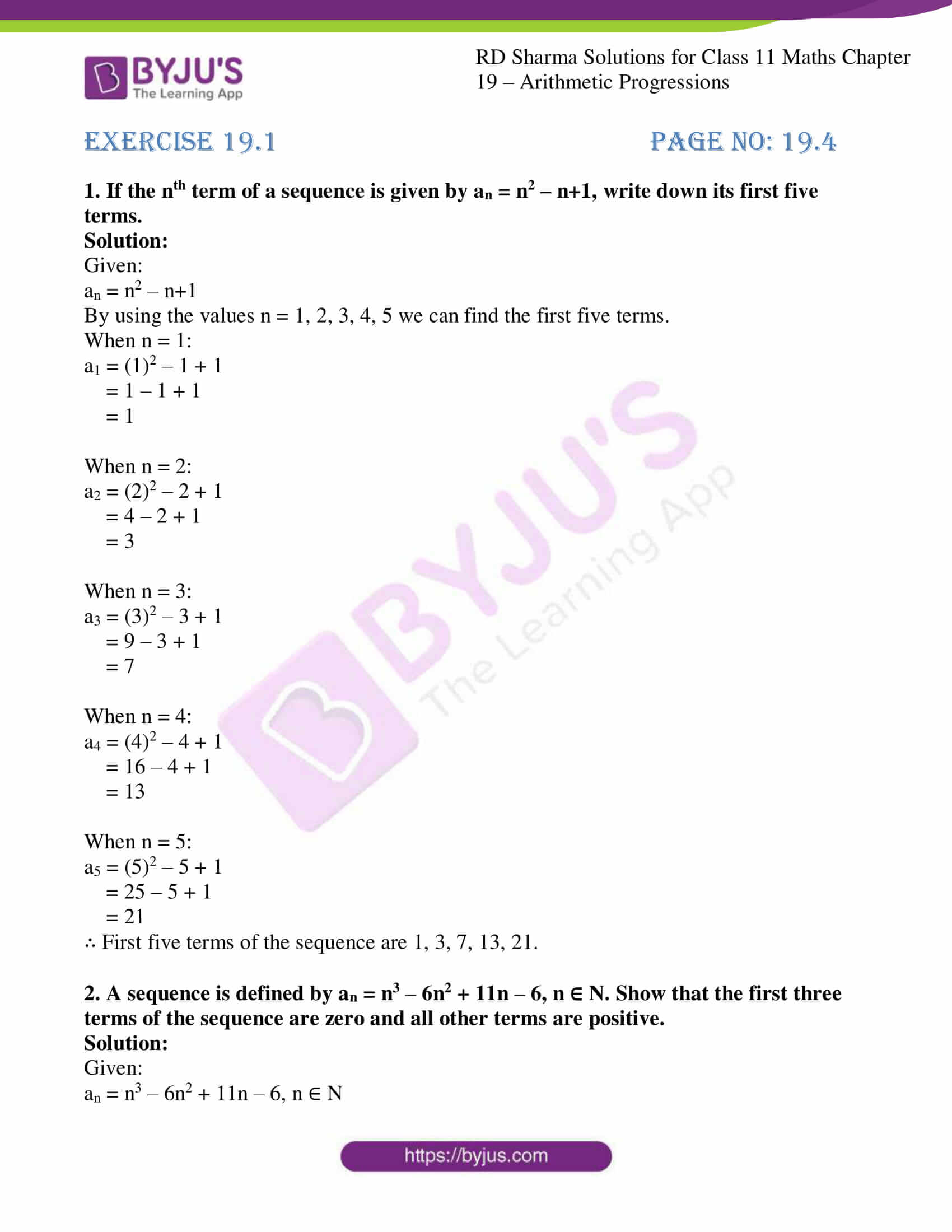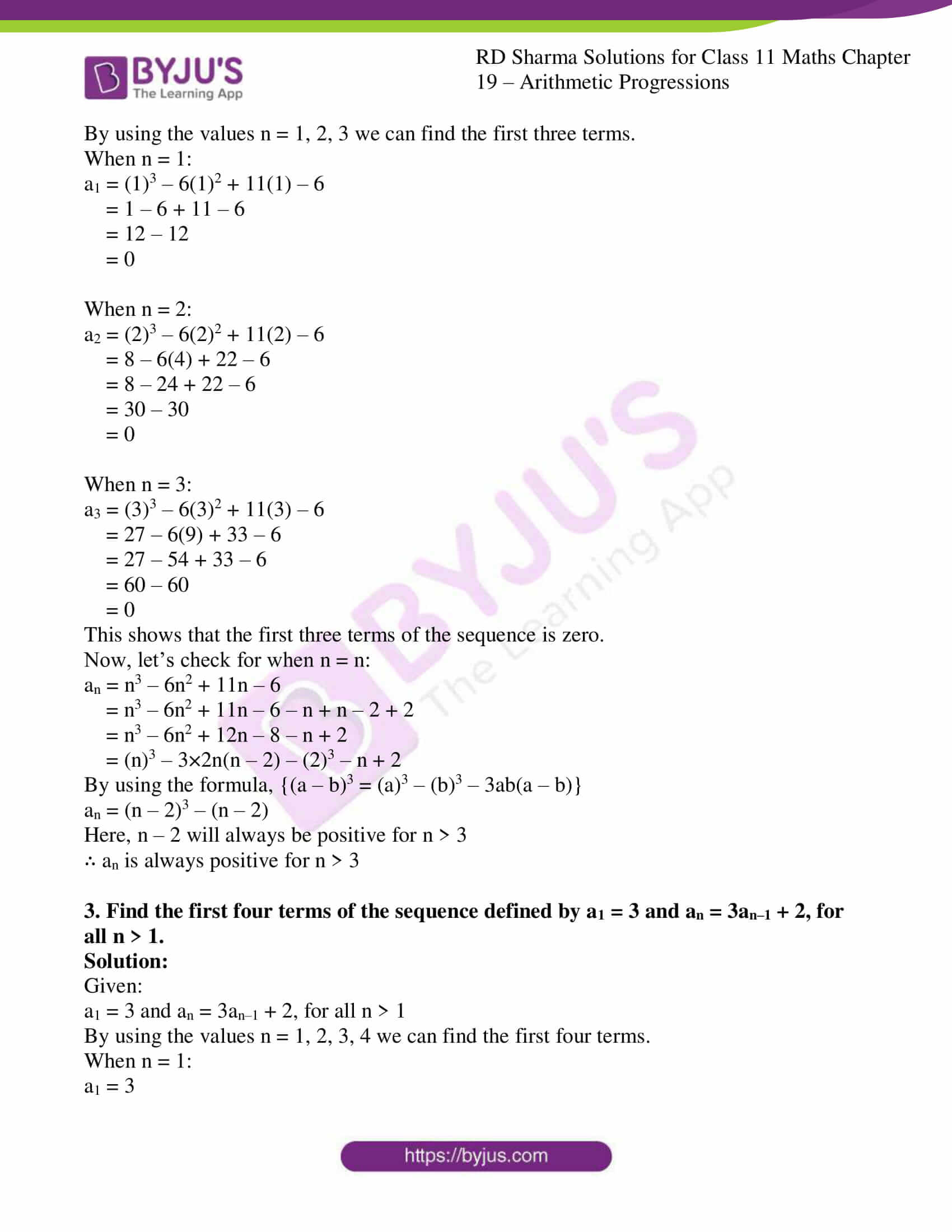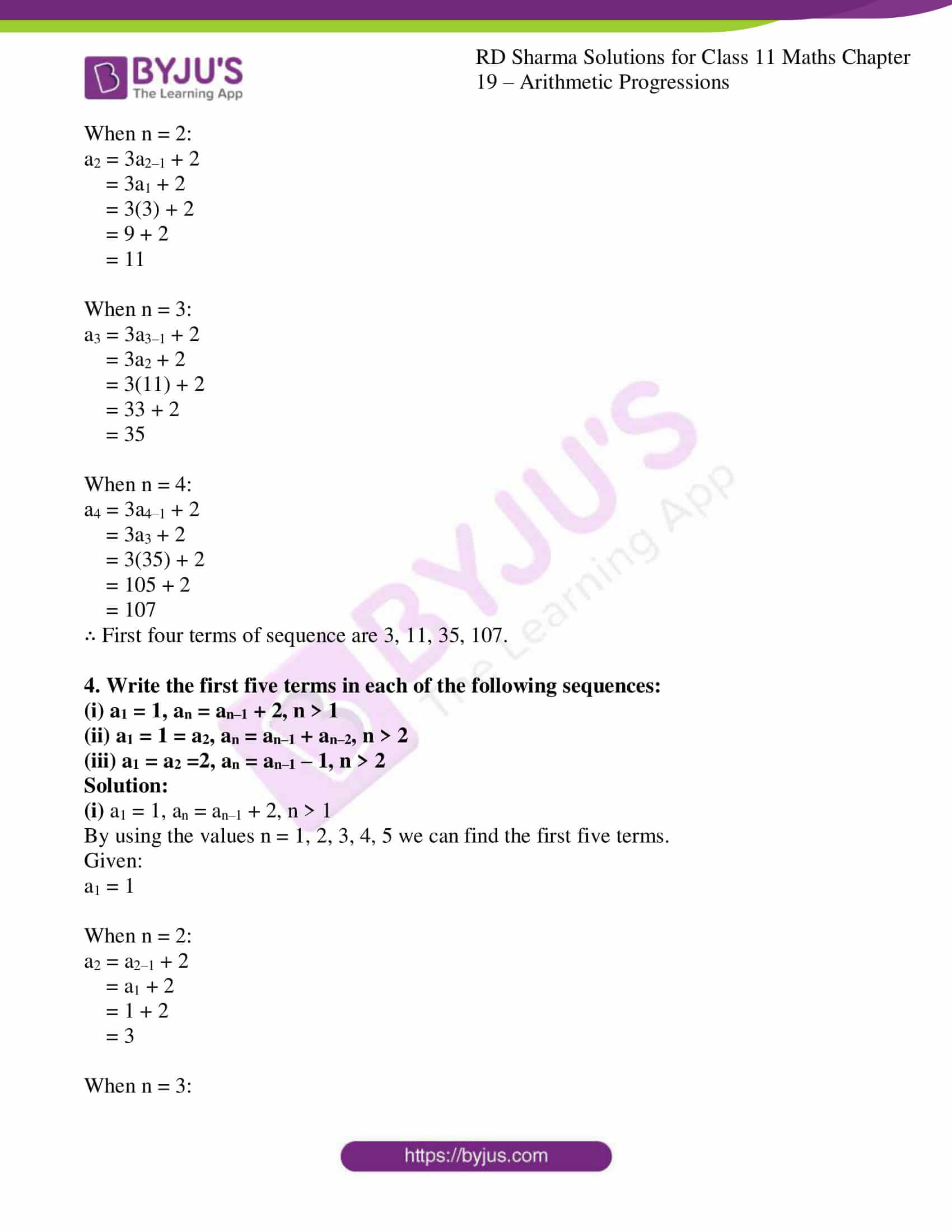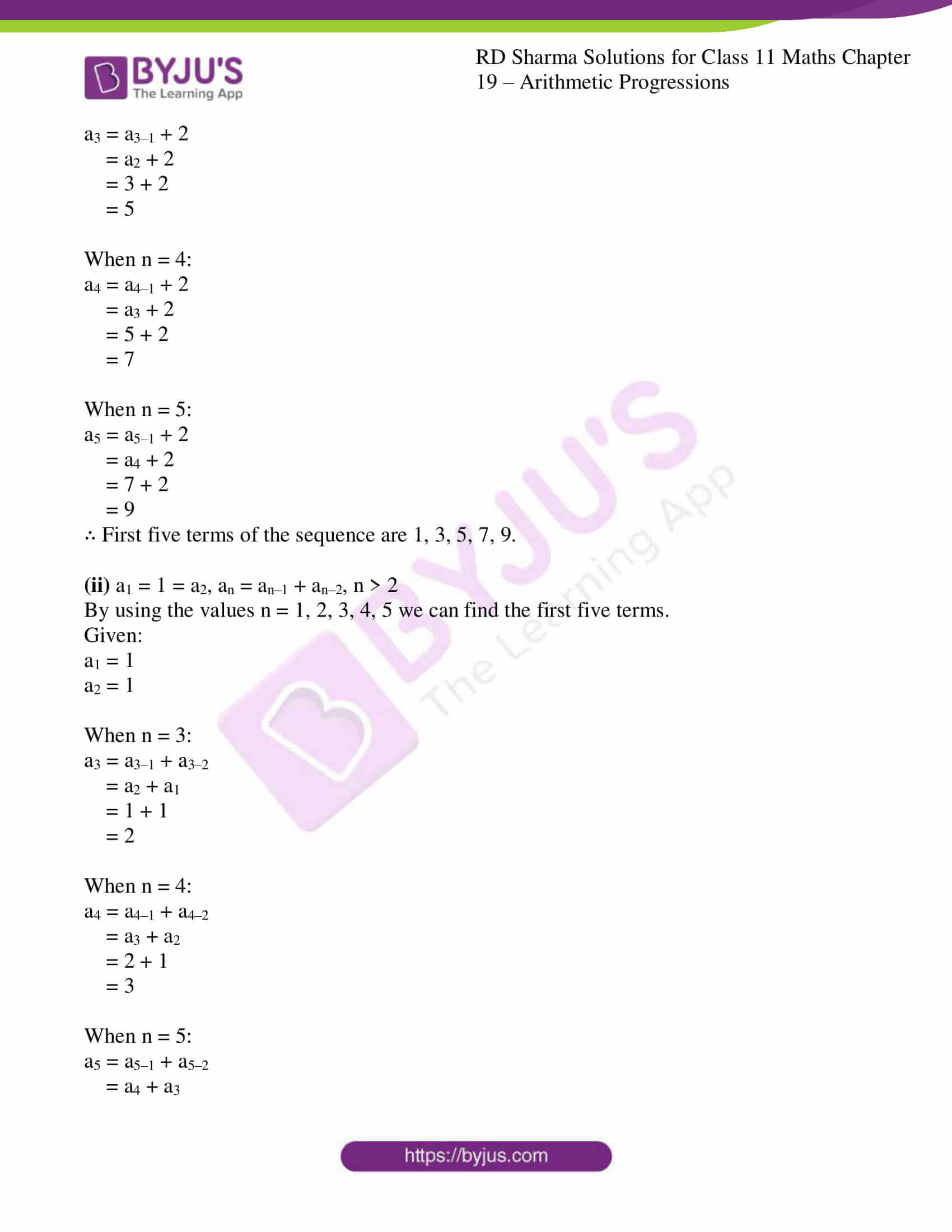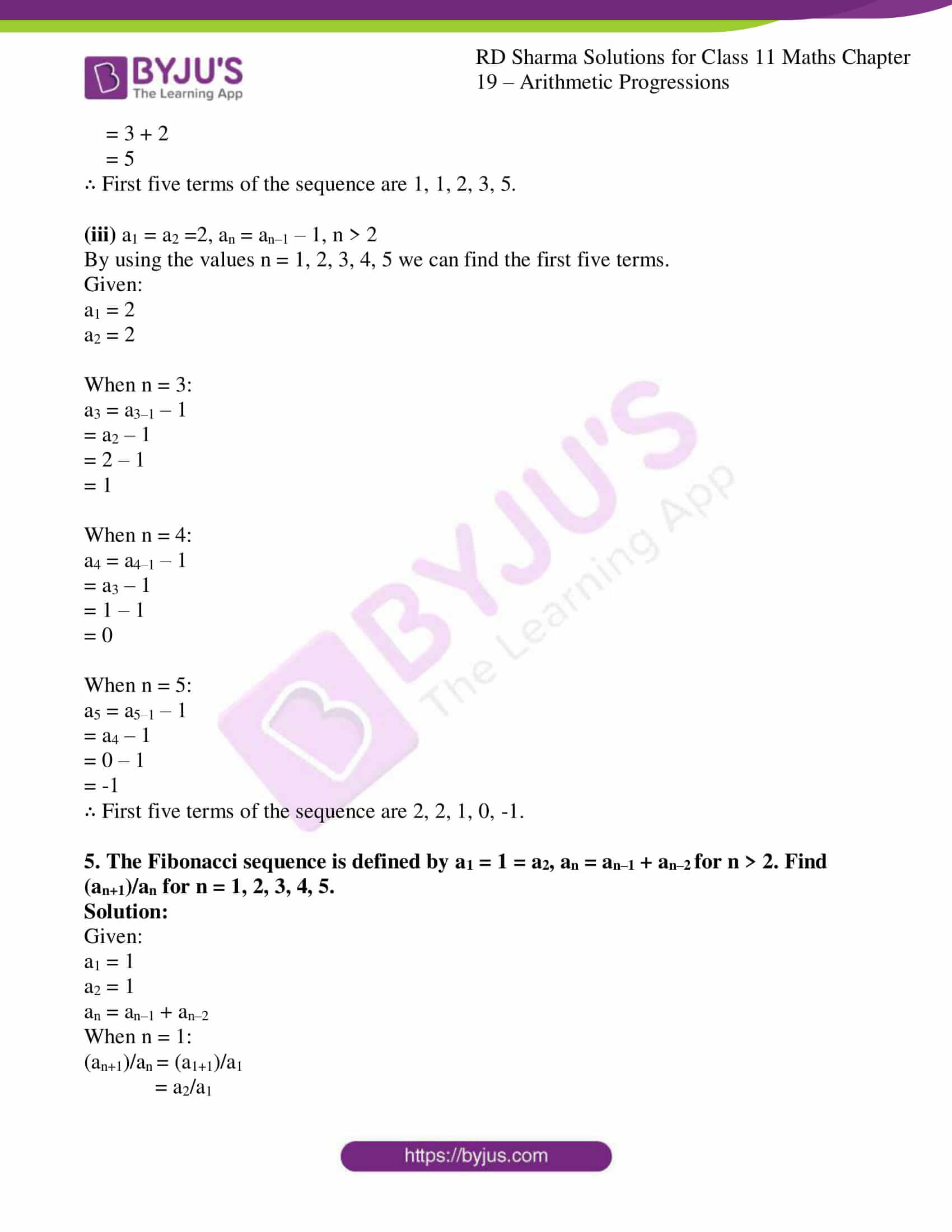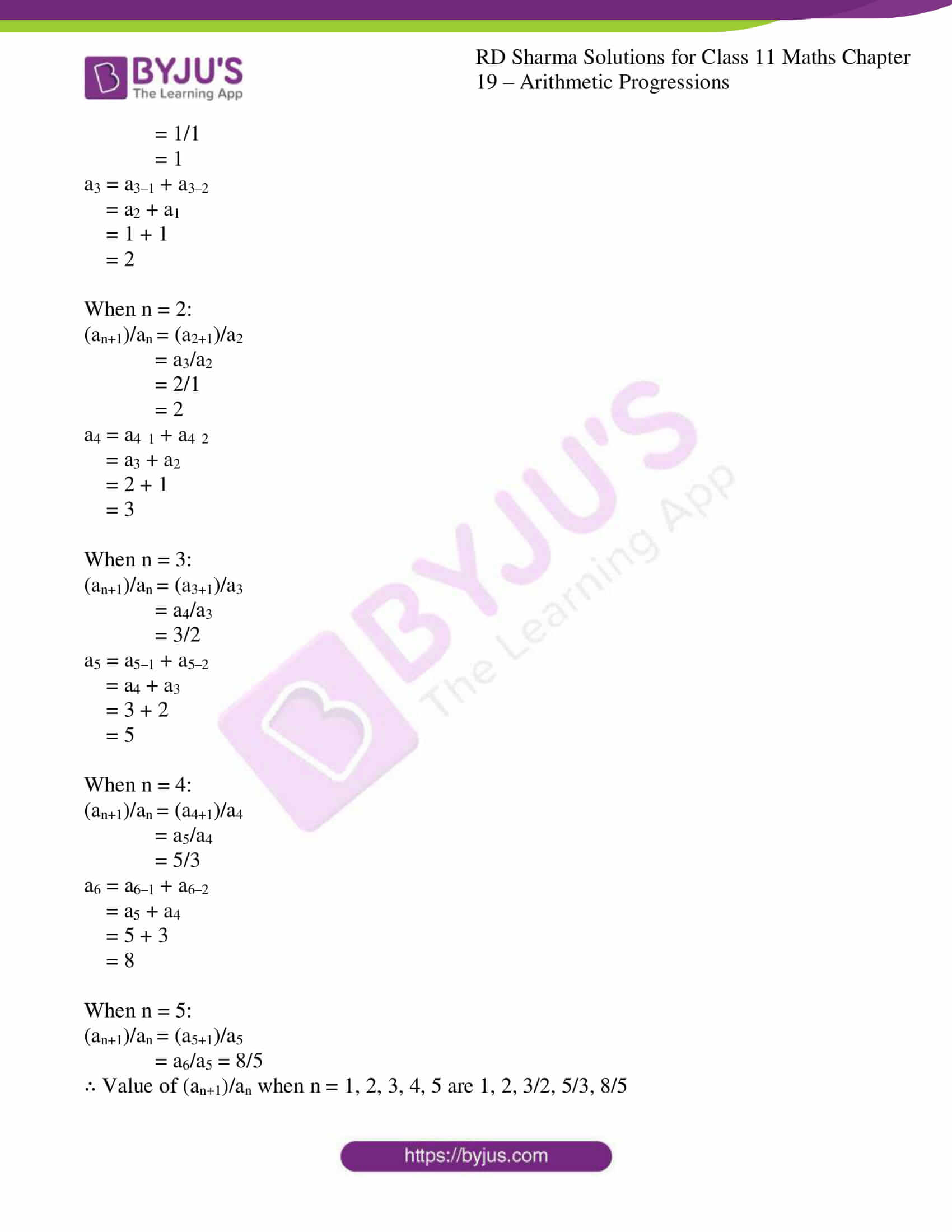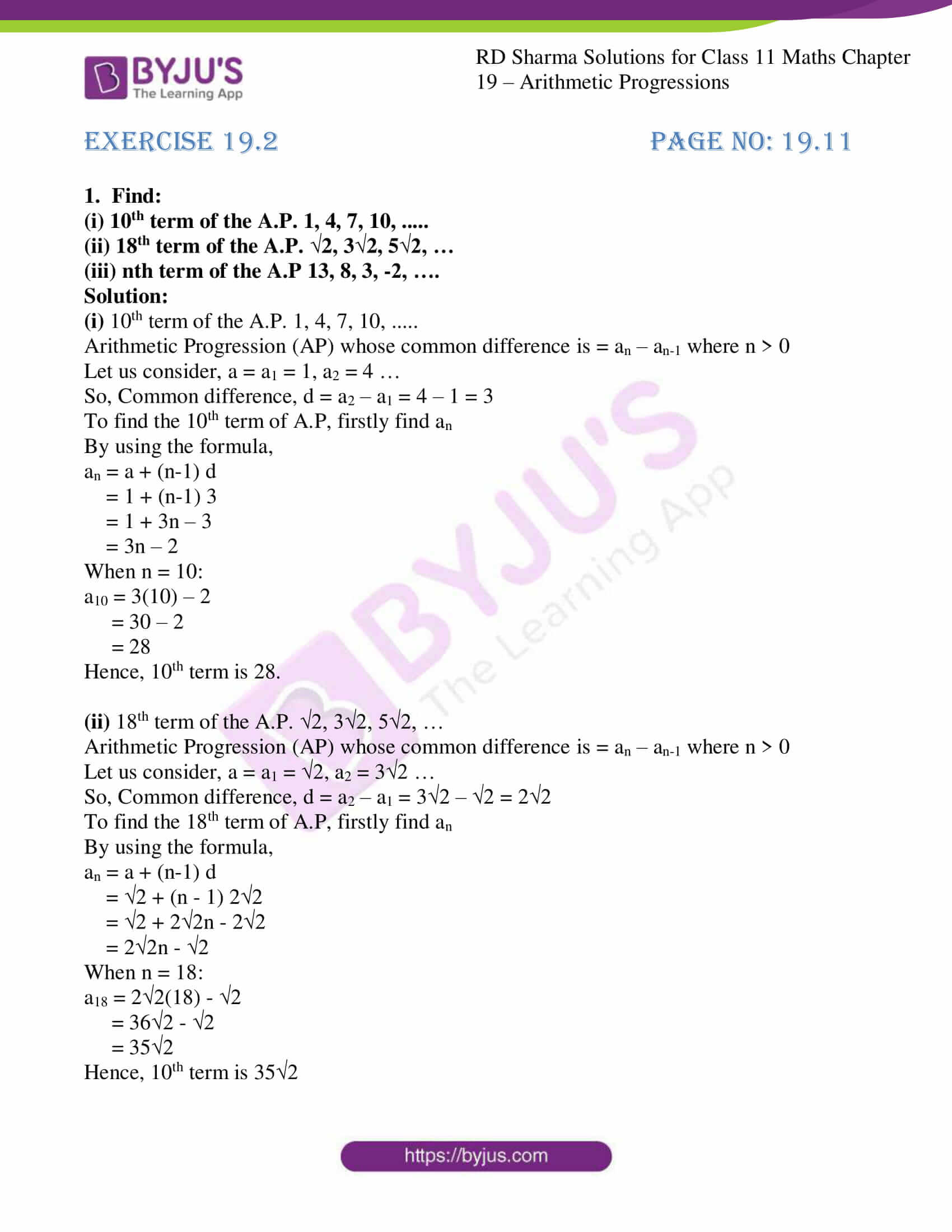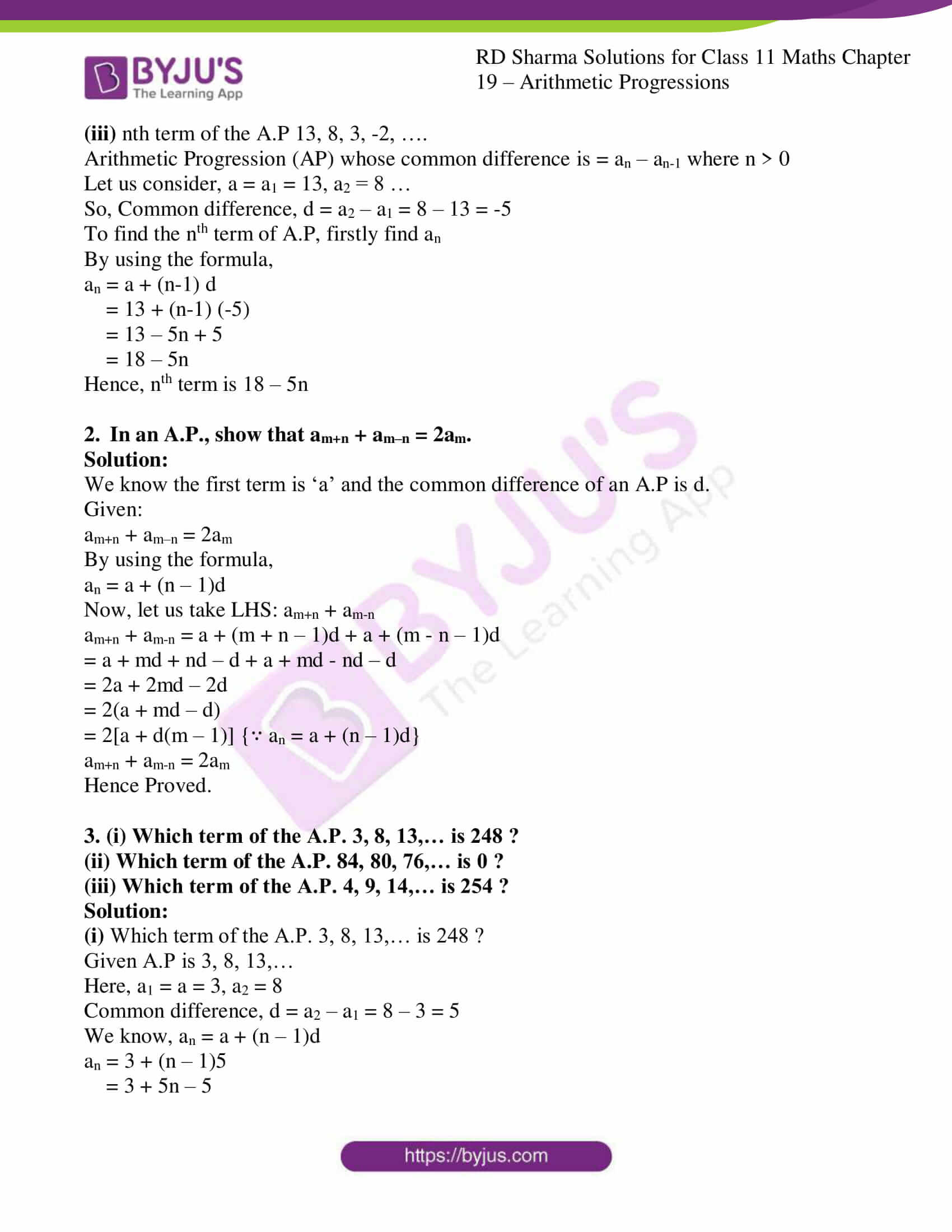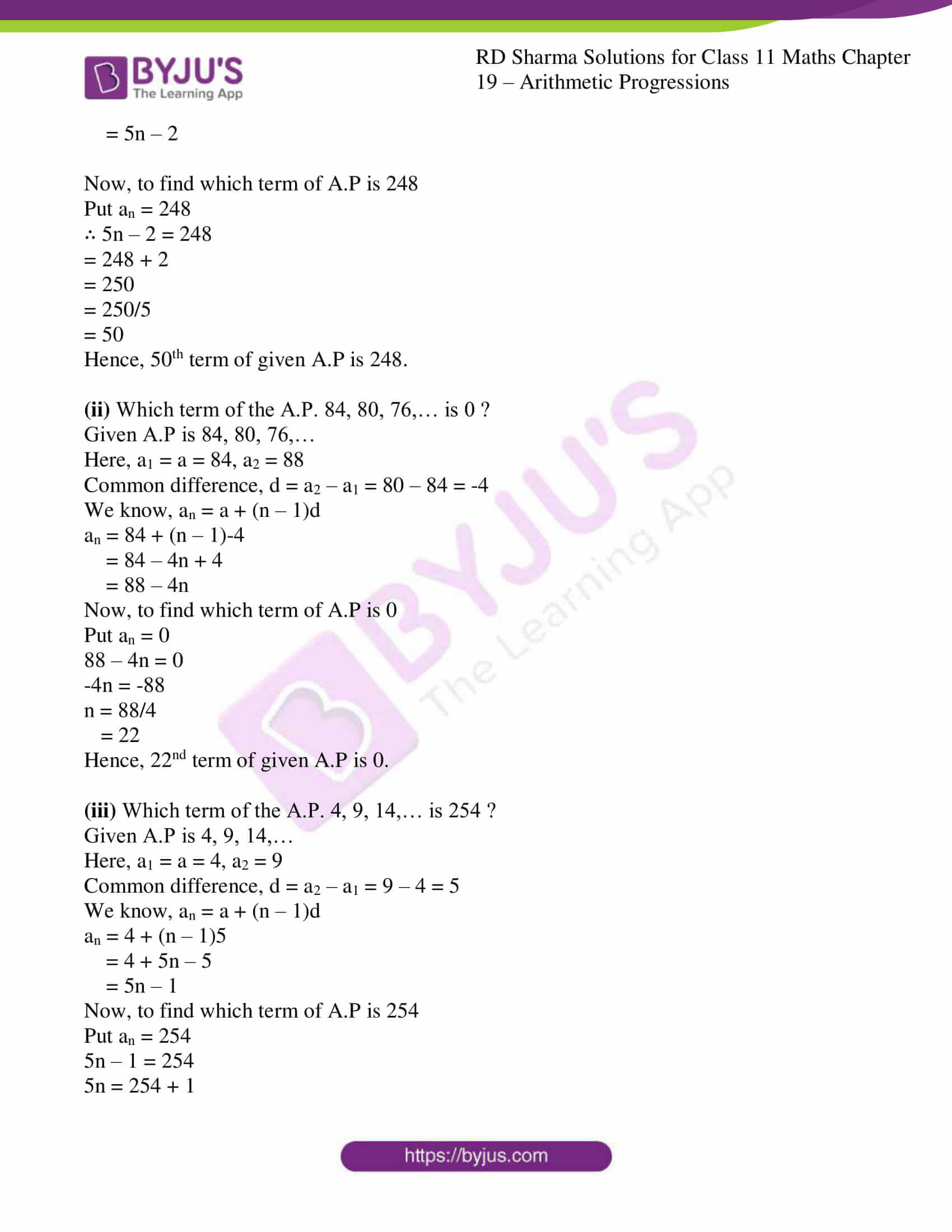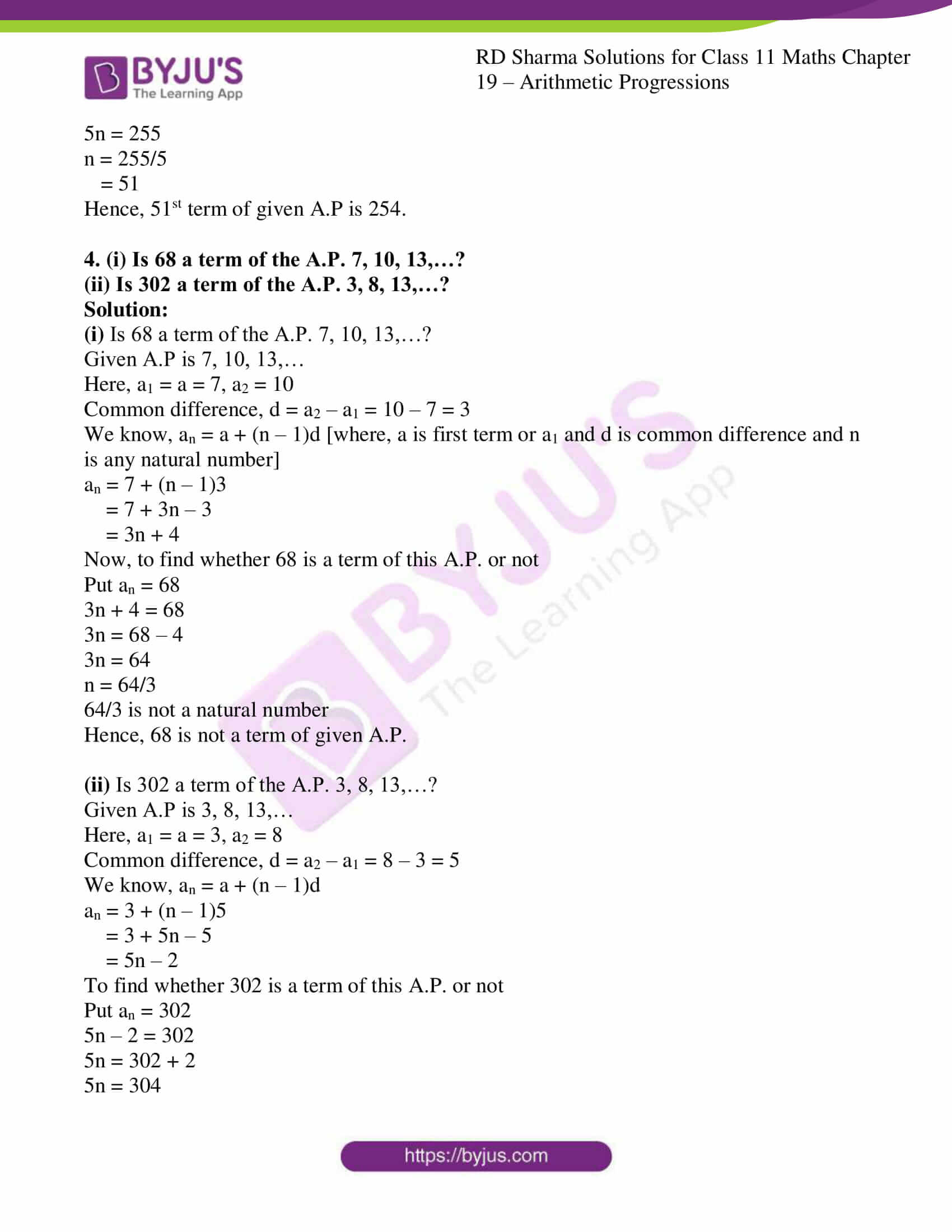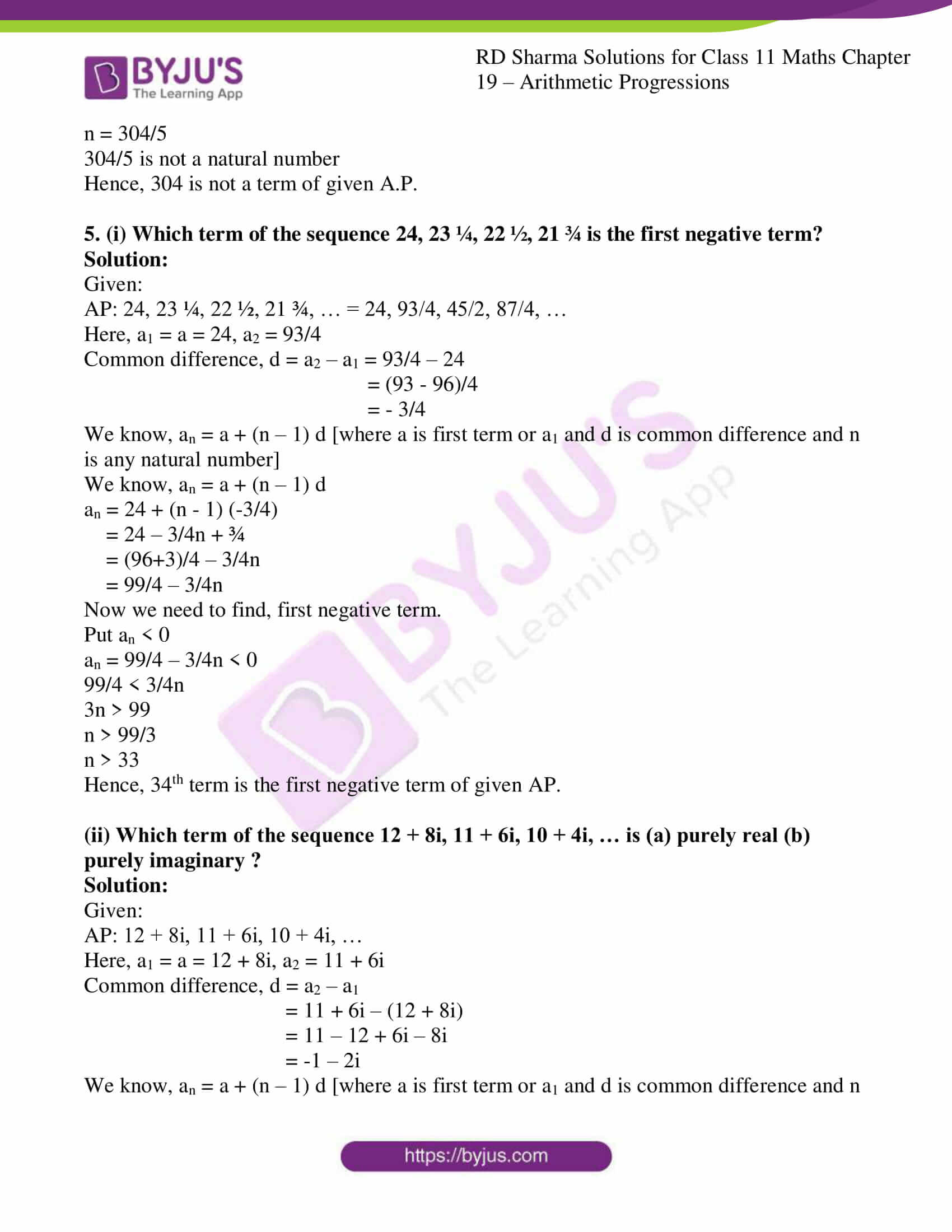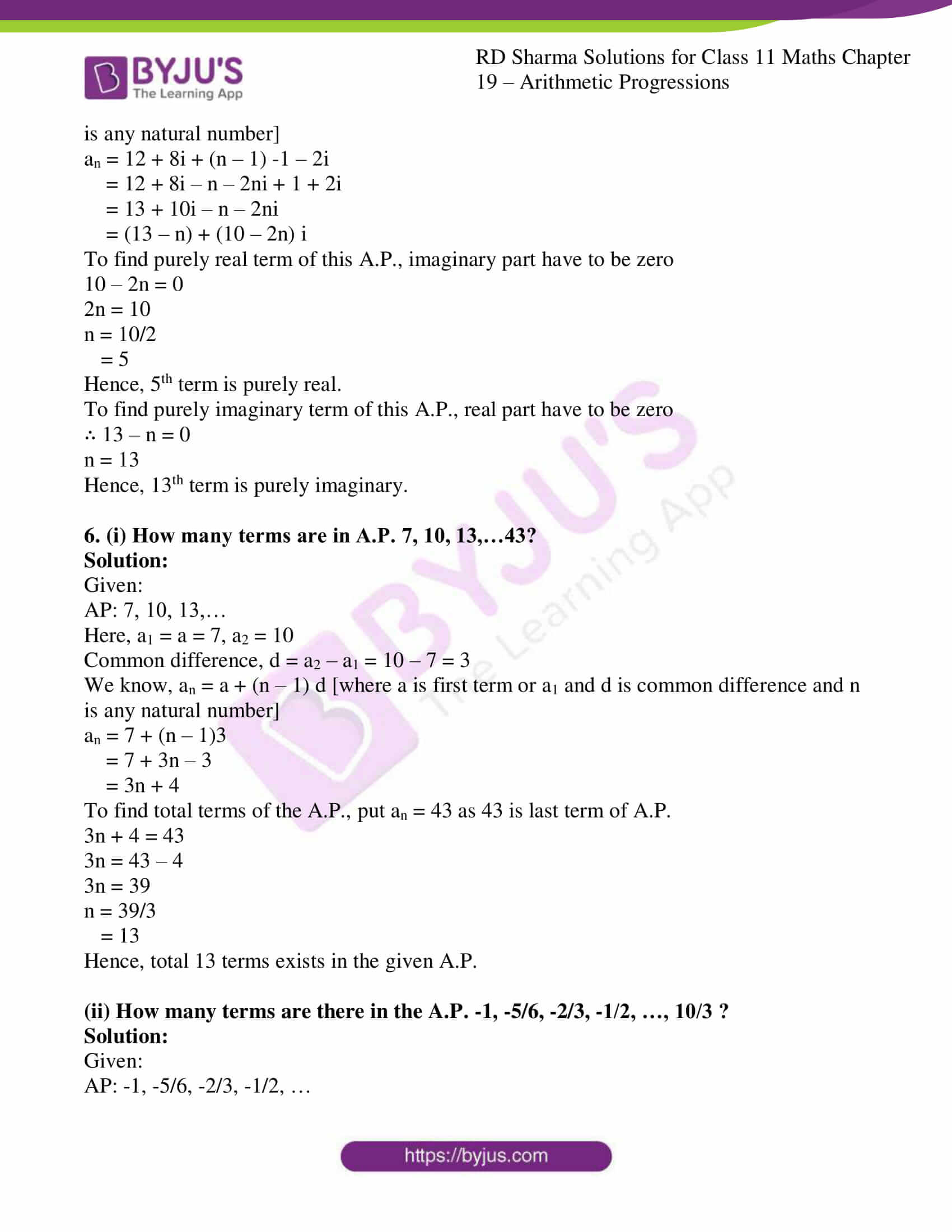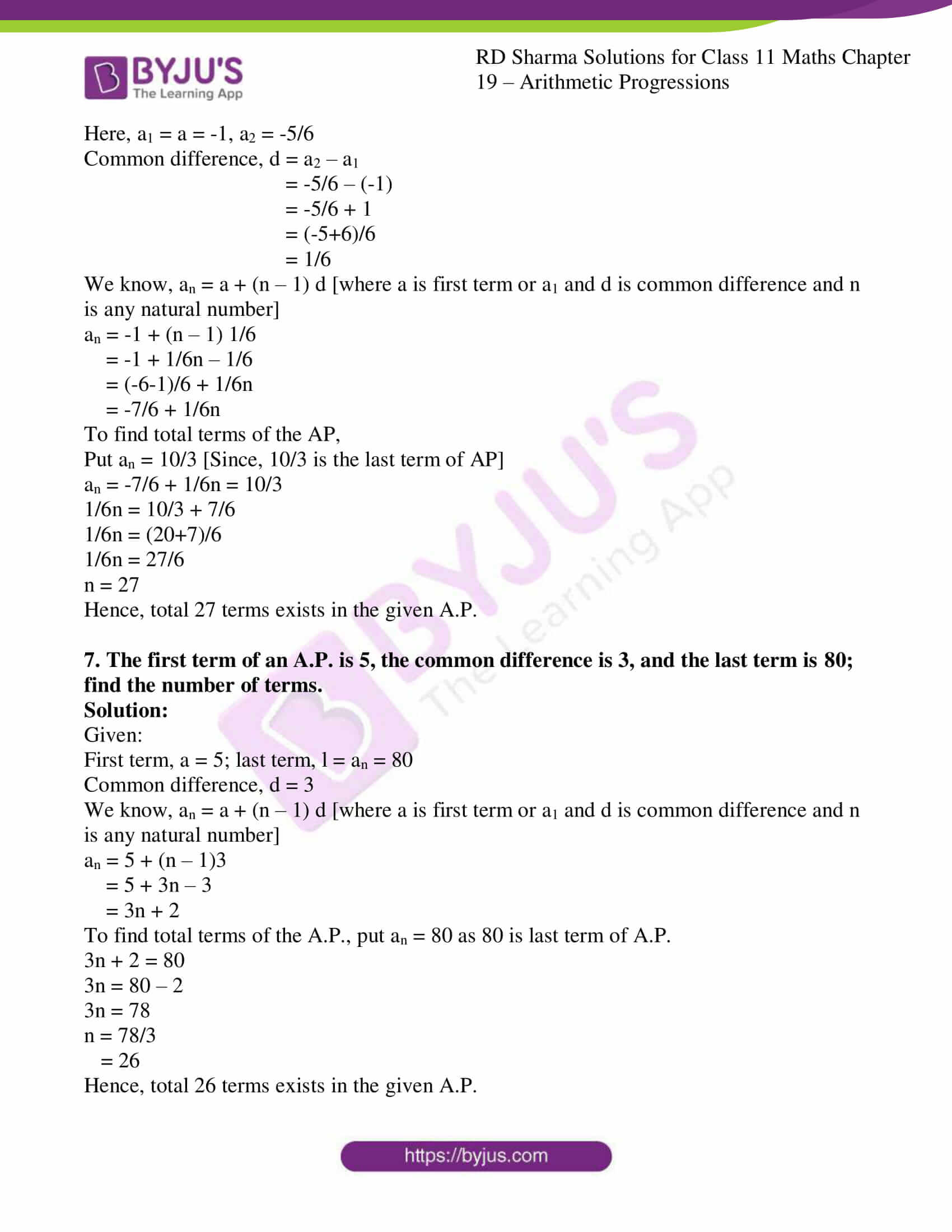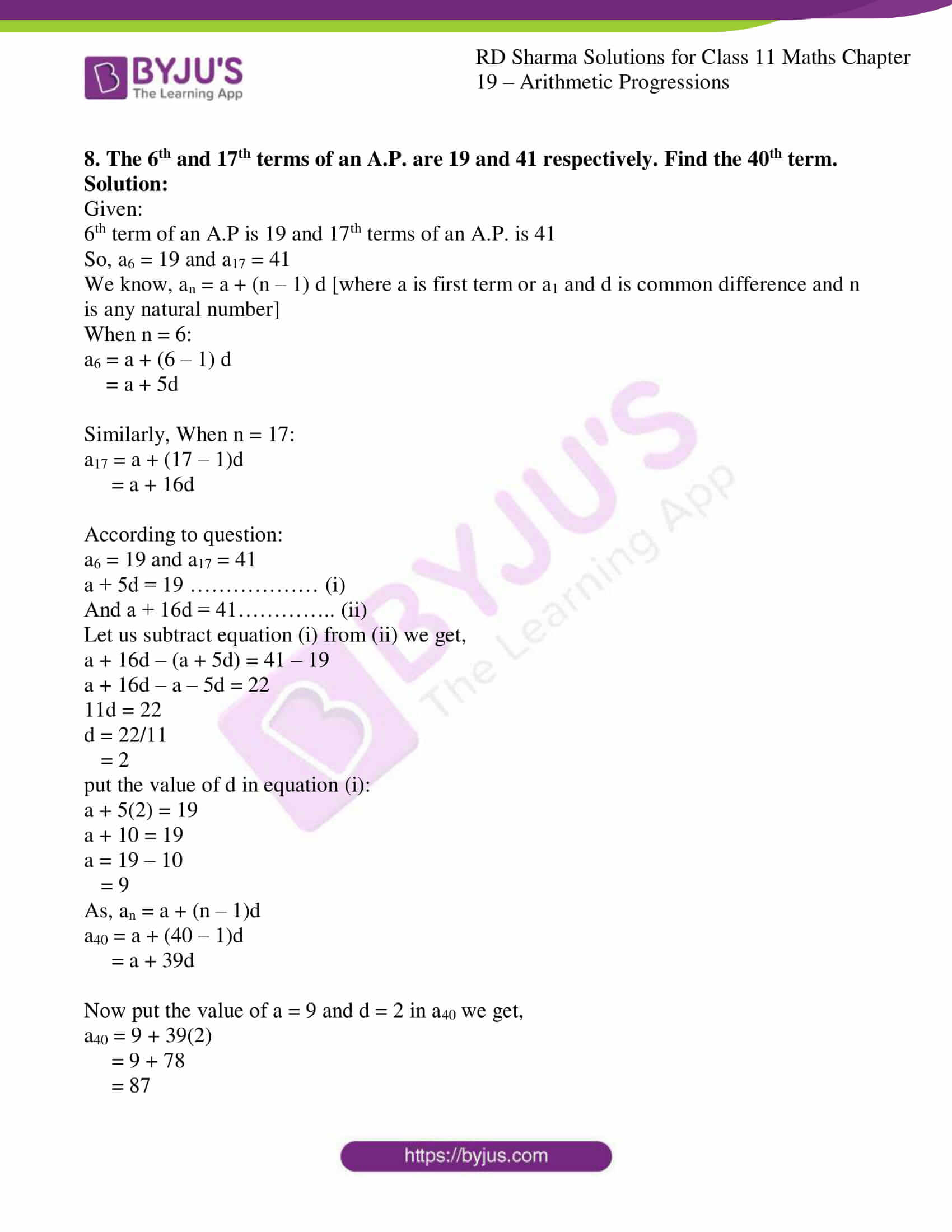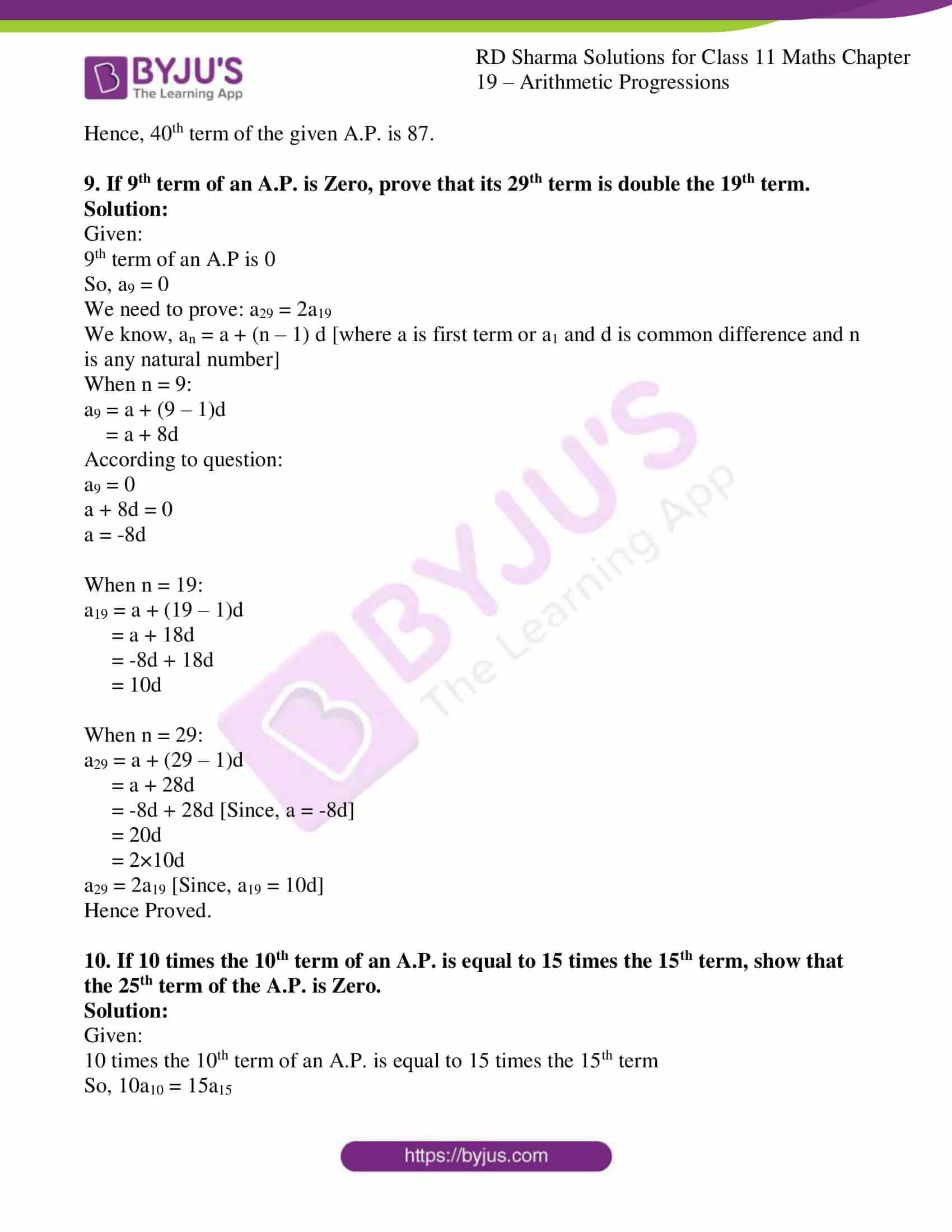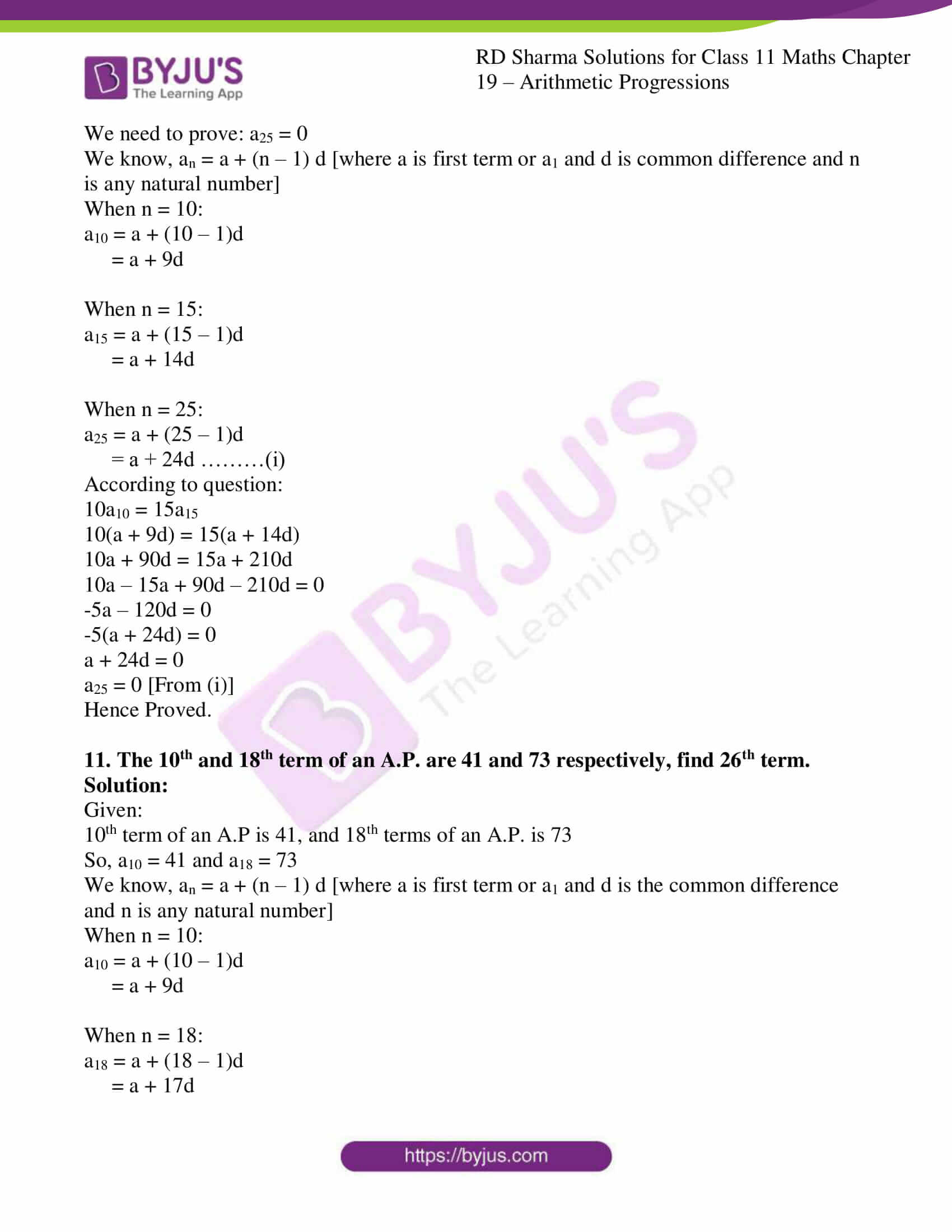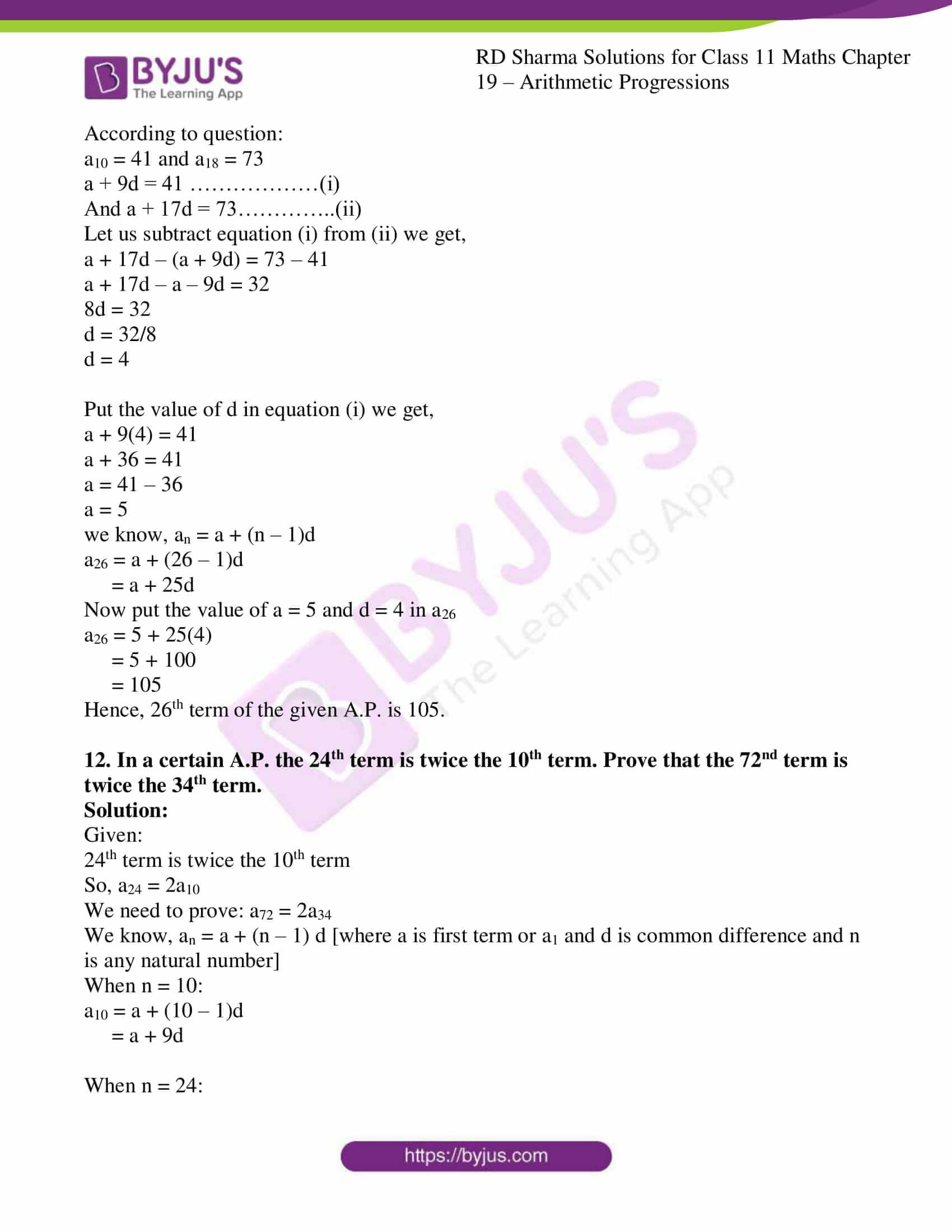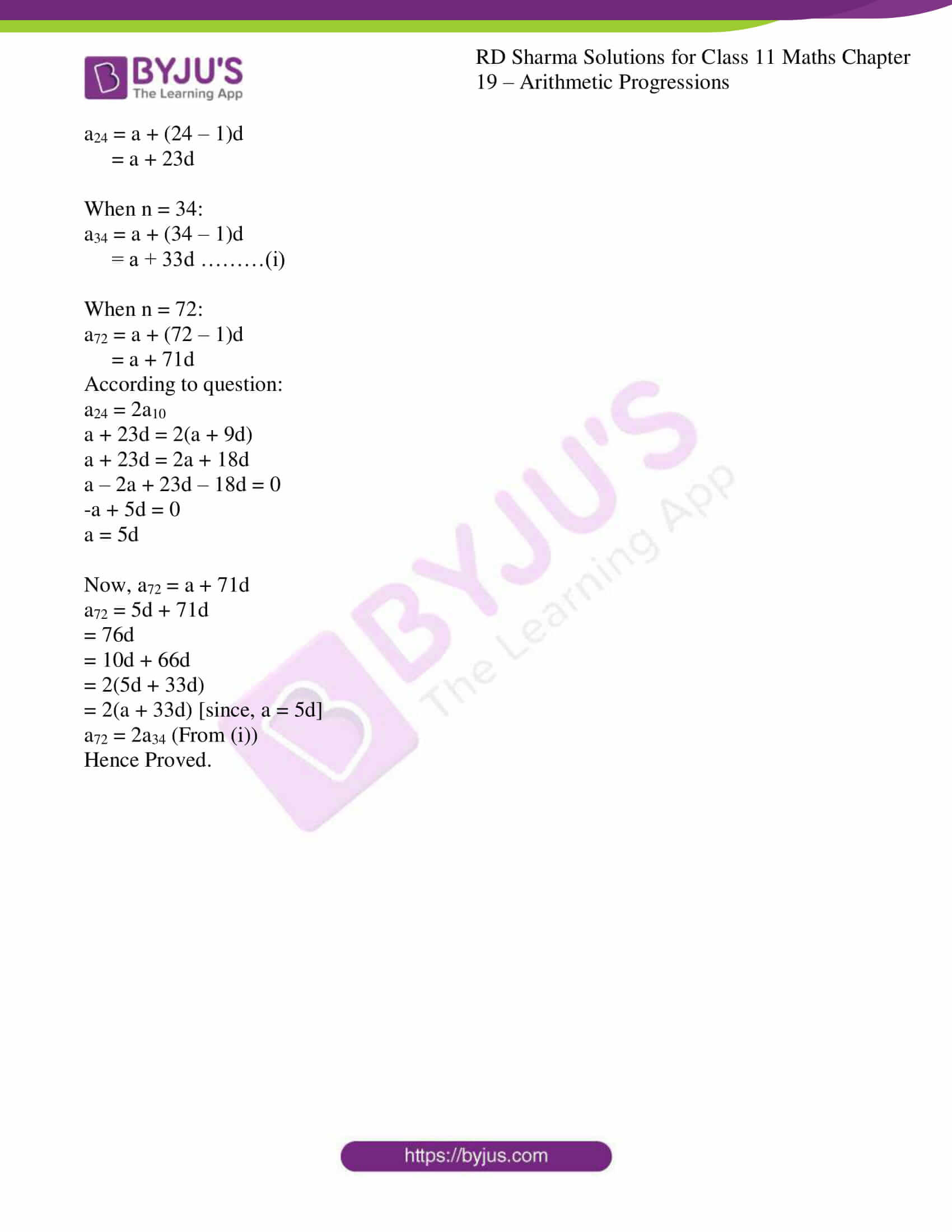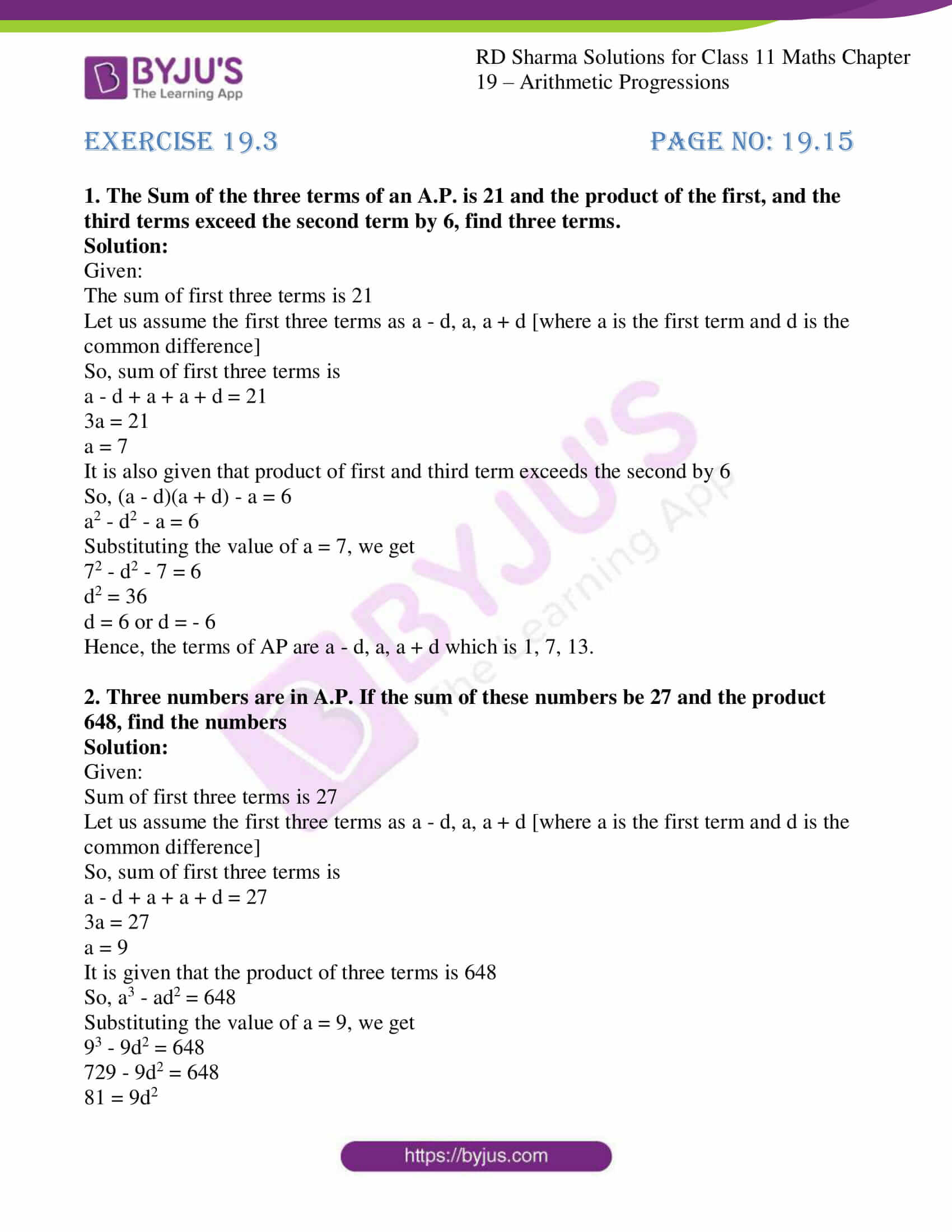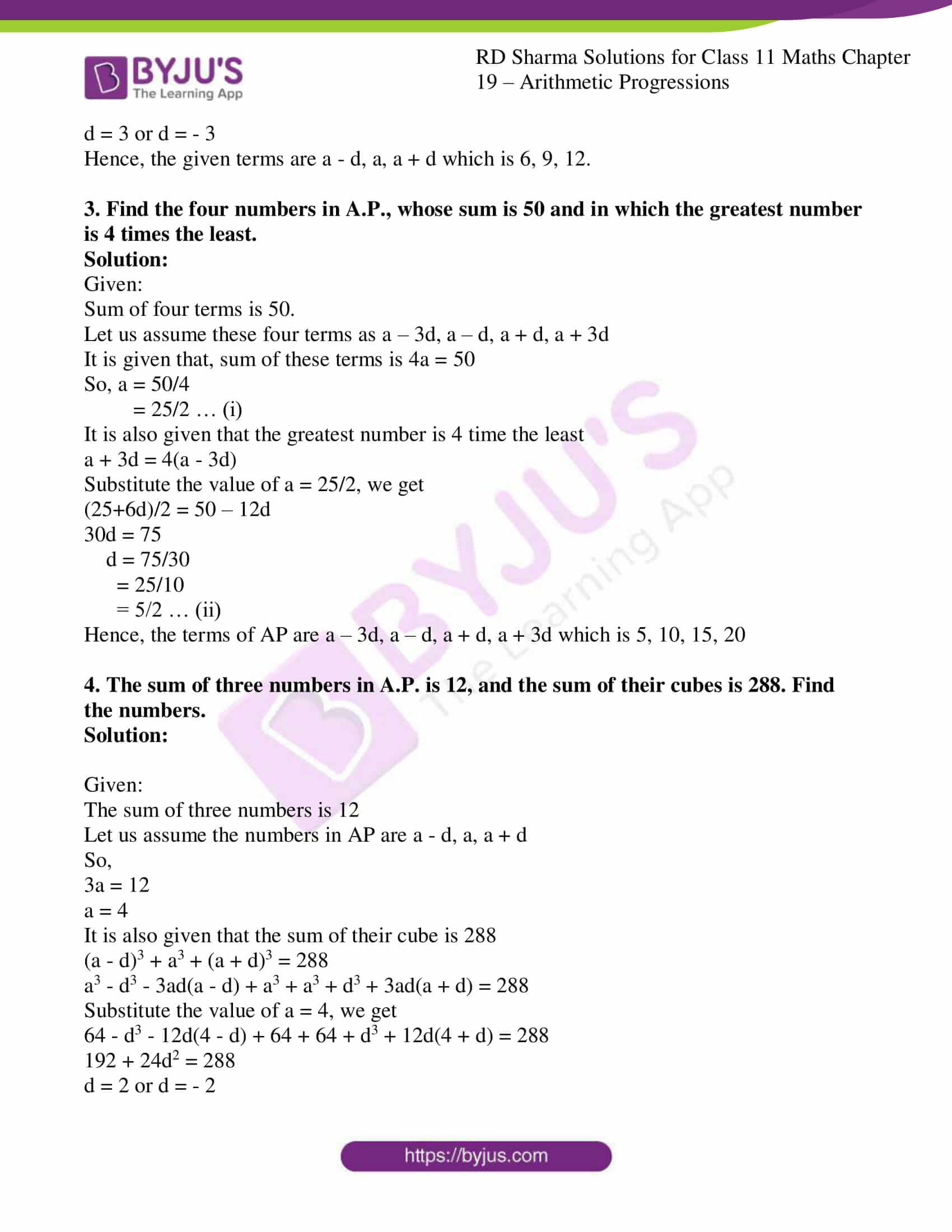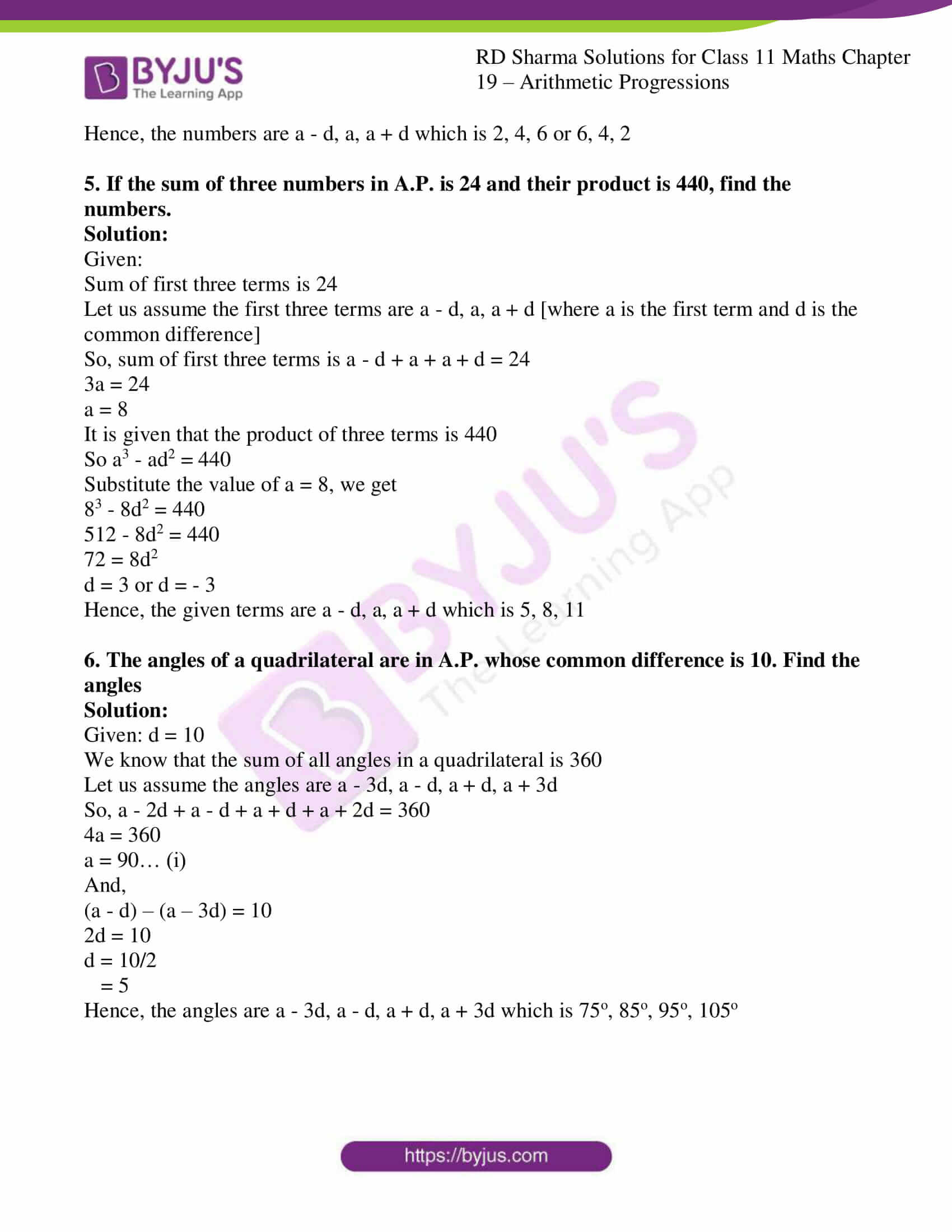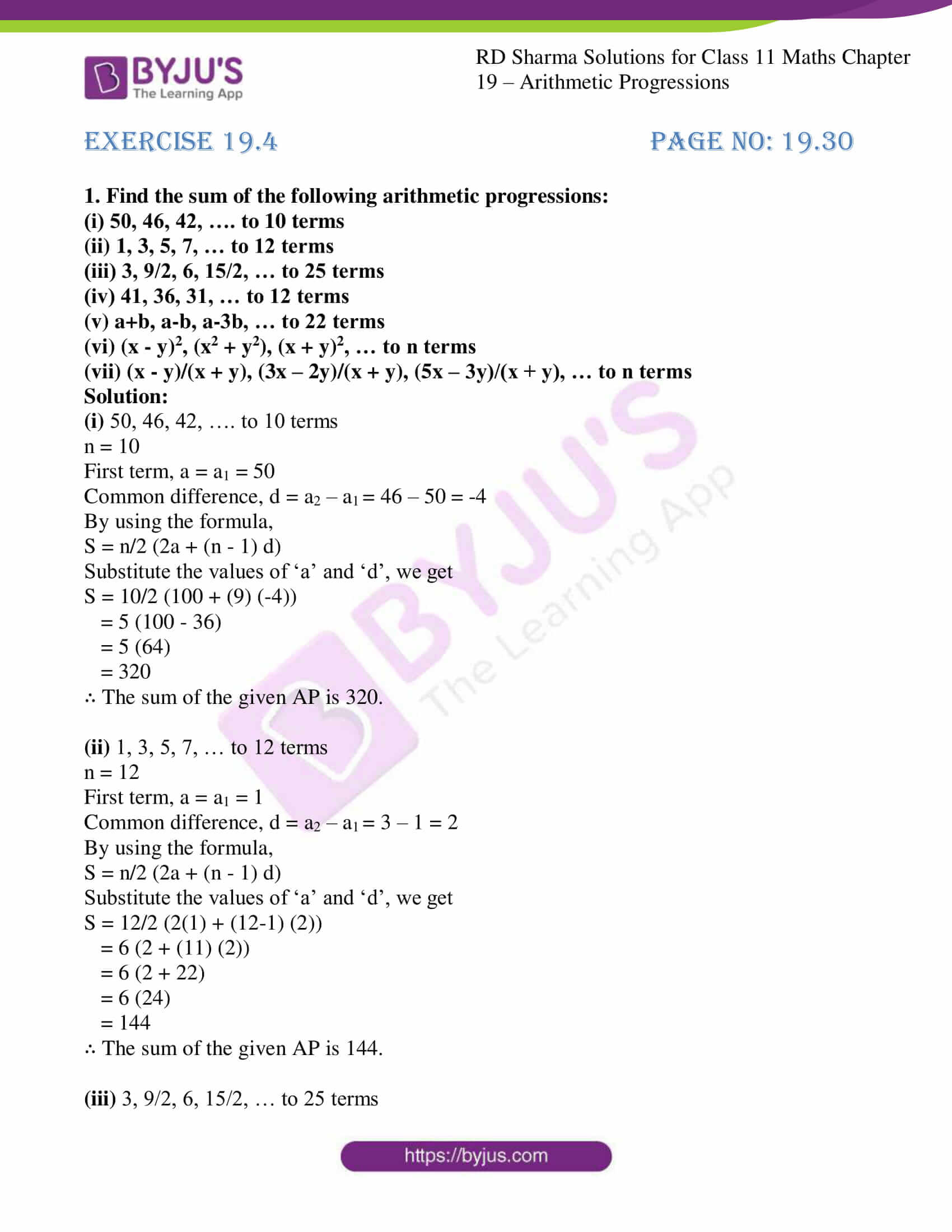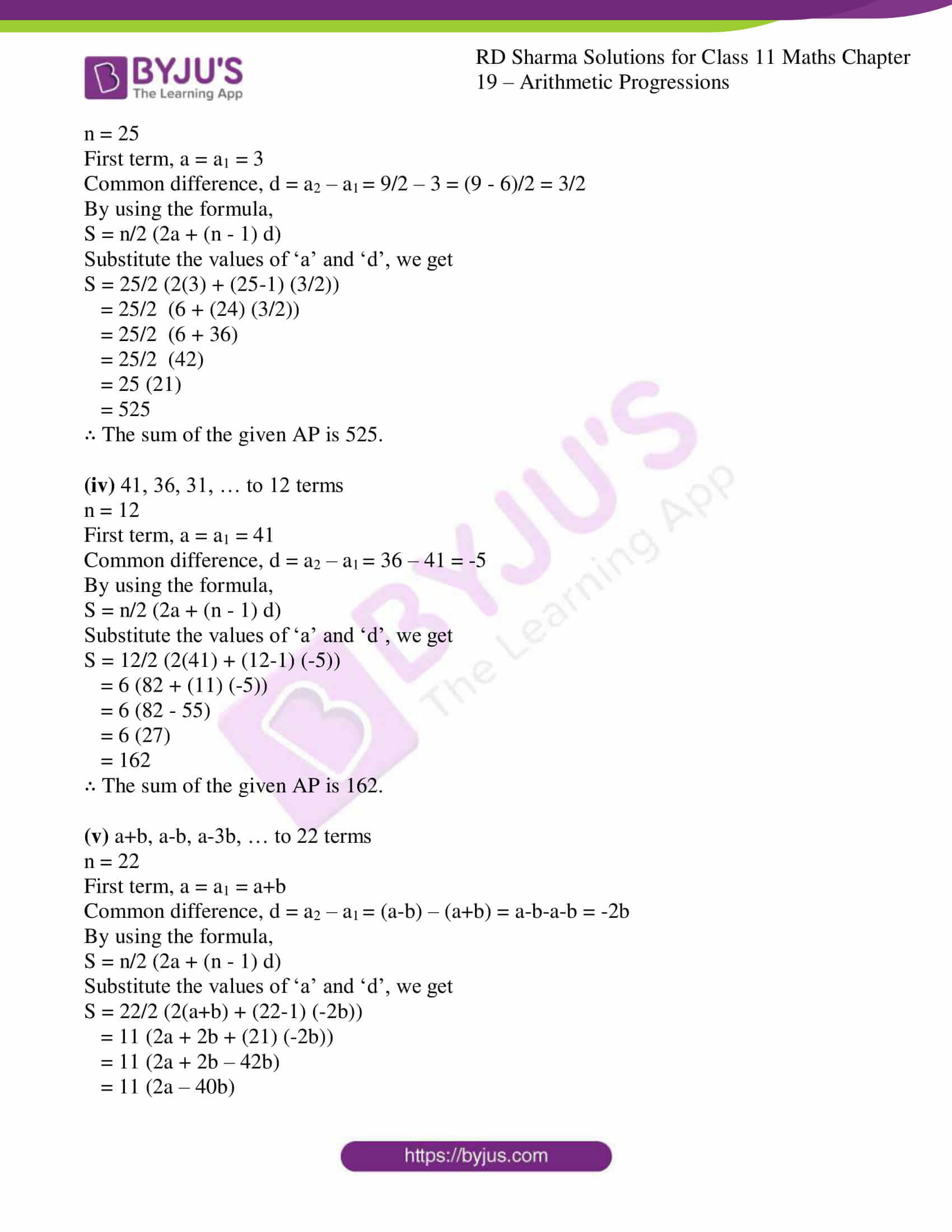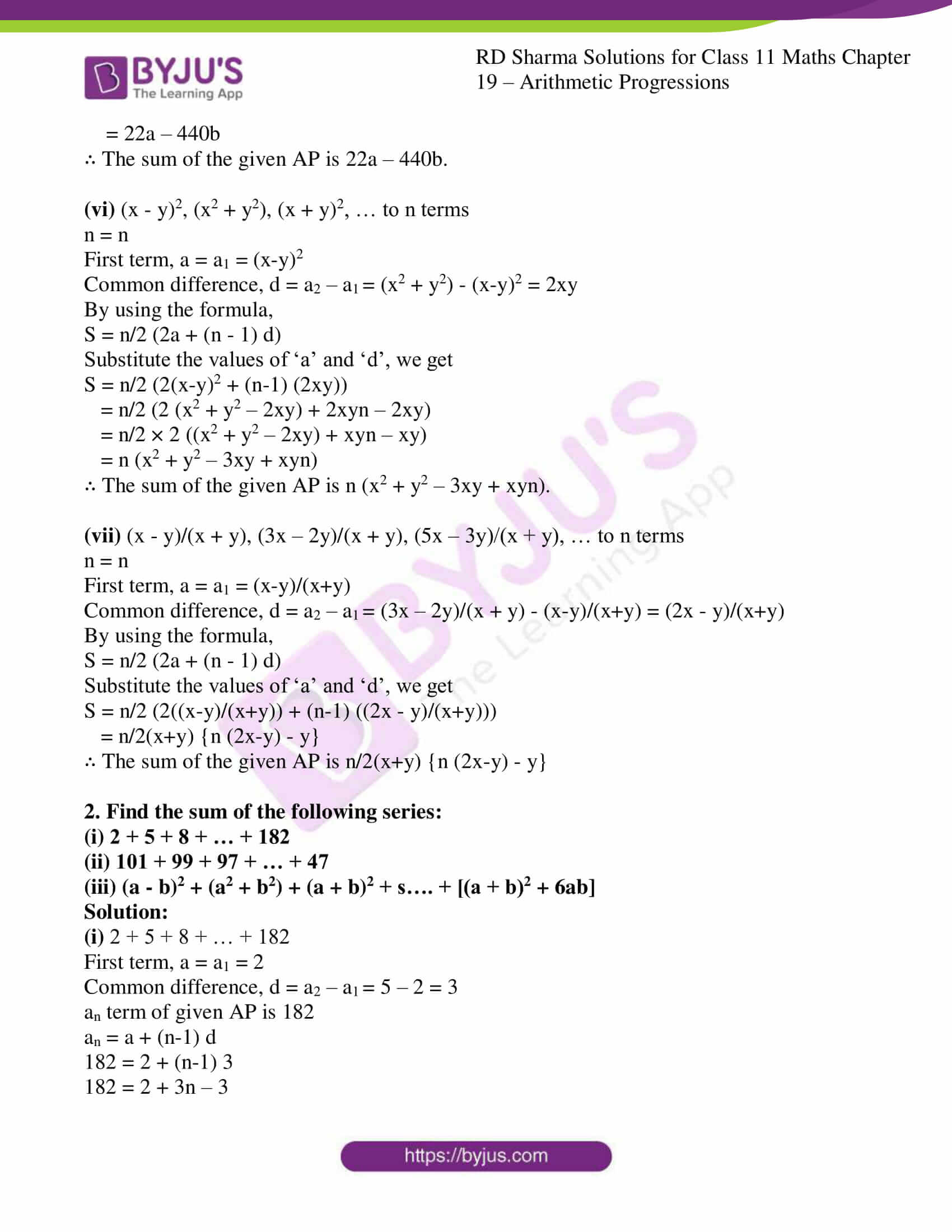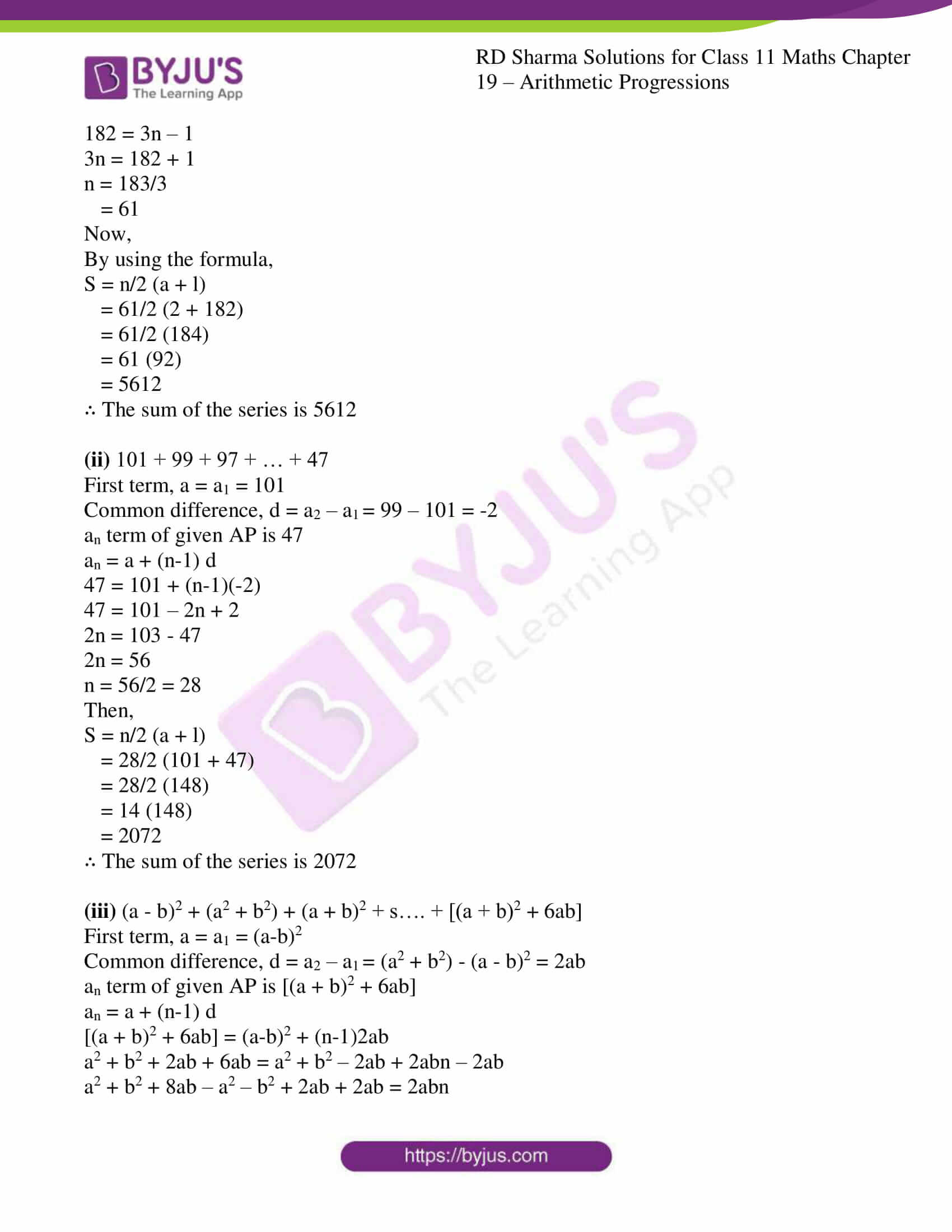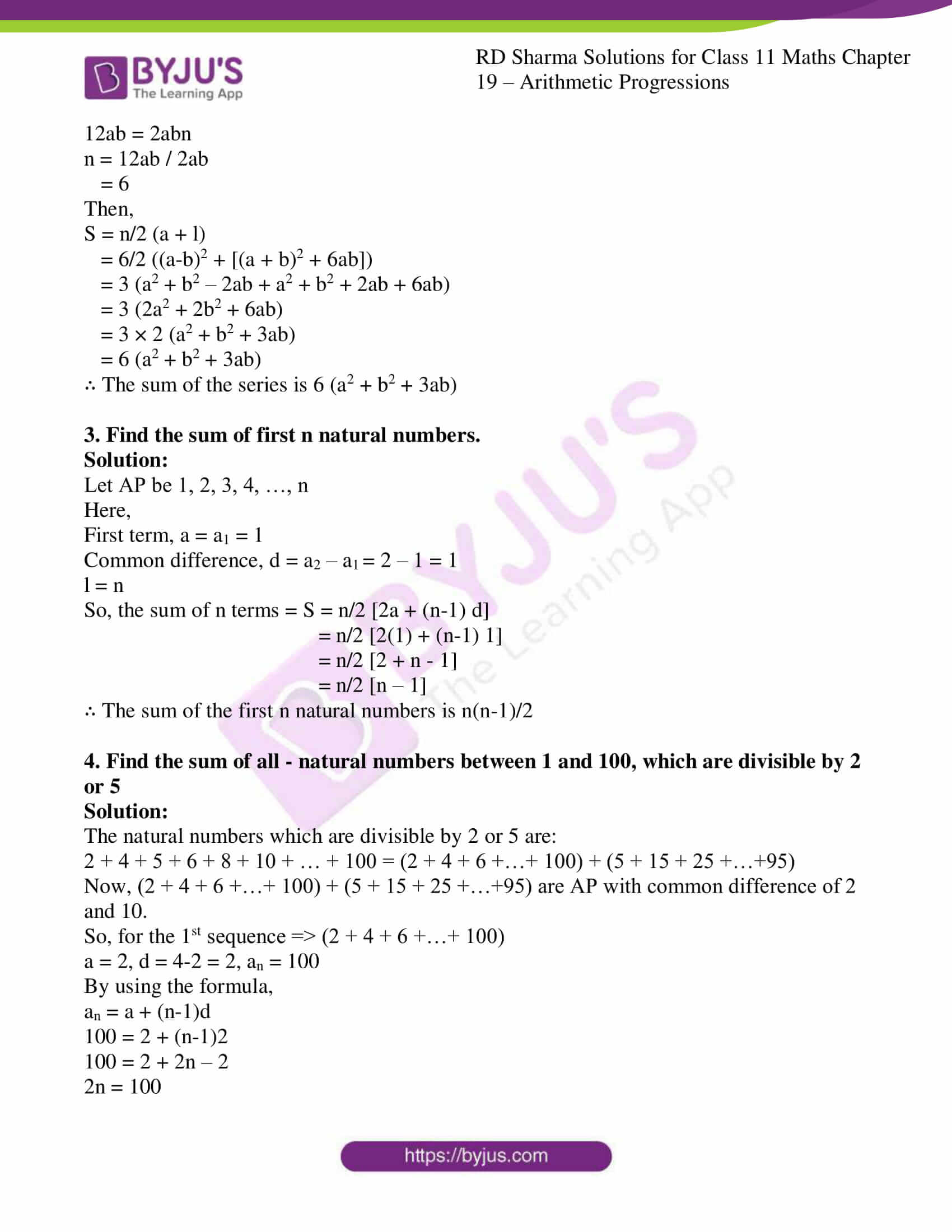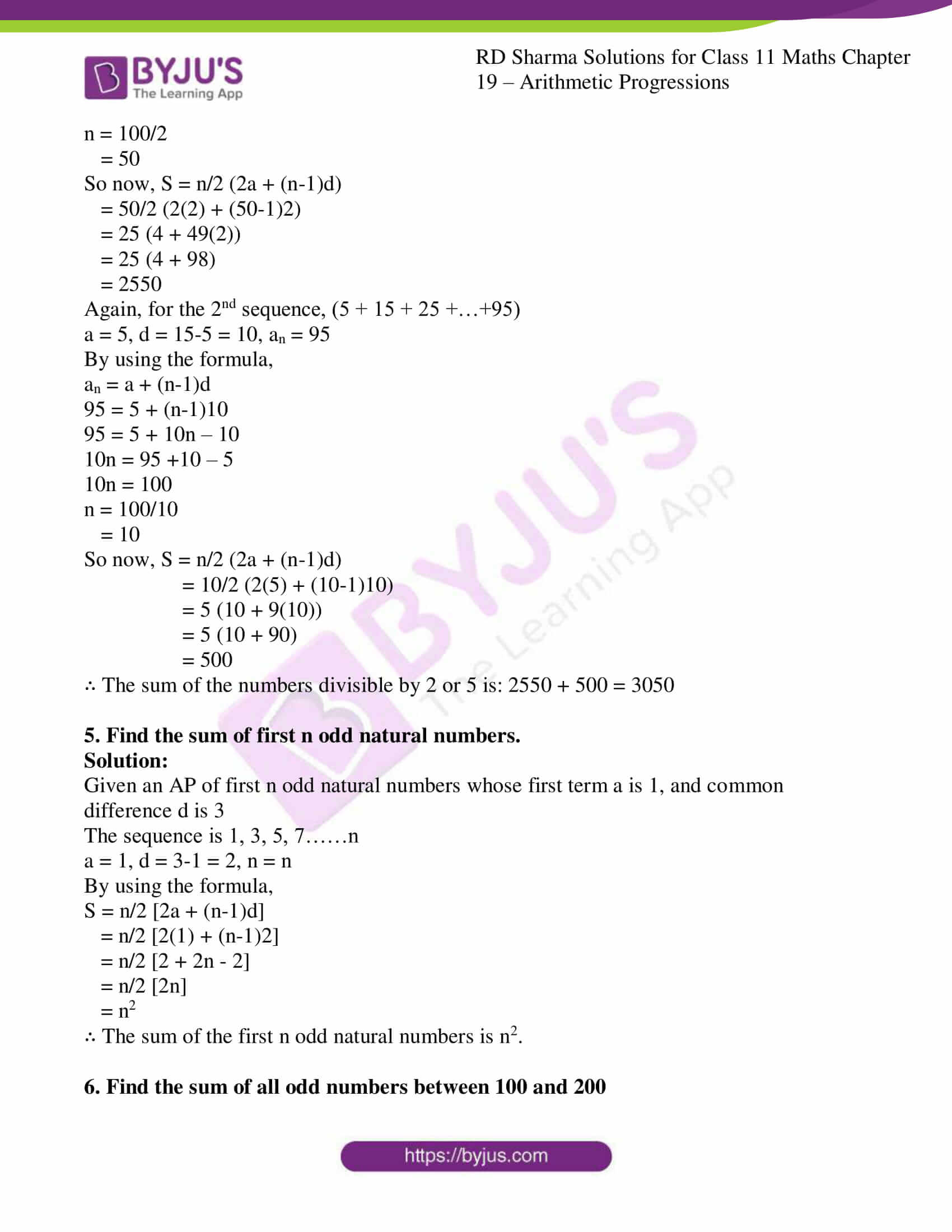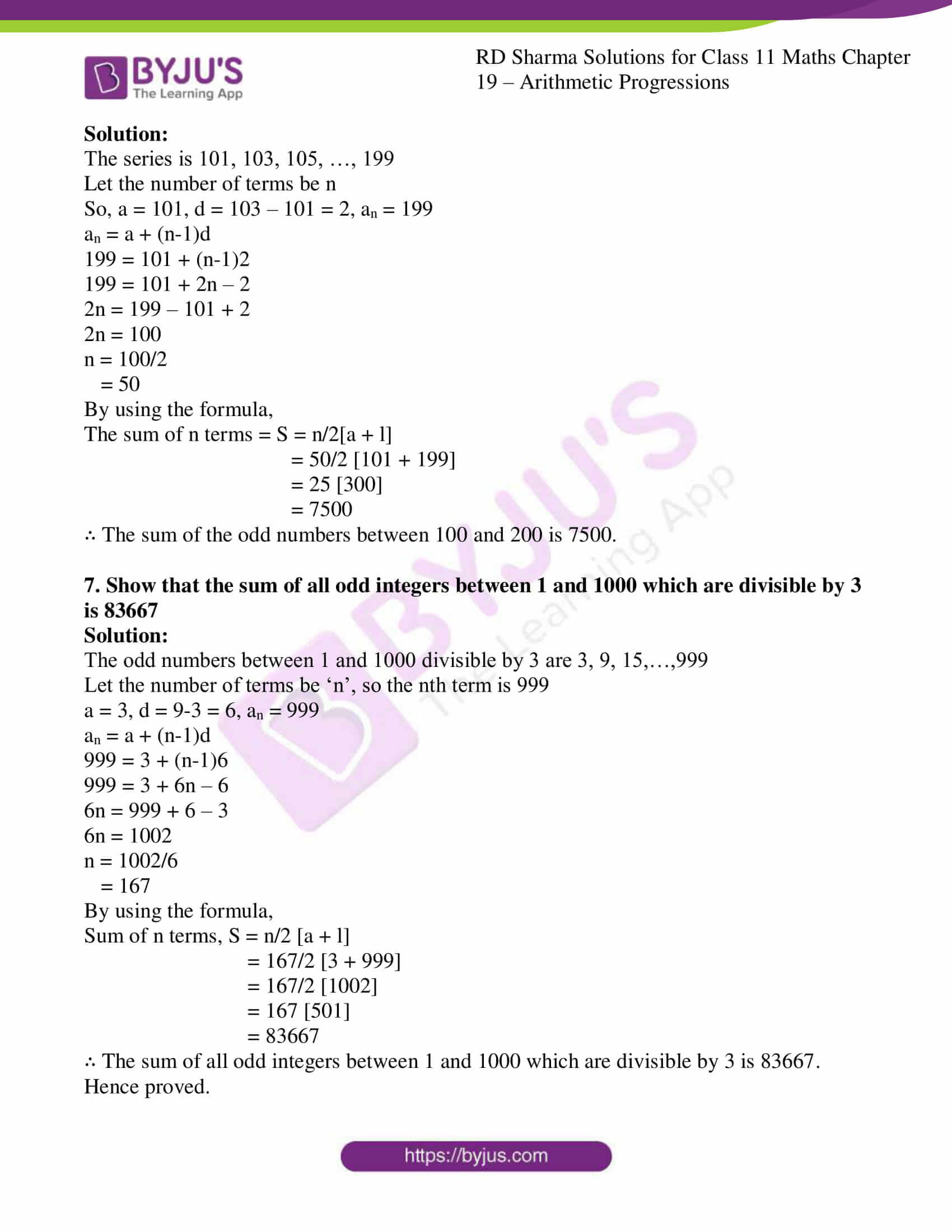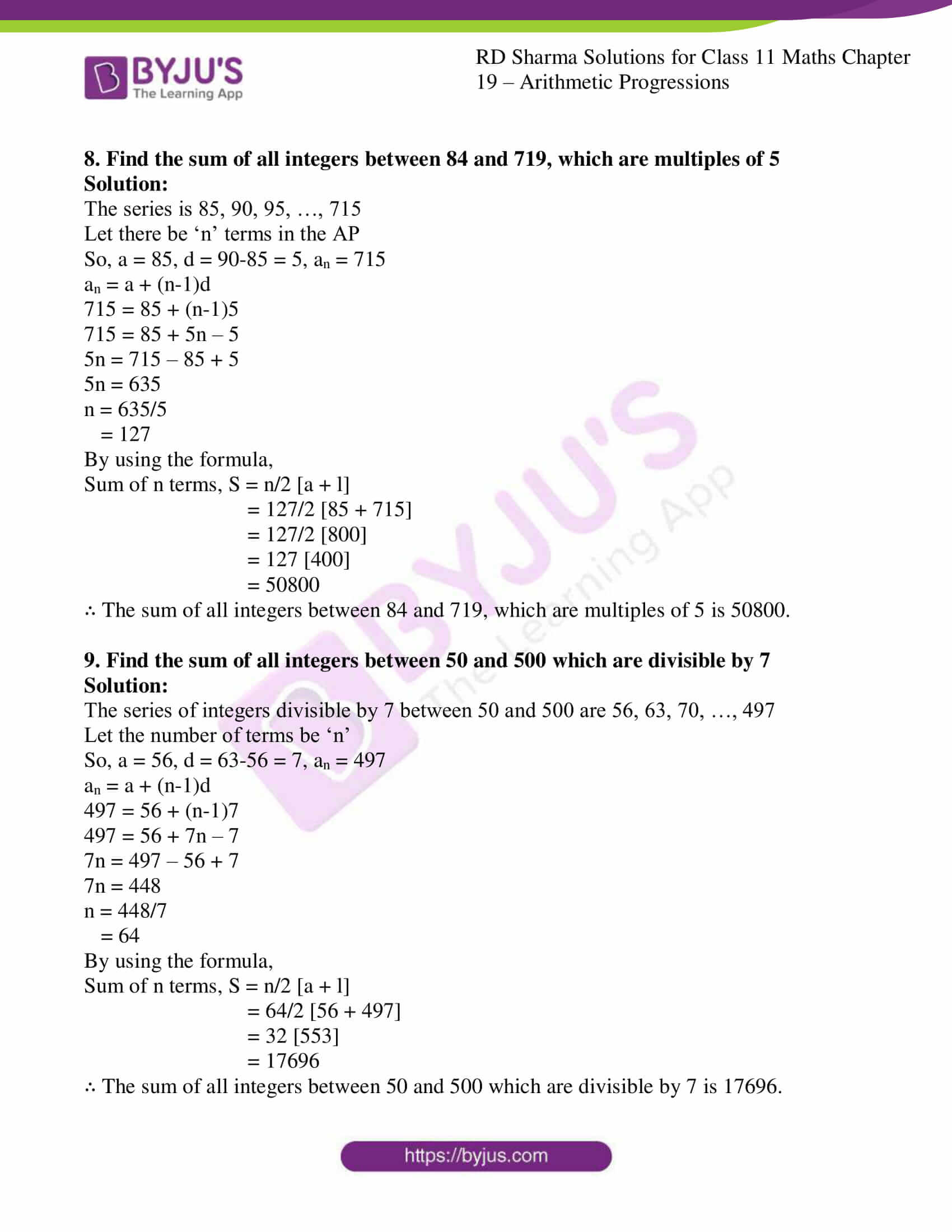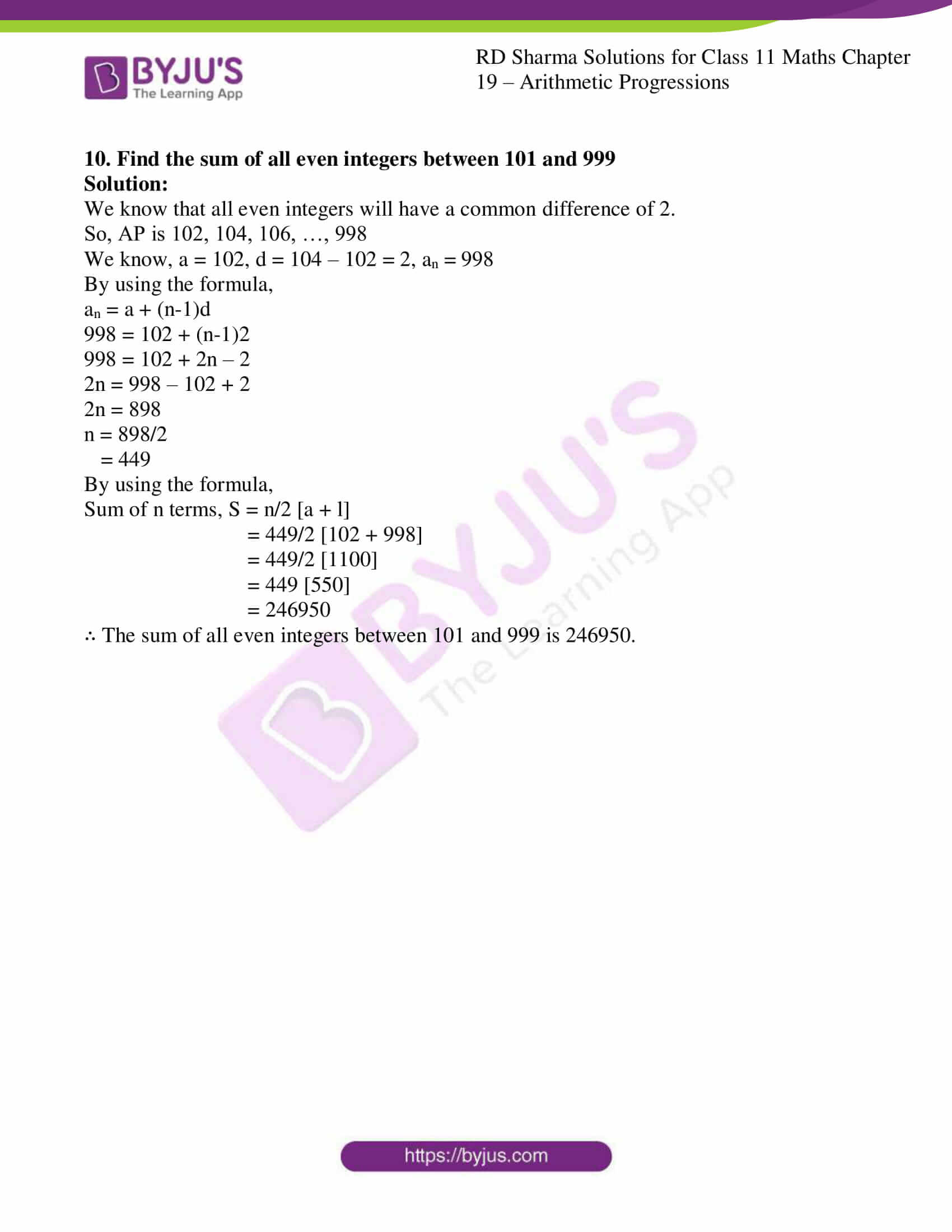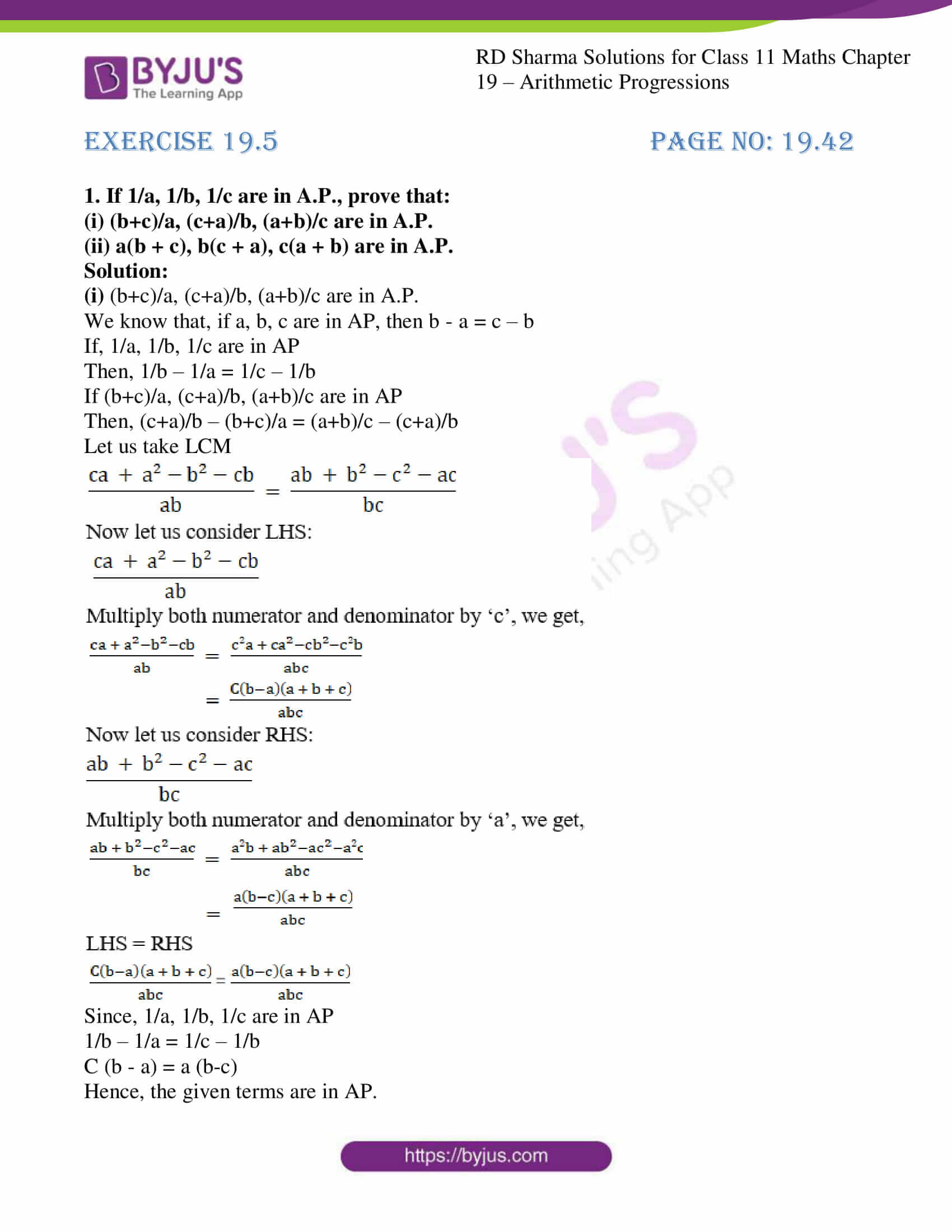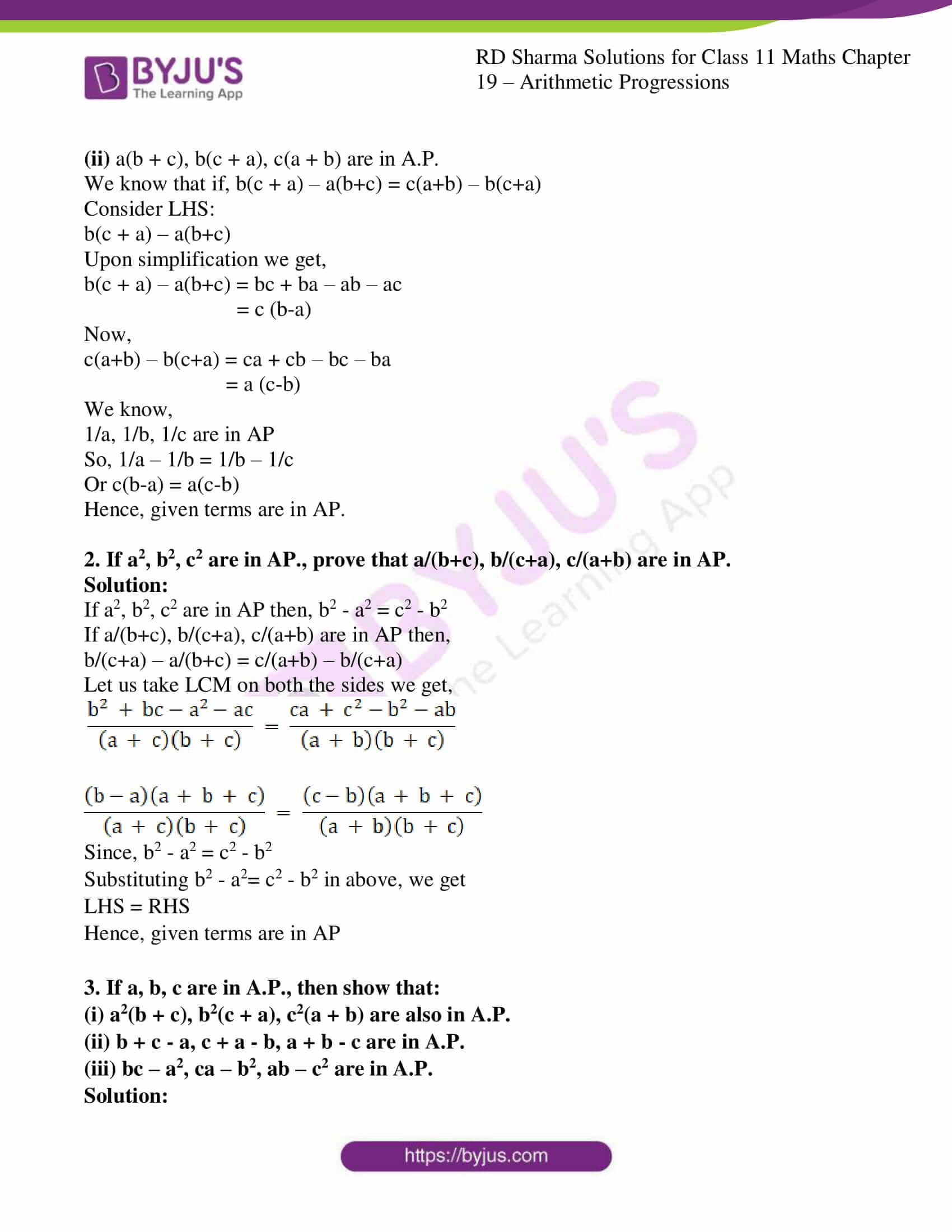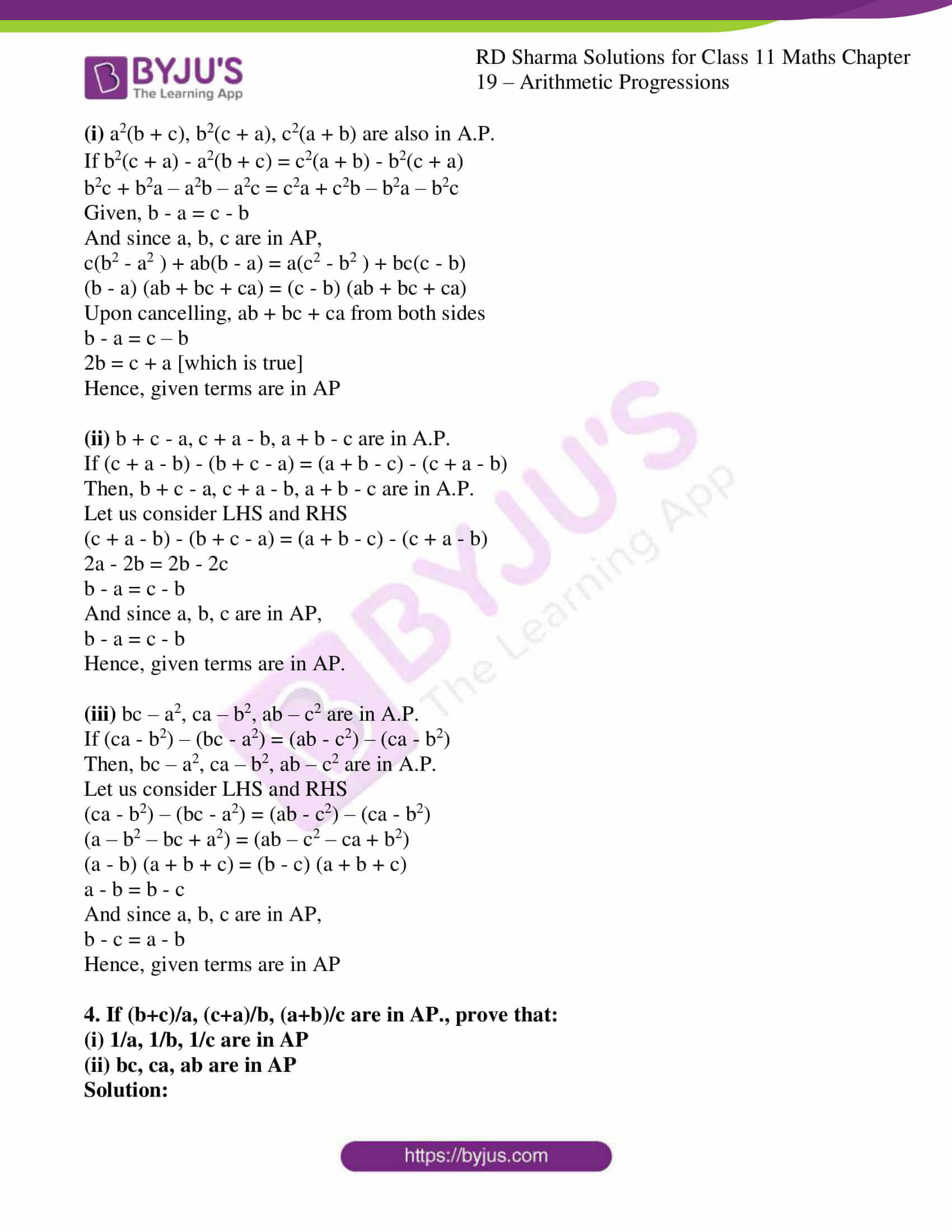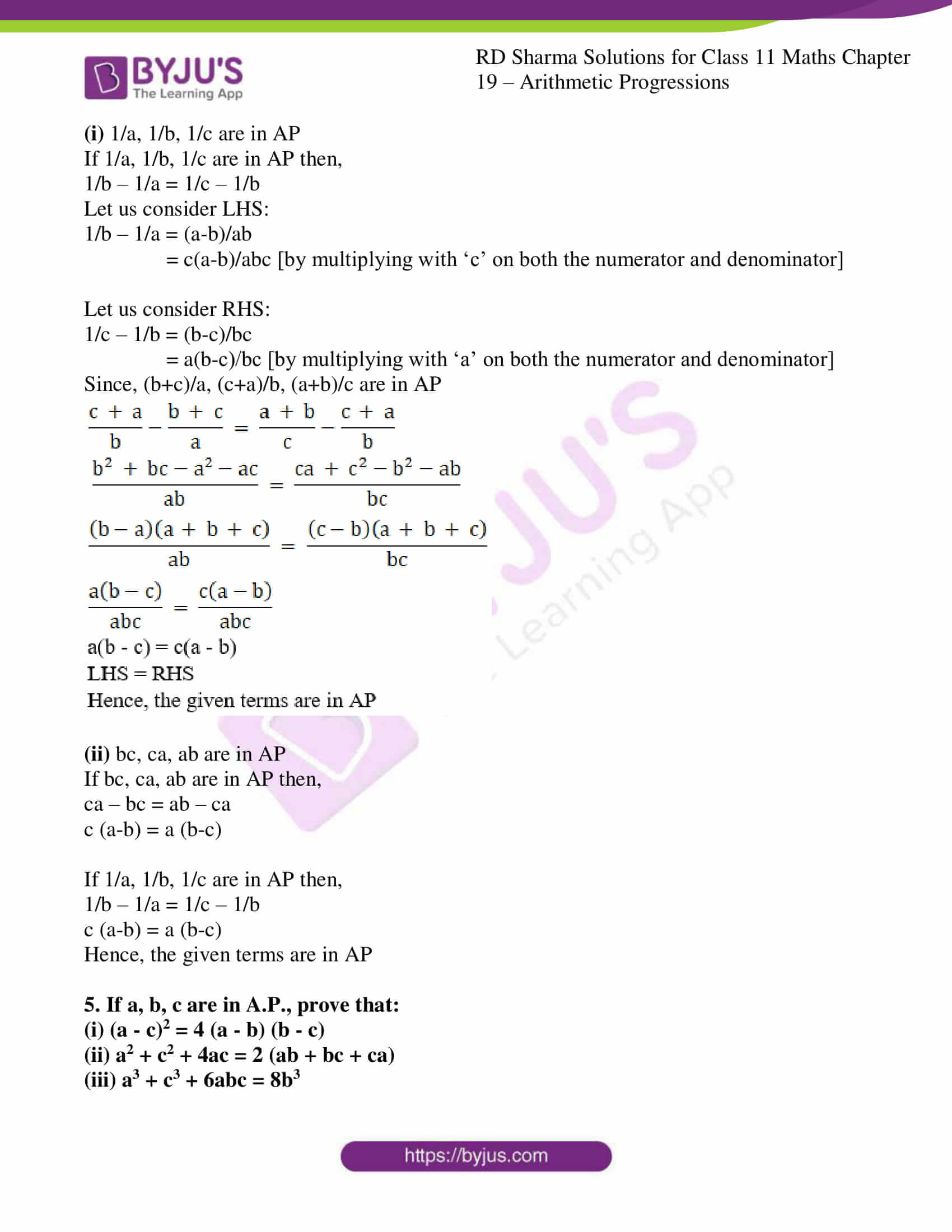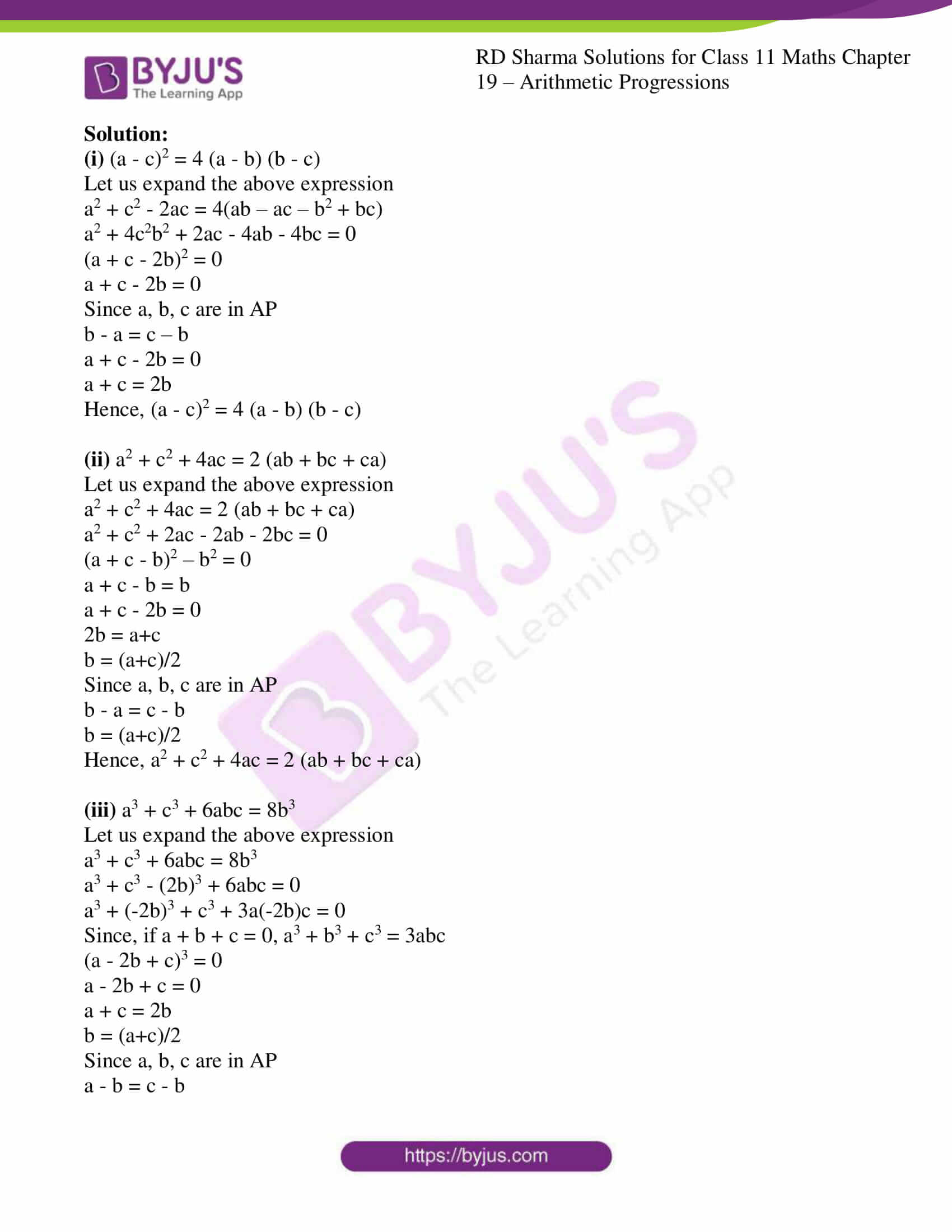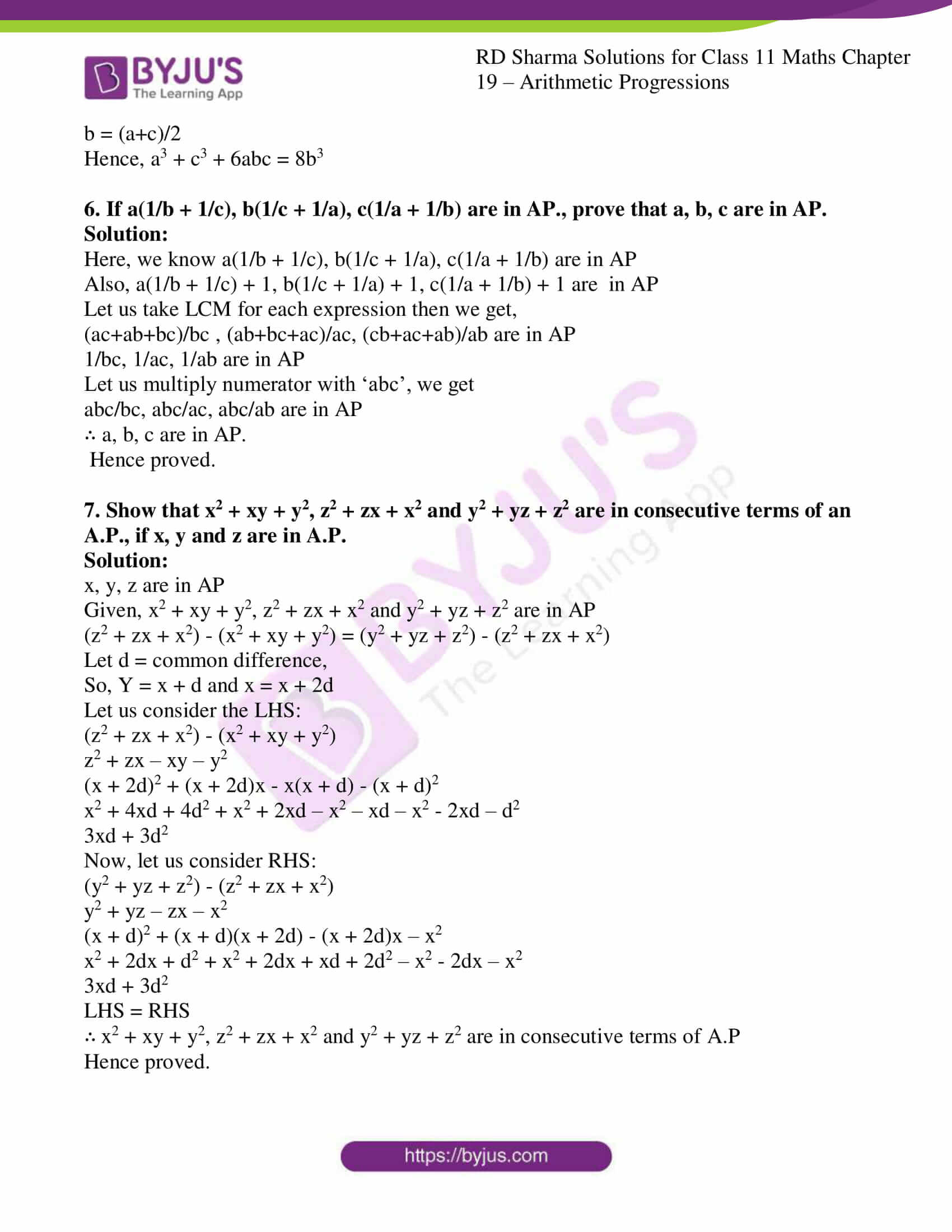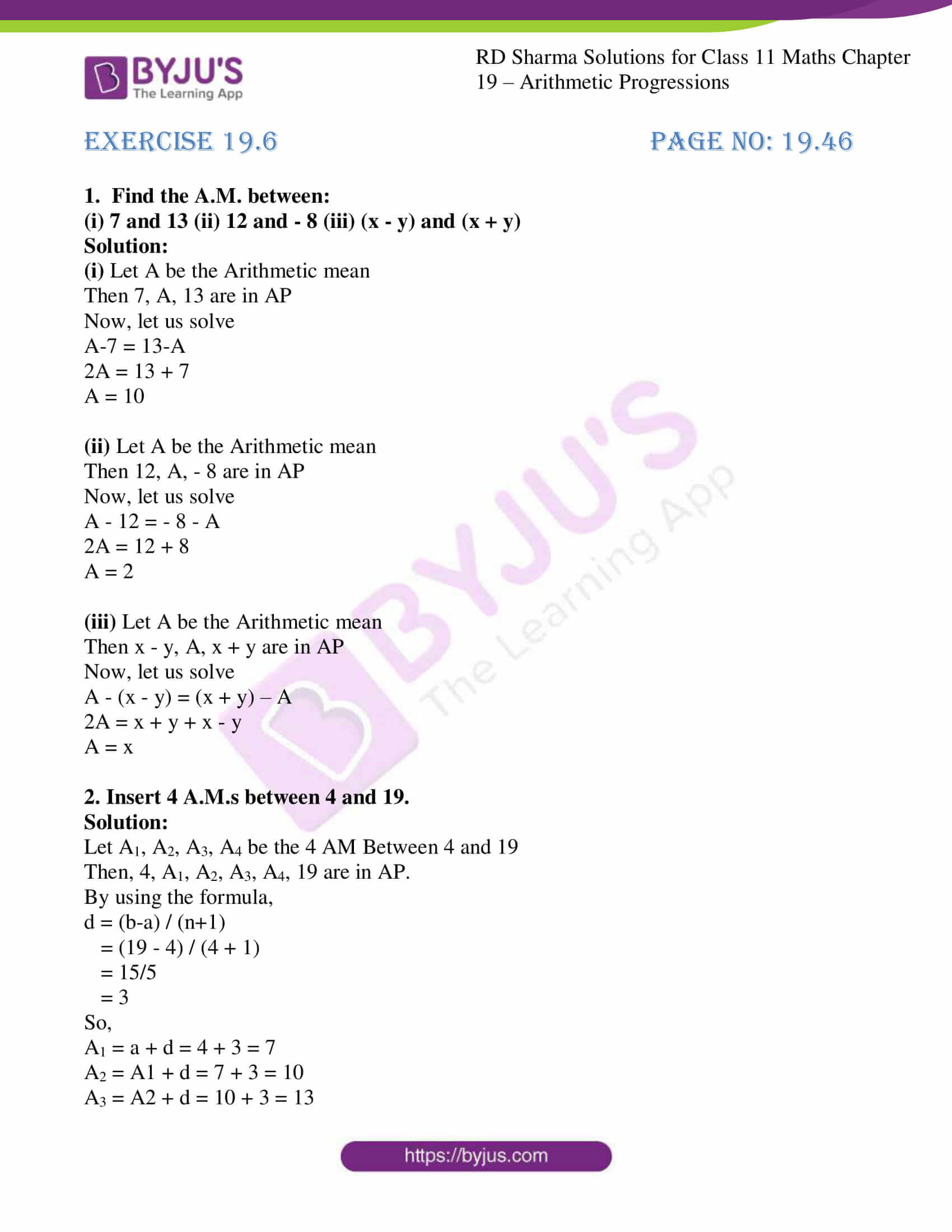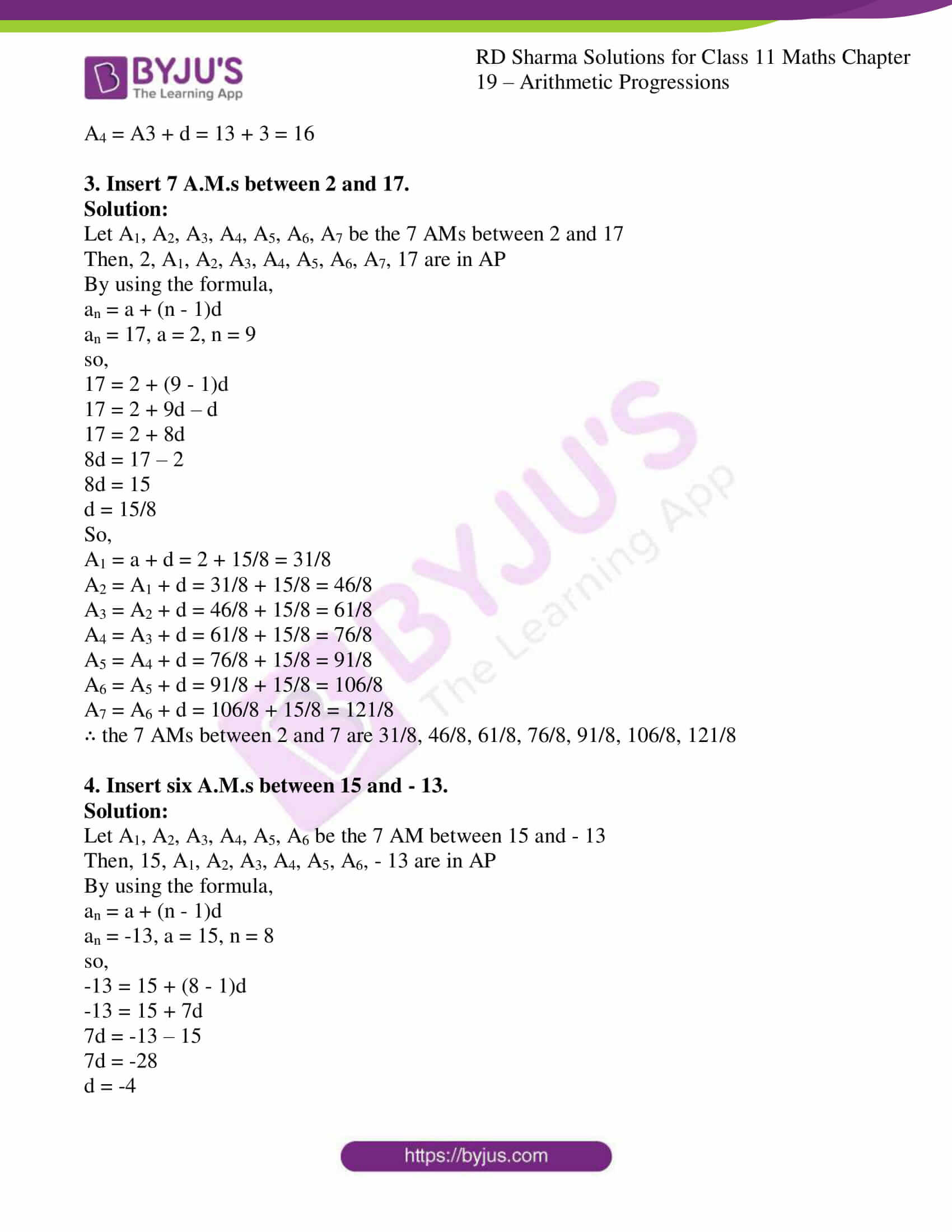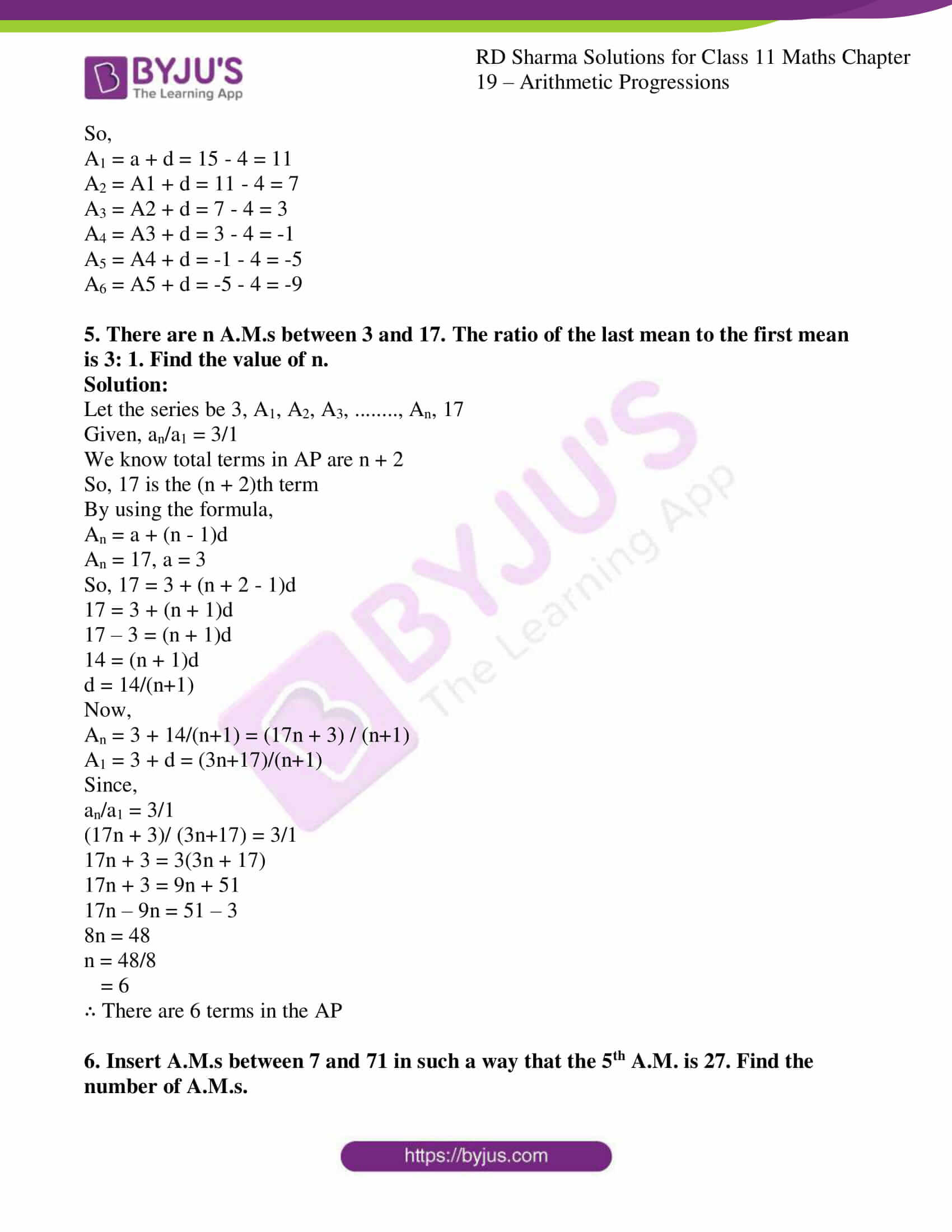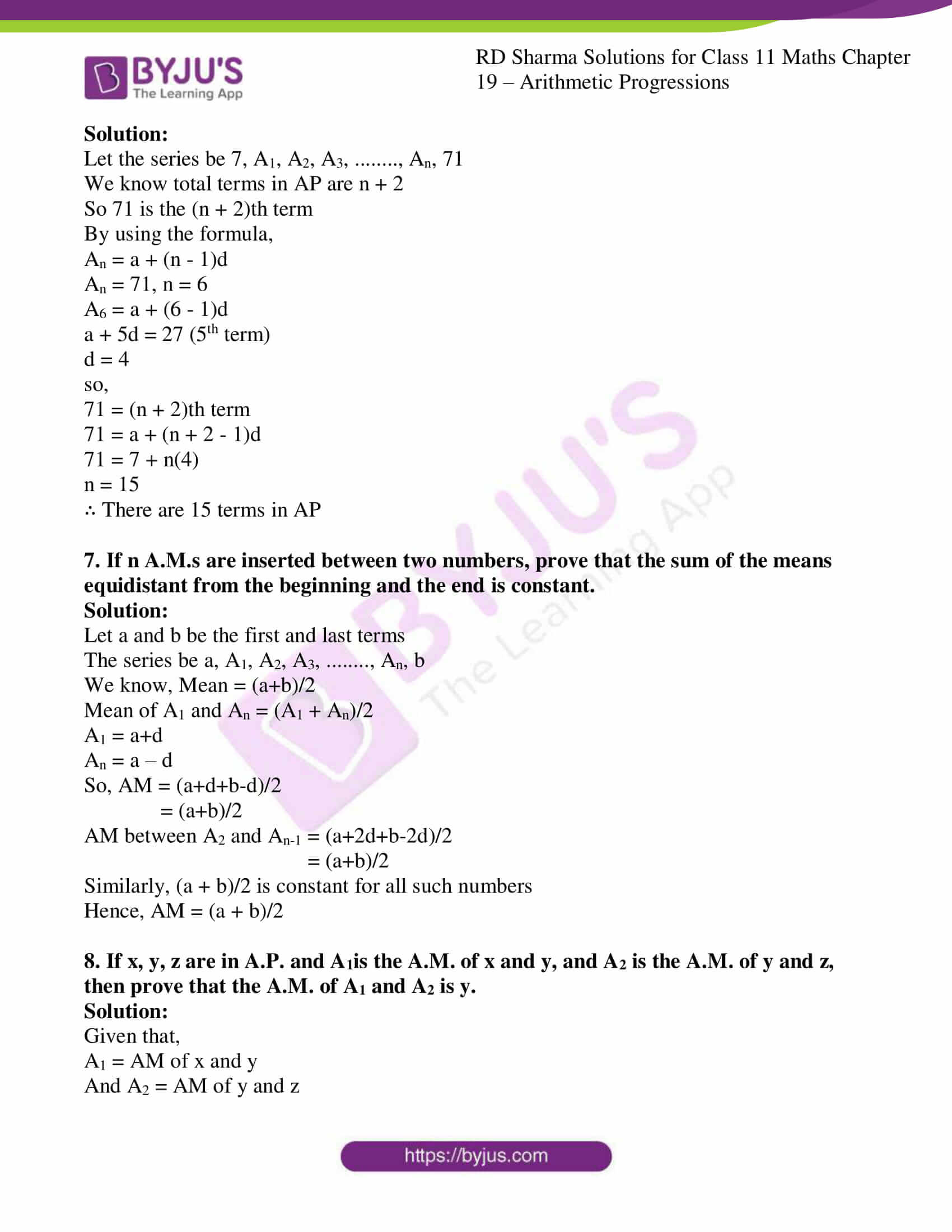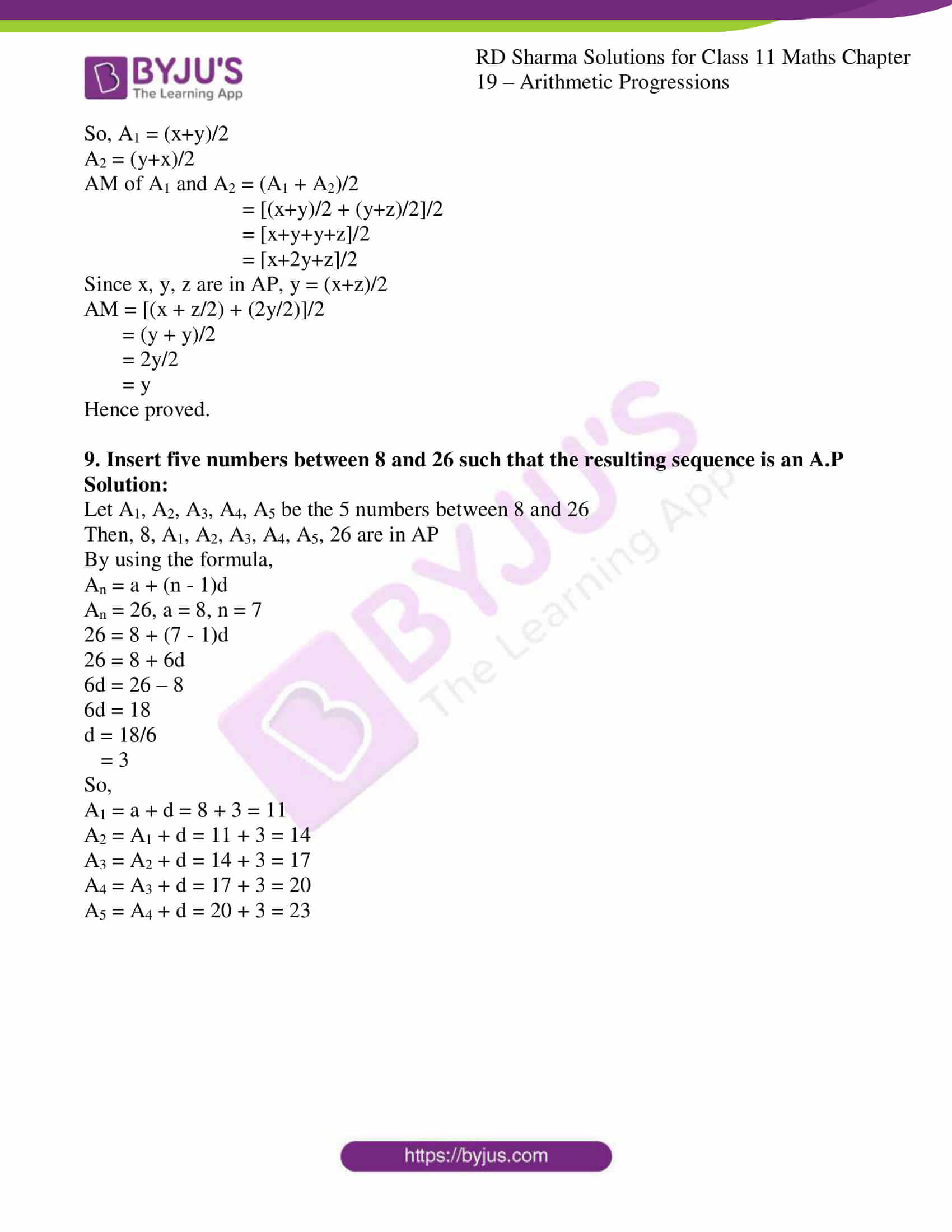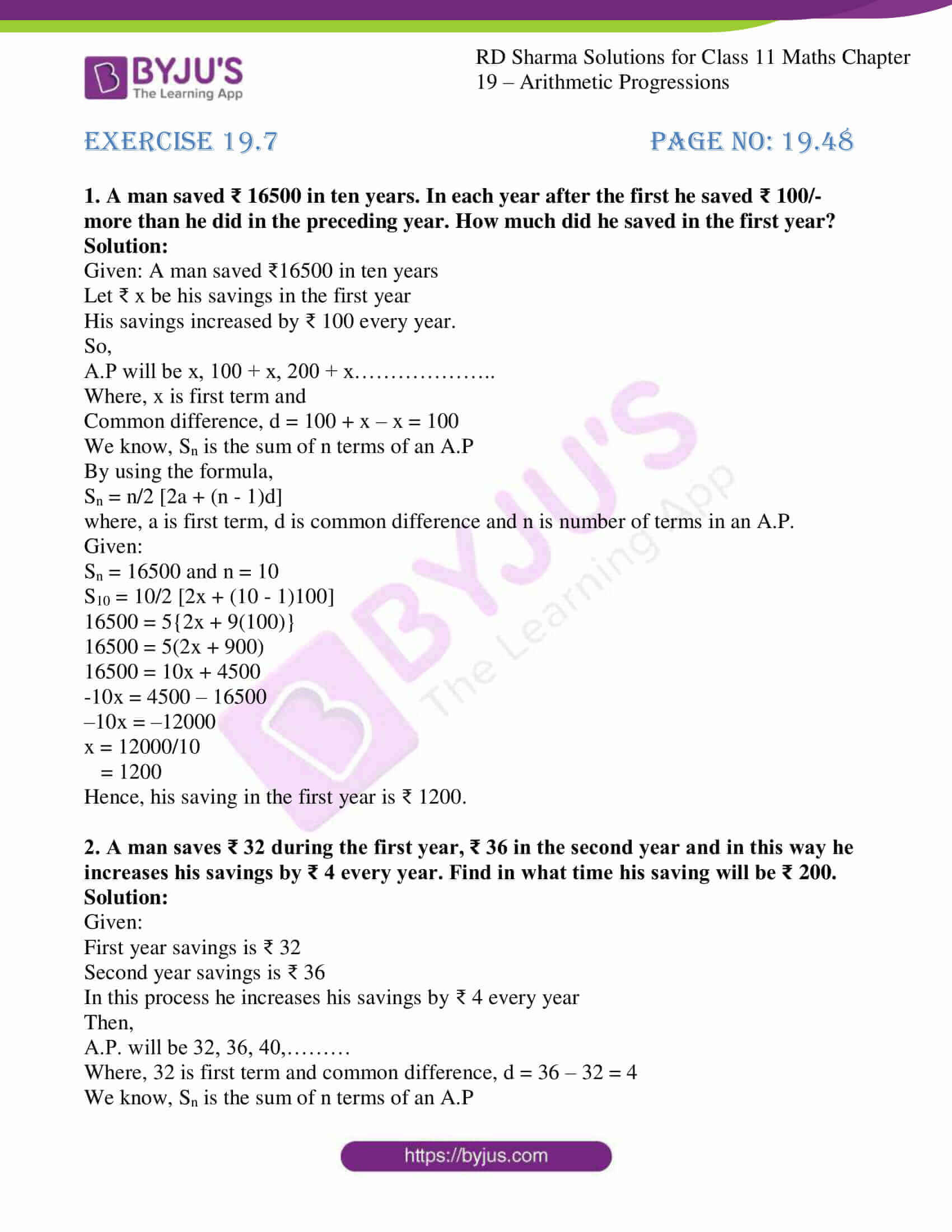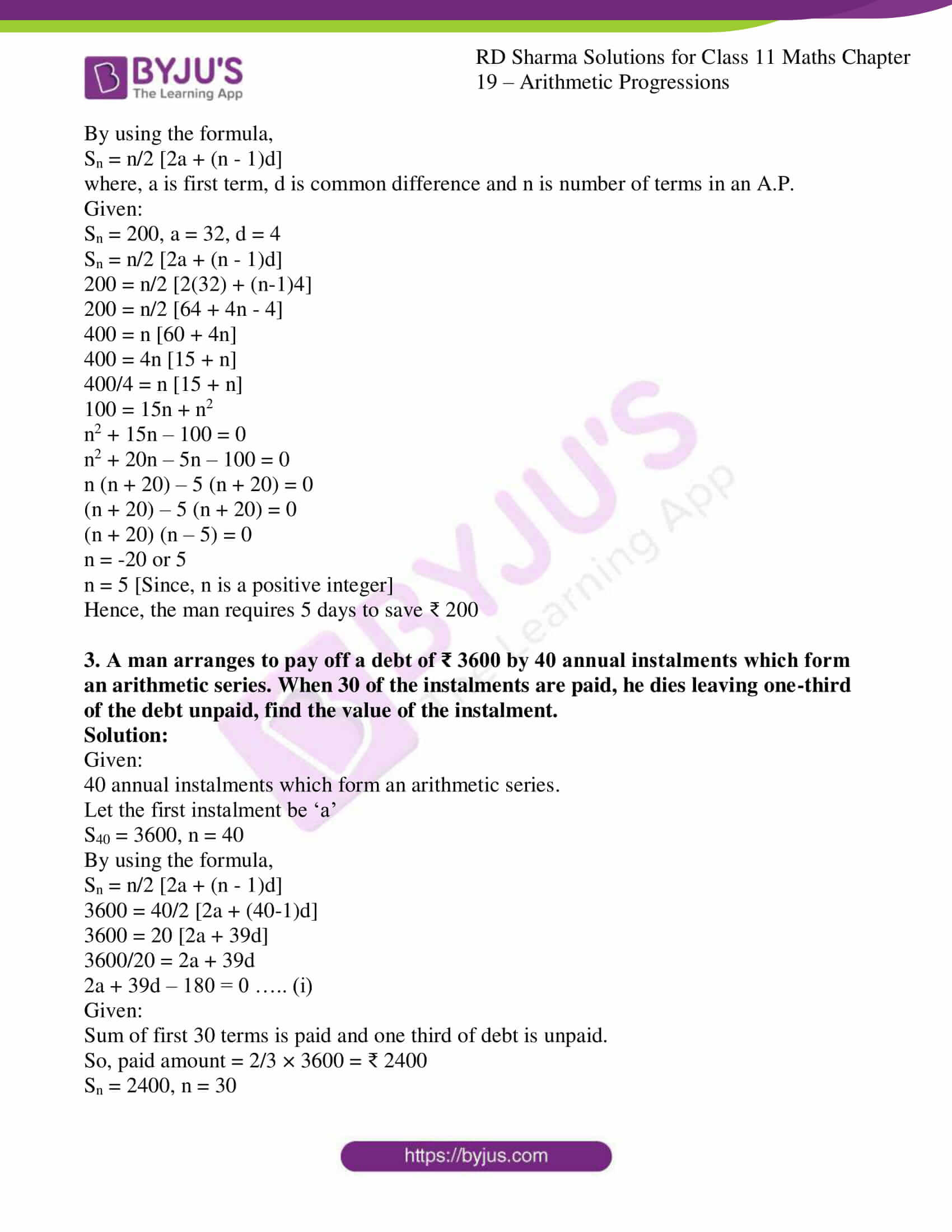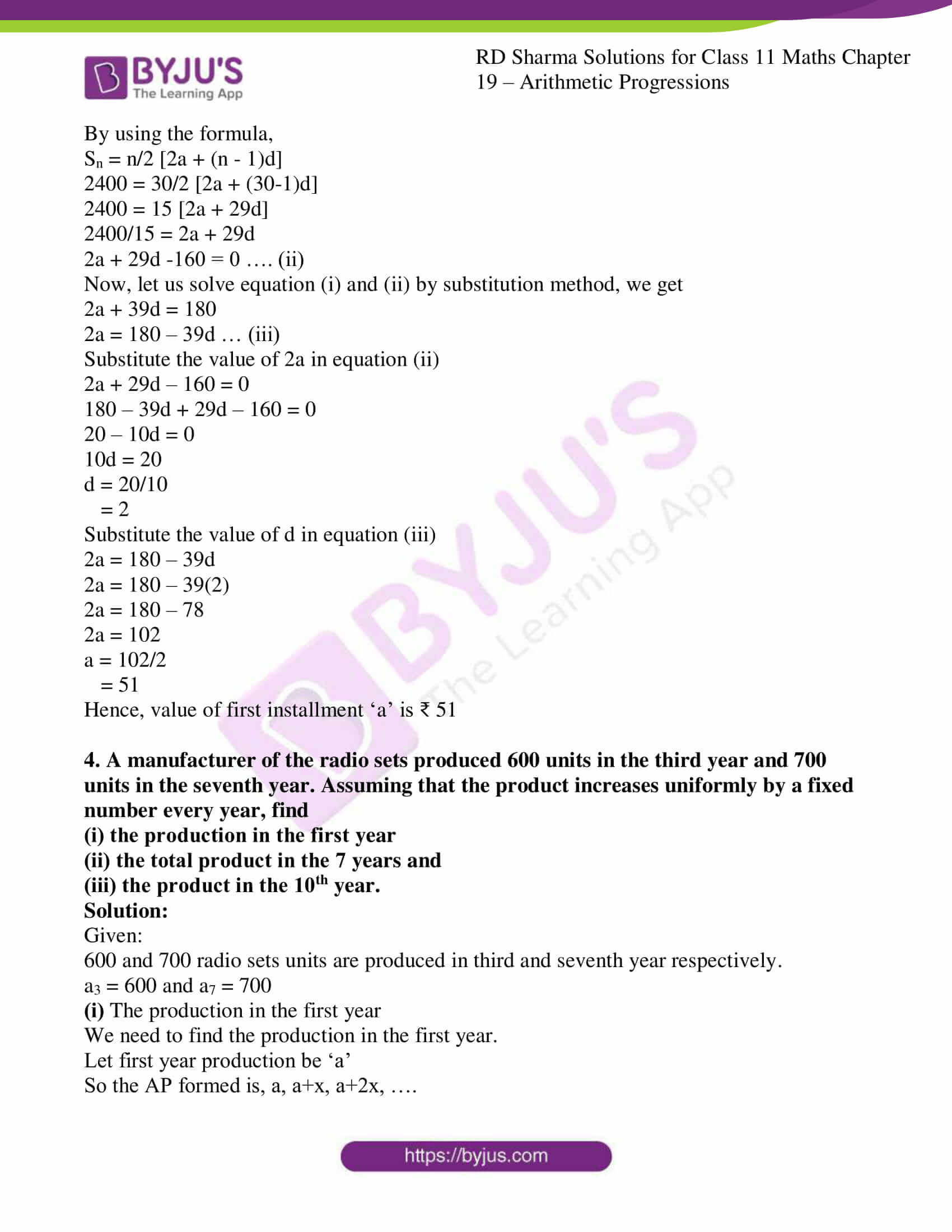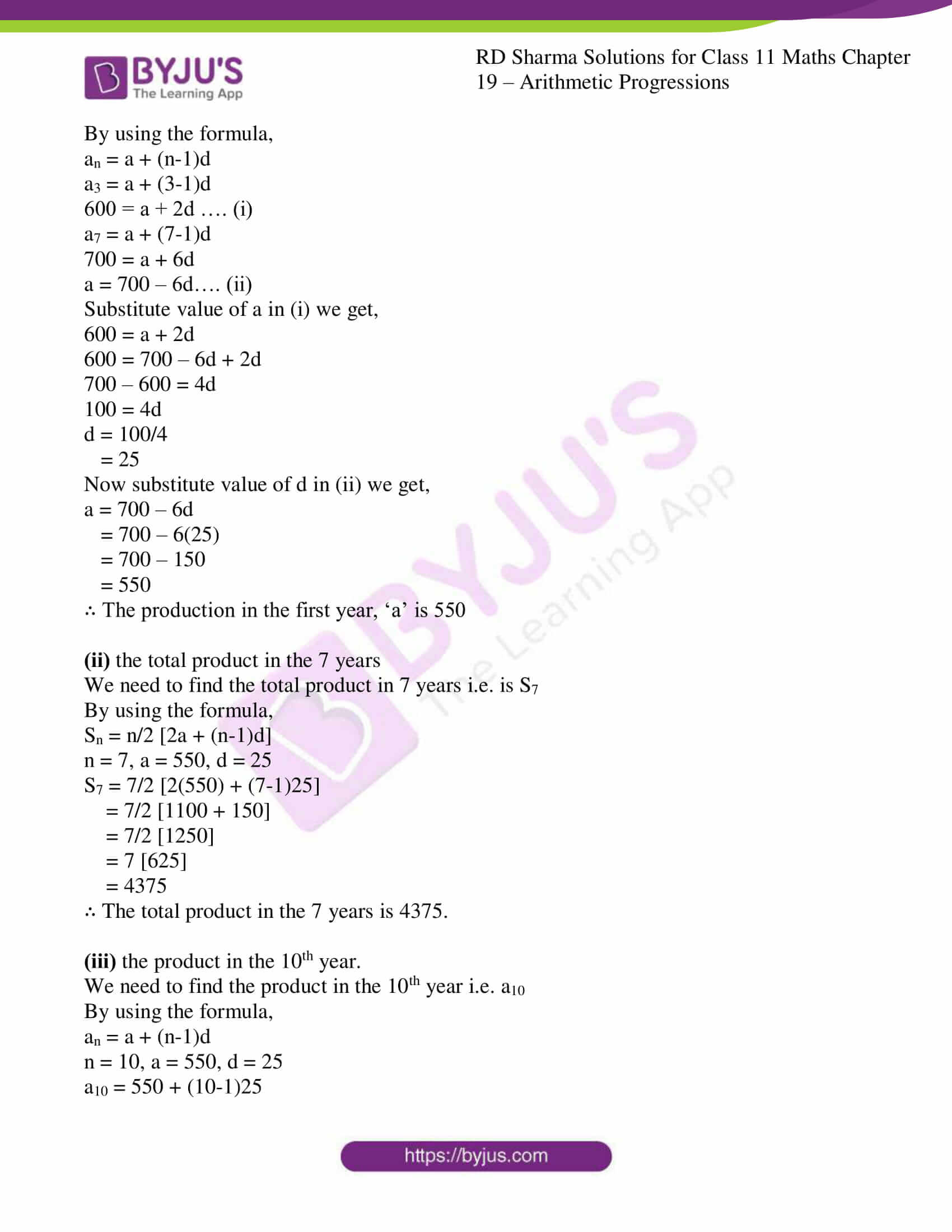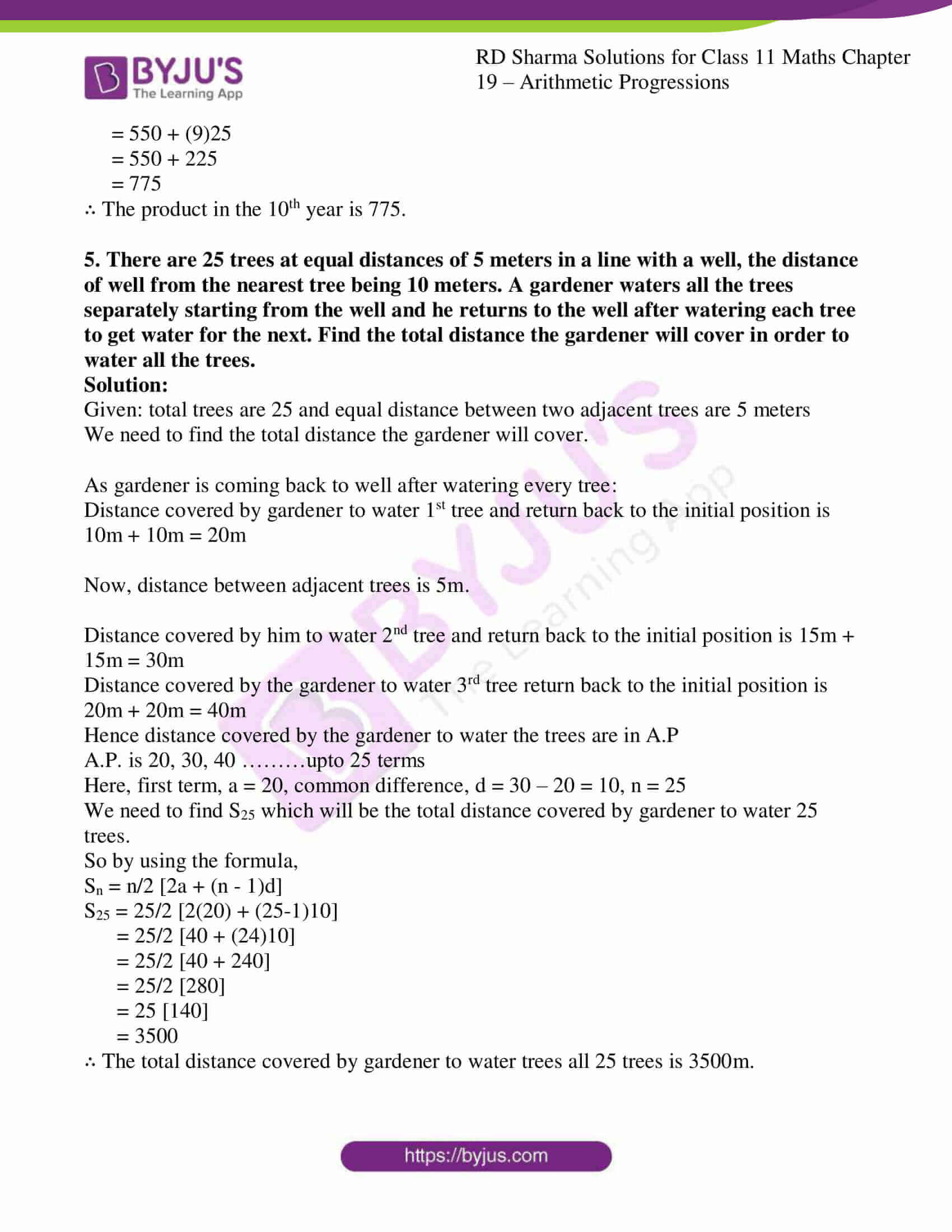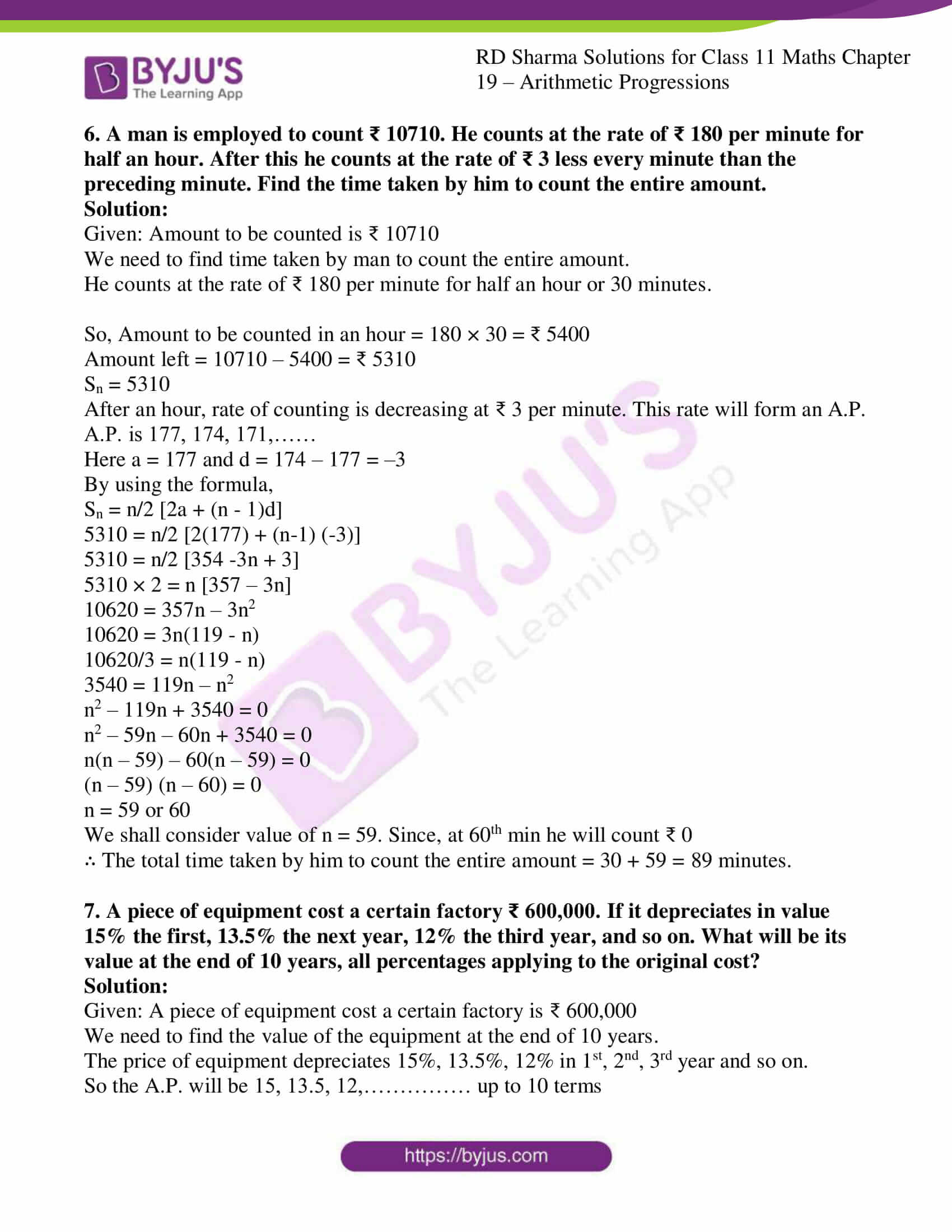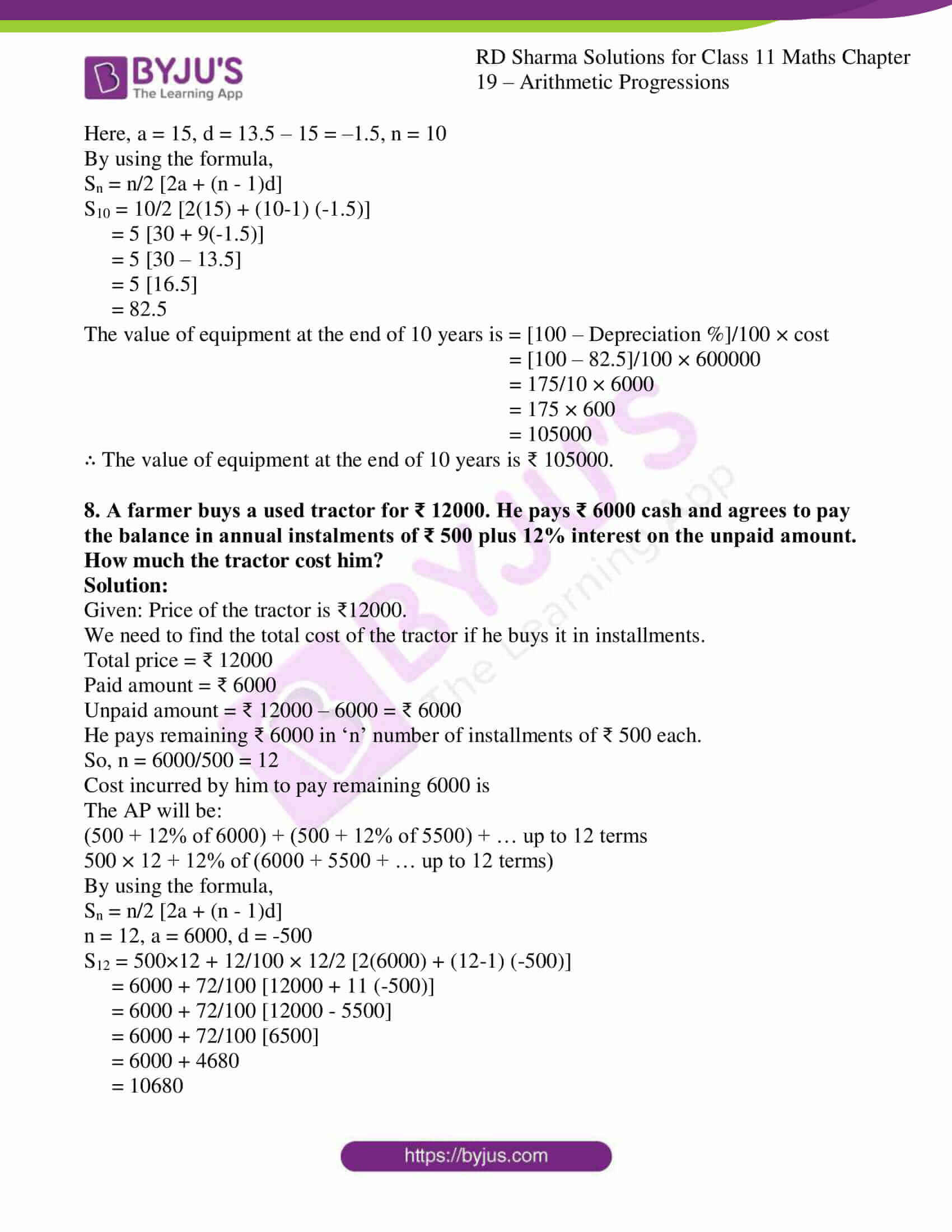### Access answers to RD Sharma Solutions for Class 11 Maths Chapter 19 – Arithmetic Progressions

EXERCISE 19.1 PAGE NO: 19.4

1. If the nth term of a sequence is given by an = n2 – n+1, write down its first five terms.

Solution:

Given:

an = n2 – n+1

By using the values n = 1, 2, 3, 4, 5 we can find the first five terms.

When n = 1:

a1 = (1)2 – 1 + 1

= 1 – 1 + 1

= 1

When n = 2:

a2 = (2)2 – 2 + 1

= 4 – 2 + 1

= 3

When n = 3:

a3 = (3)2 – 3 + 1

= 9 – 3 + 1

= 7

When n = 4:

a4 = (4)2 – 4 + 1

= 16 – 4 + 1

= 13

When n = 5:

a5 = (5)2 – 5 + 1

= 25 – 5 + 1

= 21

∴ First five terms of the sequence are 1, 3, 7, 13, 21.

2. A sequence is defined by an = n3 – 6n2 + 11n – 6, n ∈ N. Show that the first three terms of the sequence are zero and all other terms are positive.

Solution:

Given:

an = n3 – 6n2 + 11n – 6, n ∈ N

By using the values n = 1, 2, 3 we can find the first three terms.

When n = 1:

a1 = (1)3 – 6(1)2 + 11(1) – 6

= 1 – 6 + 11 – 6

= 12 – 12

= 0

When n = 2:

a2 = (2)3 – 6(2)2 + 11(2) – 6

= 8 – 6(4) + 22 – 6

= 8 – 24 + 22 – 6

= 30 – 30

= 0

When n = 3:

a3 = (3)3 – 6(3)2 + 11(3) – 6

= 27 – 6(9) + 33 – 6

= 27 – 54 + 33 – 6

= 60 – 60

= 0

This shows that the first three terms of the sequence is zero.

Now, let’s check for when n = n:

an = n3 – 6n2 + 11n – 6

= n3 – 6n2 + 11n – 6 – n + n – 2 + 2

= n3 – 6n2 + 12n – 8 – n + 2

= (n)3 – 3×2n(n – 2) – (2)3 – n + 2

By using the formula, {(a – b)3 = (a)3 – (b)3 – 3ab(a – b)}

an = (n – 2)3 – (n – 2)

Here, n – 2 will always be positive for n > 3

∴ an is always positive for n > 3

3. Find the first four terms of the sequence defined by a1 = 3 and an = 3an–1 + 2, for all n > 1.

Solution:

Given:

a1 = 3 and an = 3an–1 + 2, for all n > 1

By using the values n = 1, 2, 3, 4 we can find the first four terms.

When n = 1:

a1 = 3

When n = 2:

a2 = 3a2–1 + 2

= 3a1 + 2

= 3(3) + 2

= 9 + 2

= 11

When n = 3:

a3 = 3a3–1 + 2

= 3a2 + 2

= 3(11) + 2

= 33 + 2

= 35

When n = 4:

a4 = 3a4–1 + 2

= 3a3 + 2

= 3(35) + 2

= 105 + 2

= 107

∴ First four terms of sequence are 3, 11, 35, 107.

4. Write the first five terms in each of the following sequences:
(i) a1 = 1, an = an–1 + 2, n > 1

(ii) a1 = 1 = a2, an = an–1 + an–2, n > 2

(iii) a1 = a2 =2, an = an–1 – 1, n > 2

Solution:

(i) a1 = 1, an = an–1 + 2, n > 1

By using the values n = 1, 2, 3, 4, 5 we can find the first five terms.

Given:

a1 = 1

When n = 2:

a2 = a2–1 + 2

= a1 + 2

= 1 + 2

= 3

When n = 3:

a3 = a3–1 + 2

= a2 + 2

= 3 + 2

= 5

When n = 4:

a4 = a4–1 + 2

= a3 + 2

= 5 + 2

= 7

When n = 5:

a5 = a5–1 + 2

= a4 + 2

= 7 + 2

= 9

∴ First five terms of the sequence are 1, 3, 5, 7, 9.

(ii) a1 = 1 = a2, an = an–1 + an–2, n > 2

By using the values n = 1, 2, 3, 4, 5 we can find the first five terms.

Given:

a1 = 1

a2 = 1

When n = 3:

a3 = a3–1 + a3–2

= a2 + a1

= 1 + 1

= 2

When n = 4:

a4 = a4–1 + a4–2

= a3 + a2

= 2 + 1

= 3

When n = 5:

a5 = a5–1 + a5–2

= a4 + a3

= 3 + 2

= 5

∴ First five terms of the sequence are 1, 1, 2, 3, 5.

(iii) a1 = a2 =2, an = an–1 – 1, n > 2

By using the values n = 1, 2, 3, 4, 5 we can find the first five terms.

Given:

a1 = 2

a2 = 2

When n = 3:

a3 = a3–1 – 1

= a2 – 1

= 2 – 1

= 1

When n = 4:

a4 = a4–1 – 1

= a3 – 1

= 1 – 1

= 0

When n = 5:

a5 = a5–1 – 1

= a4 – 1

= 0 – 1

= -1

∴ First five terms of the sequence are 2, 2, 1, 0, -1.

5. The Fibonacci sequence is defined by a1 = 1 = a2, an = an–1 + an–2 for n > 2. Find (an+1)/an for n = 1, 2, 3, 4, 5.

Solution:

Given:

a1 = 1

a2 = 1

an = an–1 + an–2

When n = 1:

(an+1)/an = (a1+1)/a1

= a2/a1

= 1/1

= 1

a3 = a3–1 + a3–2

= a2 + a1

= 1 + 1

= 2

When n = 2:

(an+1)/an = (a2+1)/a2

= a3/a2

= 2/1

= 2

a4 = a4–1 + a4–2

= a3 + a2

= 2 + 1

= 3

When n = 3:

(an+1)/an = (a3+1)/a3

= a4/a3

= 3/2

a5 = a5–1 + a5–2

= a4 + a3

= 3 + 2

= 5

When n = 4:

(an+1)/an = (a4+1)/a4

= a5/a4

= 5/3

a6 = a6–1 + a6–2

= a5 + a4

= 5 + 3

= 8

When n = 5:

(an+1)/an = (a5+1)/a5

= a6/a5 = 8/5

∴ Value of (an+1)/an when n = 1, 2, 3, 4, 5 are 1, 2, 3/2, 5/3, 8/5

EXERCISE 19.2 PAGE NO: 19.11

1. Find:
(i) 10th term of the A.P. 1, 4, 7, 10, …..

(ii) 18th term of the A.P. √2, 3√2, 5√2, …

(iii) nth term of the A.P 13, 8, 3, -2, ….

Solution:

(i) 10th term of the A.P. 1, 4, 7, 10, …..

Arithmetic Progression (AP) whose common difference is = an – an-1 where n > 0

Let us consider, a = a1 = 1, a2 = 4 …

So, Common difference, d = a2 – a1 = 4 – 1 = 3

To find the 10th term of A.P, firstly find an

By using the formula,

an = a + (n-1) d

= 1 + (n-1) 3

= 1 + 3n – 3

= 3n – 2

When n = 10:

a10 = 3(10) – 2

= 30 – 2

= 28

Hence, 10th term is 28.

(ii) 18th term of the A.P. √2, 3√2, 5√2, …

Arithmetic Progression (AP) whose common difference is = an – an-1 where n > 0

Let us consider, a = a1 = √2, a2 = 3√2 …

So, Common difference, d = a2 – a1 = 3√2 – √2 = 2√2

To find the 18th term of A.P, firstly find an

By using the formula,

an = a + (n-1) d

= √2 + (n – 1) 2√2

= √2 + 2√2n – 2√2

= 2√2n – √2

When n = 18:

a18 = 2√2(18) – √2

= 36√2 – √2

= 35√2

Hence, 10th term is 35√2

(iii) nth term of the A.P 13, 8, 3, -2, ….

Arithmetic Progression (AP) whose common difference is = an – an-1 where n > 0

Let us consider, a = a1 = 13, a2 = 8 …

So, Common difference, d = a2 – a1 = 8 – 13 = -5

To find the nth term of A.P, firstly find an

By using the formula,

an = a + (n-1) d

= 13 + (n-1) (-5)

= 13 – 5n + 5

= 18 – 5n

Hence, nth term is 18 – 5n

2. In an A.P., show that am+n + am–n = 2am.

Solution:

We know the first term is ‘a’ and the common difference of an A.P is d.

Given:

am+n + am–n = 2am

By using the formula,

an = a + (n – 1)d

Now, let us take LHS: am+n + am-n

am+n + am-n = a + (m + n – 1)d + a + (m – n – 1)d

= a + md + nd – d + a + md – nd – d

= 2a + 2md – 2d

= 2(a + md – d)

= 2[a + d(m – 1)] {∵ an = a + (n – 1)d}

am+n + am-n = 2am

Hence Proved.

3. (i) Which term of the A.P. 3, 8, 13,… is 248 ?

(ii) Which term of the A.P. 84, 80, 76,… is 0 ?

(iii) Which term of the A.P. 4, 9, 14,… is 254 ?

Solution:

(i) Which term of the A.P. 3, 8, 13,… is 248 ?

Given A.P is 3, 8, 13,…

Here, a1 = a = 3, a2 = 8

Common difference, d = a2 – a1 = 8 – 3 = 5

We know, an = a + (n – 1)d

an = 3 + (n – 1)5

= 3 + 5n – 5

= 5n – 2

Now, to find which term of A.P is 248

Put an = 248

∴ 5n – 2 = 248

= 248 + 2

= 250

= 250/5

= 50

Hence, 50th term of given A.P is 248.

(ii) Which term of the A.P. 84, 80, 76,… is 0 ?

Given A.P is 84, 80, 76,…

Here, a1 = a = 84, a2 = 88

Common difference, d = a2 – a1 = 80 – 84 = -4

We know, an = a + (n – 1)d

an = 84 + (n – 1)-4

= 84 – 4n + 4

= 88 – 4n

Now, to find which term of A.P is 0

Put an = 0

88 – 4n = 0

-4n = -88

n = 88/4

= 22

Hence, 22nd term of given A.P is 0.

(iii) Which term of the A.P. 4, 9, 14,… is 254 ?

Given A.P is 4, 9, 14,…

Here, a1 = a = 4, a2 = 9

Common difference, d = a2 – a1 = 9 – 4 = 5

We know, an = a + (n – 1)d

an = 4 + (n – 1)5

= 4 + 5n – 5

= 5n – 1

Now, to find which term of A.P is 254

Put an = 254

5n – 1 = 254

5n = 254 + 1

5n = 255

n = 255/5

= 51

Hence, 51st term of given A.P is 254.

4. (i) Is 68 a term of the A.P. 7, 10, 13,…?

(ii) Is 302 a term of the A.P. 3, 8, 13,…?

Solution:

(i) Is 68 a term of the A.P. 7, 10, 13,…?

Given A.P is 7, 10, 13,…

Here, a1 = a = 7, a2 = 10

Common difference, d = a2 – a1 = 10 – 7 = 3

We know, an = a + (n – 1)d [where, a is first term or a1 and d is common difference and n is any natural number]

an = 7 + (n – 1)3

= 7 + 3n – 3

= 3n + 4

Now, to find whether 68 is a term of this A.P. or not

Put an = 68

3n + 4 = 68

3n = 68 – 4

3n = 64

n = 64/3

64/3 is not a natural number

Hence, 68 is not a term of given A.P.

(ii) Is 302 a term of the A.P. 3, 8, 13,…?

Given A.P is 3, 8, 13,…

Here, a1 = a = 3, a2 = 8

Common difference, d = a2 – a1 = 8 – 3 = 5

We know, an = a + (n – 1)d

an = 3 + (n – 1)5

= 3 + 5n – 5

= 5n – 2

To find whether 302 is a term of this A.P. or not

Put an = 302

5n – 2 = 302

5n = 302 + 2

5n = 304

n = 304/5

304/5 is not a natural number

Hence, 304 is not a term of given A.P.

5. (i) Which term of the sequence 24, 23 ¼, 22 ½, 21 ¾ is the first negative term?

Solution:

Given:

AP: 24, 23 ¼, 22 ½, 21 ¾, … = 24, 93/4, 45/2, 87/4, …

Here, a1 = a = 24, a2 = 93/4

Common difference, d = a2 – a1 = 93/4 – 24

= (93 – 96)/4

= – 3/4

We know, an = a + (n – 1) d [where a is first term or a1 and d is common difference and n is any natural number]

We know, an = a + (n – 1) d

an = 24 + (n – 1) (-3/4)

= 24 – 3/4n + ¾

= (96+3)/4 – 3/4n

= 99/4 – 3/4n

Now we need to find, first negative term.

Put an < 0

an = 99/4 – 3/4n < 0

99/4 < 3/4n

3n > 99

n > 99/3

n > 33

Hence, 34th term is the first negative term of given AP.

(ii) Which term of the sequence 12 + 8i, 11 + 6i, 10 + 4i, … is (a) purely real (b) purely imaginary ?

Solution:

Given:

AP: 12 + 8i, 11 + 6i, 10 + 4i, …

Here, a1 = a = 12 + 8i, a2 = 11 + 6i

Common difference, d = a2 – a1

= 11 + 6i – (12 + 8i)

= 11 – 12 + 6i – 8i

= -1 – 2i

We know, an = a + (n – 1) d [where a is first term or a1 and d is common difference and n is any natural number]

an = 12 + 8i + (n – 1) -1 – 2i

= 12 + 8i – n – 2ni + 1 + 2i

= 13 + 10i – n – 2ni

= (13 – n) + (10 – 2n) i

To find purely real term of this A.P., imaginary part have to be zero

10 – 2n = 0

2n = 10

n = 10/2

= 5

Hence, 5th term is purely real.

To find purely imaginary term of this A.P., real part have to be zero

∴ 13 – n = 0

n = 13

Hence, 13th term is purely imaginary.

6. (i) How many terms are in A.P. 7, 10, 13,…43?

Solution:

Given:

AP: 7, 10, 13,…

Here, a1 = a = 7, a2 = 10

Common difference, d = a2 – a1 = 10 – 7 = 3

We know, an = a + (n – 1) d [where a is first term or a1 and d is common difference and n is any natural number]

an = 7 + (n – 1)3

= 7 + 3n – 3

= 3n + 4

To find total terms of the A.P., put an = 43 as 43 is last term of A.P.

3n + 4 = 43

3n = 43 – 4

3n = 39

n = 39/3

= 13

Hence, total 13 terms exists in the given A.P.

(ii) How many terms are there in the A.P. -1, -5/6, -2/3, -1/2, …, 10/3 ?

Solution:

Given:

AP: -1, -5/6, -2/3, -1/2, …

Here, a1 = a = -1, a2 = -5/6

Common difference, d = a2 – a1

= -5/6 – (-1)

= -5/6 + 1

= (-5+6)/6

= 1/6

We know, an = a + (n – 1) d [where a is first term or a1 and d is common difference and n is any natural number]

an = -1 + (n – 1) 1/6

= -1 + 1/6n – 1/6

= (-6-1)/6 + 1/6n

= -7/6 + 1/6n

To find total terms of the AP,

Put an = 10/3 [Since, 10/3 is the last term of AP]

an = -7/6 + 1/6n = 10/3

1/6n = 10/3 + 7/6

1/6n = (20+7)/6

1/6n = 27/6

n = 27

Hence, total 27 terms exists in the given A.P.

7. The first term of an A.P. is 5, the common difference is 3, and the last term is 80; find the number of terms.

Solution:

Given:

First term, a = 5; last term, l = an = 80

Common difference, d = 3

We know, an = a + (n – 1) d [where a is first term or a1 and d is common difference and n is any natural number]

an = 5 + (n – 1)3

= 5 + 3n – 3

= 3n + 2

To find total terms of the A.P., put an = 80 as 80 is last term of A.P.

3n + 2 = 80

3n = 80 – 2

3n = 78

n = 78/3

= 26

Hence, total 26 terms exists in the given A.P.

8. The 6th and 17th terms of an A.P. are 19 and 41 respectively. Find the 40th term.

Solution:

Given:

6th term of an A.P is 19 and 17th terms of an A.P. is 41

So, a6 = 19 and a17 = 41

We know, an = a + (n – 1) d [where a is first term or a1 and d is common difference and n is any natural number]

When n = 6:

a6 = a + (6 – 1) d

= a + 5d

Similarly, When n = 17:

a17 = a + (17 – 1)d

= a + 16d

According to question:

a6 = 19 and a17 = 41

a + 5d = 19 ……………… (i)

And a + 16d = 41………….. (ii)

Let us subtract equation (i) from (ii) we get,

a + 16d – (a + 5d) = 41 – 19

a + 16d – a – 5d = 22

11d = 22

d = 22/11

= 2

put the value of d in equation (i):

a + 5(2) = 19

a + 10 = 19

a = 19 – 10

= 9

As, an = a + (n – 1)d

a40 = a + (40 – 1)d

= a + 39d

Now put the value of a = 9 and d = 2 in a40 we get,

a40 = 9 + 39(2)

= 9 + 78

= 87

Hence, 40th term of the given A.P. is 87.

9. If 9th term of an A.P. is Zero, prove that its 29th term is double the 19th term.

Solution:

Given:

9th term of an A.P is 0

So, a9 = 0

We need to prove: a29 = 2a19

We know, an = a + (n – 1) d [where a is first term or a1 and d is common difference and n is any natural number]

When n = 9:

a9 = a + (9 – 1)d

= a + 8d

According to question:

a9 = 0

a + 8d = 0

a = -8d

When n = 19:

a19 = a + (19 – 1)d

= a + 18d

= -8d + 18d

= 10d

When n = 29:

a29 = a + (29 – 1)d

= a + 28d

= -8d + 28d [Since, a = -8d]

= 20d

= 2×10d

a29 = 2a19 [Since, a19 = 10d]

Hence Proved.

10. If 10 times the 10th term of an A.P. is equal to 15 times the 15th term, show that the 25th term of the A.P. is Zero.

Solution:

Given:

10 times the 10th term of an A.P. is equal to 15 times the 15th term

So, 10a10 = 15a15

We need to prove: a25 = 0

We know, an = a + (n – 1) d [where a is first term or a1 and d is common difference and n is any natural number]

When n = 10:

a10 = a + (10 – 1)d

= a + 9d

When n = 15:

a15 = a + (15 – 1)d

= a + 14d

When n = 25:

a25 = a + (25 – 1)d

= a + 24d ………(i)

According to question:

10a10 = 15a15

10(a + 9d) = 15(a + 14d)

10a + 90d = 15a + 210d

10a – 15a + 90d – 210d = 0

-5a – 120d = 0

-5(a + 24d) = 0

a + 24d = 0

a25 = 0 [From (i)]

Hence Proved.

11. The 10th and 18th term of an A.P. are 41 and 73 respectively, find 26th term.

Solution:

Given:

10th term of an A.P is 41, and 18th terms of an A.P. is 73

So, a10 = 41 and a18 = 73

We know, an = a + (n – 1) d [where a is first term or a1 and d is the common difference and n is any natural number]

When n = 10:

a10 = a + (10 – 1)d

= a + 9d

When n = 18:

a18 = a + (18 – 1)d

= a + 17d

According to question:

a10 = 41 and a18 = 73

a + 9d = 41 ………………(i)

And a + 17d = 73…………..(ii)

Let us subtract equation (i) from (ii) we get,

a + 17d – (a + 9d) = 73 – 41

a + 17d – a – 9d = 32

8d = 32

d = 32/8

d = 4

Put the value of d in equation (i) we get,

a + 9(4) = 41

a + 36 = 41

a = 41 – 36

a = 5

we know, an = a + (n – 1)d

a26 = a + (26 – 1)d

= a + 25d

Now put the value of a = 5 and d = 4 in a26

a26 = 5 + 25(4)

= 5 + 100

= 105

Hence, 26th term of the given A.P. is 105.

12. In a certain A.P. the 24th term is twice the 10th term. Prove that the 72nd term is twice the 34th term.

Solution:

Given:

24th term is twice the 10th term

So, a24 = 2a10

We need to prove: a72 = 2a34

We know, an = a + (n – 1) d [where a is first term or a1 and d is common difference and n is any natural number]

When n = 10:

a10 = a + (10 – 1)d

= a + 9d

When n = 24:

a24 = a + (24 – 1)d

= a + 23d

When n = 34:

a34 = a + (34 – 1)d

= a + 33d ………(i)

When n = 72:

a72 = a + (72 – 1)d

= a + 71d

According to question:

a24 = 2a10

a + 23d = 2(a + 9d)

a + 23d = 2a + 18d

a – 2a + 23d – 18d = 0

-a + 5d = 0

a = 5d

Now, a72 = a + 71d

a72 = 5d + 71d

= 76d

= 10d + 66d

= 2(5d + 33d)

= 2(a + 33d) [since, a = 5d]

a72 = 2a34 (From (i))

Hence Proved.

EXERCISE 19.3 PAGE NO: 19.15

1. The Sum of the three terms of an A.P. is 21 and the product of the first, and the third terms exceed the second term by 6, find three terms.

Solution:

Given:

The sum of first three terms is 21

Let us assume the first three terms as a – d, a, a + d [where a is the first term and d is the common difference]

So, sum of first three terms is

a – d + a + a + d = 21

3a = 21

a = 7

It is also given that product of first and third term exceeds the second by 6

So, (a – d)(a + d) – a = 6

a2 – d2 – a = 6

Substituting the value of a = 7, we get

72 – d2 – 7 = 6

d2 = 36

d = 6 or d = – 6

Hence, the terms of AP are a – d, a, a + d which is 1, 7, 13.

2. Three numbers are in A.P. If the sum of these numbers be 27 and the product 648, find the numbers

Solution:

Given:

Sum of first three terms is 27

Let us assume the first three terms as a – d, a, a + d [where a is the first term and d is the common difference]

So, sum of first three terms is

a – d + a + a + d = 27

3a = 27

a = 9

It is given that the product of three terms is 648

So, a3 – ad2 = 648

Substituting the value of a = 9, we get

93 – 9d2 = 648

729 – 9d2 = 648

81 = 9d2

d = 3 or d = – 3

Hence, the given terms are a – d, a, a + d which is 6, 9, 12.

3. Find the four numbers in A.P., whose sum is 50 and in which the greatest number is 4 times the least.

Solution:

Given:

Sum of four terms is 50.

Let us assume these four terms as a – 3d, a – d, a + d, a + 3d

It is given that, sum of these terms is 4a = 50

So, a = 50/4

= 25/2 … (i)

It is also given that the greatest number is 4 time the least

a + 3d = 4(a – 3d)

Substitute the value of a = 25/2, we get

(25+6d)/2 = 50 – 12d

30d = 75

d = 75/30

= 25/10

= 5/2 … (ii)

Hence, the terms of AP are a – 3d, a – d, a + d, a + 3d which is 5, 10, 15, 20

4. The sum of three numbers in A.P. is 12, and the sum of their cubes is 288. Find the numbers.

Solution:

Given:

The sum of three numbers is 12

Let us assume the numbers in AP are a – d, a, a + d

So,

3a = 12

a = 4

It is also given that the sum of their cube is 288

(a – d)3 + a3 + (a + d)3 = 288

a3 – d3 – 3ad(a – d) + a3 + a3 + d3 + 3ad(a + d) = 288

Substitute the value of a = 4, we get

64 – d3 – 12d(4 – d) + 64 + 64 + d3 + 12d(4 + d) = 288

192 + 24d2 = 288

d = 2 or d = – 2

Hence, the numbers are a – d, a, a + d which is 2, 4, 6 or 6, 4, 2

5. If the sum of three numbers in A.P. is 24 and their product is 440, find the numbers.

Solution:

Given:

Sum of first three terms is 24

Let us assume the first three terms are a – d, a, a + d [where a is the first term and d is the common difference]

So, sum of first three terms is a – d + a + a + d = 24

3a = 24

a = 8

It is given that the product of three terms is 440

So a3 – ad2 = 440

Substitute the value of a = 8, we get

83 – 8d2 = 440

512 – 8d2 = 440

72 = 8d2

d = 3 or d = – 3

Hence, the given terms are a – d, a, a + d which is 5, 8, 11

6. The angles of a quadrilateral are in A.P. whose common difference is 10. Find the angles

Solution:

Given: d = 10

We know that the sum of all angles in a quadrilateral is 360

Let us assume the angles are a – 3d, a – d, a + d, a + 3d

So, a – 2d + a – d + a + d + a + 2d = 360

4a = 360

a = 90… (i)

And,

(a – d) – (a – 3d) = 10

2d = 10

d = 10/2

= 5

Hence, the angles are a – 3d, a – d, a + d, a + 3d which is 75o, 85o, 95o, 105o

EXERCISE 19.4 PAGE NO: 19.30

1. Find the sum of the following arithmetic progressions:
(i) 50, 46, 42, …. to 10 terms

(ii) 1, 3, 5, 7, … to 12 terms

(iii) 3, 9/2, 6, 15/2, … to 25 terms

(iv) 41, 36, 31, … to 12 terms

(v) a+b, a-b, a-3b, … to 22 terms

(vi) (x – y)2, (x2 + y2), (x + y)2, … to n terms

(vii) (x – y)/(x + y), (3x – 2y)/(x + y), (5x – 3y)/(x + y), … to n terms

Solution:

(i) 50, 46, 42, …. to 10 terms

n = 10

First term, a = a1 = 50

Common difference, d = a2 – a1 = 46 – 50 = -4

By using the formula,

S = n/2 (2a + (n – 1) d)

Substitute the values of ‘a’ and ‘d’, we get

S = 10/2 (100 + (9) (-4))

= 5 (100 – 36)

= 5 (64)

= 320

∴ The sum of the given AP is 320.

(ii) 1, 3, 5, 7, … to 12 terms

n = 12

First term, a = a1 = 1

Common difference, d = a2 – a1 = 3 – 1 = 2

By using the formula,

S = n/2 (2a + (n – 1) d)

Substitute the values of ‘a’ and ‘d’, we get

S = 12/2 (2(1) + (12-1) (2))

= 6 (2 + (11) (2))

= 6 (2 + 22)

= 6 (24)

= 144

∴ The sum of the given AP is 144.

(iii) 3, 9/2, 6, 15/2, … to 25 terms

n = 25

First term, a = a1 = 3

Common difference, d = a2 – a1 = 9/2 – 3 = (9 – 6)/2 = 3/2

By using the formula,

S = n/2 (2a + (n – 1) d)

Substitute the values of ‘a’ and ‘d’, we get

S = 25/2 (2(3) + (25-1) (3/2))

= 25/2 (6 + (24) (3/2))

= 25/2 (6 + 36)

= 25/2 (42)

= 25 (21)

= 525

∴ The sum of the given AP is 525.

(iv) 41, 36, 31, … to 12 terms

n = 12

First term, a = a1 = 41

Common difference, d = a2 – a1 = 36 – 41 = -5

By using the formula,

S = n/2 (2a + (n – 1) d)

Substitute the values of ‘a’ and ‘d’, we get

S = 12/2 (2(41) + (12-1) (-5))

= 6 (82 + (11) (-5))

= 6 (82 – 55)

= 6 (27)

= 162

∴ The sum of the given AP is 162.

(v) a+b, a-b, a-3b, … to 22 terms

n = 22

First term, a = a1 = a+b

Common difference, d = a2 – a1 = (a-b) – (a+b) = a-b-a-b = -2b

By using the formula,

S = n/2 (2a + (n – 1) d)

Substitute the values of ‘a’ and ‘d’, we get

S = 22/2 (2(a+b) + (22-1) (-2b))

= 11 (2a + 2b + (21) (-2b))

= 11 (2a + 2b – 42b)

= 11 (2a – 40b)

= 22a – 440b

∴ The sum of the given AP is 22a – 440b.

(vi) (x – y)2, (x2 + y2), (x + y)2, … to n terms

n = n

First term, a = a1 = (x-y)2

Common difference, d = a2 – a1 = (x2 + y2) – (x-y)2 = 2xy

By using the formula,

S = n/2 (2a + (n – 1) d)

Substitute the values of ‘a’ and ‘d’, we get

S = n/2 (2(x-y)2 + (n-1) (2xy))

= n/2 (2 (x2 + y2 – 2xy) + 2xyn – 2xy)

= n/2 × 2 ((x2 + y2 – 2xy) + xyn – xy)

= n (x2 + y2 – 3xy + xyn)

∴ The sum of the given AP is n (x2 + y2 – 3xy + xyn).

(vii) (x – y)/(x + y), (3x – 2y)/(x + y), (5x – 3y)/(x + y), … to n terms

n = n

First term, a = a1 = (x-y)/(x+y)

Common difference, d = a2 – a1 = (3x – 2y)/(x + y) – (x-y)/(x+y) = (2x – y)/(x+y)

By using the formula,

S = n/2 (2a + (n – 1) d)

Substitute the values of ‘a’ and ‘d’, we get

S = n/2 (2((x-y)/(x+y)) + (n-1) ((2x – y)/(x+y)))

= n/2(x+y) {n (2x-y) – y}

∴ The sum of the given AP is n/2(x+y) {n (2x-y) – y}

2. Find the sum of the following series:
(i) 2 + 5 + 8 + … + 182

(ii) 101 + 99 + 97 + … + 47

(iii) (a – b)2 + (a2 + b2) + (a + b)2 + s…. + [(a + b)2 + 6ab]

Solution:

(i) 2 + 5 + 8 + … + 182

First term, a = a1 = 2

Common difference, d = a2 – a1 = 5 – 2 = 3

an term of given AP is 182

an = a + (n-1) d

182 = 2 + (n-1) 3

182 = 2 + 3n – 3

182 = 3n – 1

3n = 182 + 1

n = 183/3

= 61

Now,

By using the formula,

S = n/2 (a + l)

= 61/2 (2 + 182)

= 61/2 (184)

= 61 (92)

= 5612

∴ The sum of the series is 5612

(ii) 101 + 99 + 97 + … + 47

First term, a = a1 = 101

Common difference, d = a2 – a1 = 99 – 101 = -2

an term of given AP is 47

an = a + (n-1) d

47 = 101 + (n-1)(-2)

47 = 101 – 2n + 2

2n = 103 – 47

2n = 56

n = 56/2 = 28

Then,

S = n/2 (a + l)

= 28/2 (101 + 47)

= 28/2 (148)

= 14 (148)

= 2072

∴ The sum of the series is 2072

(iii) (a – b)2 + (a2 + b2) + (a + b)2 + s…. + [(a + b)2 + 6ab]

First term, a = a1 = (a-b)2

Common difference, d = a2 – a1 = (a2 + b2) – (a – b)2 = 2ab

an term of given AP is [(a + b)2 + 6ab]

an = a + (n-1) d

[(a + b)2 + 6ab] = (a-b)2 + (n-1)2ab

a2 + b2 + 2ab + 6ab = a2 + b2 – 2ab + 2abn – 2ab

a2 + b2 + 8ab – a2 – b2 + 2ab + 2ab = 2abn

12ab = 2abn

n = 12ab / 2ab

= 6

Then,

S = n/2 (a + l)

= 6/2 ((a-b)2 + [(a + b)2 + 6ab])

= 3 (a2 + b2 – 2ab + a2 + b2 + 2ab + 6ab)

= 3 (2a2 + 2b2 + 6ab)

= 3 × 2 (a2 + b2 + 3ab)

= 6 (a2 + b2 + 3ab)

∴ The sum of the series is 6 (a2 + b2 + 3ab)

3. Find the sum of first n natural numbers.

Solution:

Let AP be 1, 2, 3, 4, …, n

Here,

First term, a = a1 = 1

Common difference, d = a2 – a1 = 2 – 1 = 1

l = n

So, the sum of n terms = S = n/2 [2a + (n-1) d]

= n/2 [2(1) + (n-1) 1]

= n/2 [2 + n – 1]

= n/2 [n – 1]

∴ The sum of the first n natural numbers is n(n-1)/2

4. Find the sum of all – natural numbers between 1 and 100, which are divisible by 2 or 5

Solution:

The natural numbers which are divisible by 2 or 5 are:

2 + 4 + 5 + 6 + 8 + 10 + … + 100 = (2 + 4 + 6 +…+ 100) + (5 + 15 + 25 +…+95)

Now, (2 + 4 + 6 +…+ 100) + (5 + 15 + 25 +…+95) are AP with common difference of 2 and 10.

So, for the 1st sequence => (2 + 4 + 6 +…+ 100)

a = 2, d = 4-2 = 2, an = 100

By using the formula,

an = a + (n-1)d

100 = 2 + (n-1)2

100 = 2 + 2n – 2

2n = 100

n = 100/2

= 50

So now, S = n/2 (2a + (n-1)d)

= 50/2 (2(2) + (50-1)2)

= 25 (4 + 49(2))

= 25 (4 + 98)

= 2550

Again, for the 2nd sequence, (5 + 15 + 25 +…+95)

a = 5, d = 15-5 = 10, an = 95

By using the formula,

an = a + (n-1)d

95 = 5 + (n-1)10

95 = 5 + 10n – 10

10n = 95 +10 – 5

10n = 100

n = 100/10

= 10

So now, S = n/2 (2a + (n-1)d)

= 10/2 (2(5) + (10-1)10)

= 5 (10 + 9(10))

= 5 (10 + 90)

= 500

∴ The sum of the numbers divisible by 2 or 5 is: 2550 + 500 = 3050

5. Find the sum of first n odd natural numbers.

Solution:

Given an AP of first n odd natural numbers whose first term a is 1, and common difference d is 3

The sequence is 1, 3, 5, 7……n

a = 1, d = 3-1 = 2, n = n

By using the formula,

S = n/2 [2a + (n-1)d]

= n/2 [2(1) + (n-1)2]

= n/2 [2 + 2n – 2]

= n/2 [2n]

= n2

∴ The sum of the first n odd natural numbers is n2.

6. Find the sum of all odd numbers between 100 and 200

Solution:

The series is 101, 103, 105, …, 199

Let the number of terms be n

So, a = 101, d = 103 – 101 = 2, an = 199

an = a + (n-1)d

199 = 101 + (n-1)2

199 = 101 + 2n – 2

2n = 199 – 101 + 2

2n = 100

n = 100/2

= 50

By using the formula,

The sum of n terms = S = n/2[a + l]

= 50/2 [101 + 199]

= 25 

= 7500

∴ The sum of the odd numbers between 100 and 200 is 7500.

7. Show that the sum of all odd integers between 1 and 1000 which are divisible by 3 is 83667

Solution:

The odd numbers between 1 and 1000 divisible by 3 are 3, 9, 15,…,999

Let the number of terms be ‘n’, so the nth term is 999

a = 3, d = 9-3 = 6, an = 999

an = a + (n-1)d

999 = 3 + (n-1)6

999 = 3 + 6n – 6

6n = 999 + 6 – 3

6n = 1002

n = 1002/6

= 167

By using the formula,

Sum of n terms, S = n/2 [a + l]

= 167/2 [3 + 999]

= 167/2 

= 167 

= 83667

∴ The sum of all odd integers between 1 and 1000 which are divisible by 3 is 83667.

Hence proved.

8. Find the sum of all integers between 84 and 719, which are multiples of 5

Solution:

The series is 85, 90, 95, …, 715

Let there be ‘n’ terms in the AP

So, a = 85, d = 90-85 = 5, an = 715

an = a + (n-1)d

715 = 85 + (n-1)5

715 = 85 + 5n – 5

5n = 715 – 85 + 5

5n = 635

n = 635/5

= 127

By using the formula,

Sum of n terms, S = n/2 [a + l]

= 127/2 [85 + 715]

= 127/2 

= 127 

= 50800

∴ The sum of all integers between 84 and 719, which are multiples of 5 is 50800.

9. Find the sum of all integers between 50 and 500 which are divisible by 7

Solution:

The series of integers divisible by 7 between 50 and 500 are 56, 63, 70, …, 497

Let the number of terms be ‘n’

So, a = 56, d = 63-56 = 7, an = 497

an = a + (n-1)d

497 = 56 + (n-1)7

497 = 56 + 7n – 7

7n = 497 – 56 + 7

7n = 448

n = 448/7

= 64

By using the formula,

Sum of n terms, S = n/2 [a + l]

= 64/2 [56 + 497]

= 32 

= 17696

∴ The sum of all integers between 50 and 500 which are divisible by 7 is 17696.

10. Find the sum of all even integers between 101 and 999

Solution:

We know that all even integers will have a common difference of 2.

So, AP is 102, 104, 106, …, 998

We know, a = 102, d = 104 – 102 = 2, an = 998

By using the formula,

an = a + (n-1)d

998 = 102 + (n-1)2

998 = 102 + 2n – 2

2n = 998 – 102 + 2

2n = 898

n = 898/2

= 449

By using the formula,

Sum of n terms, S = n/2 [a + l]

= 449/2 [102 + 998]

= 449/2 

= 449 

= 246950

∴ The sum of all even integers between 101 and 999 is 246950.

EXERCISE 19.5 PAGE NO: 19.42

1. If 1/a, 1/b, 1/c are in A.P., prove that:

(i) (b+c)/a, (c+a)/b, (a+b)/c are in A.P.

(ii) a(b + c), b(c + a), c(a + b) are in A.P.

Solution:

(i) (b+c)/a, (c+a)/b, (a+b)/c are in A.P.

We know that, if a, b, c are in AP, then b – a = c – b

If, 1/a, 1/b, 1/c are in AP

Then, 1/b – 1/a = 1/c – 1/b

If (b+c)/a, (c+a)/b, (a+b)/c are in AP

Then, (c+a)/b – (b+c)/a = (a+b)/c – (c+a)/b

Let us take LCM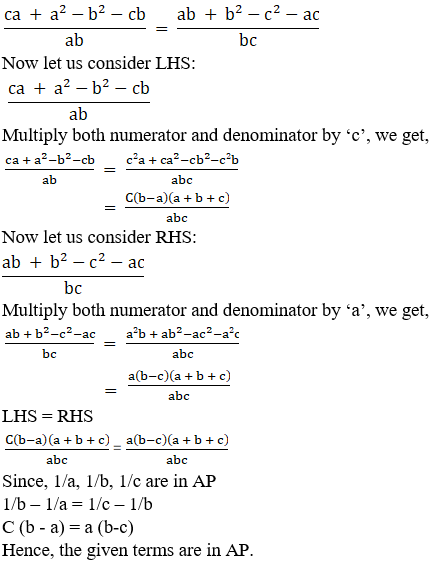Since, 1/a, 1/b, 1/c are in AP

1/b – 1/a = 1/c – 1/b

C (b – a) = a (b-c)

Hence, the given terms are in AP.

(ii) a(b + c), b(c + a), c(a + b) are in A.P.

We know that if, b(c + a) – a(b+c) = c(a+b) – b(c+a)

Consider LHS:

b(c + a) – a(b+c)

Upon simplification we get,

b(c + a) – a(b+c) = bc + ba – ab – ac

= c (b-a)

Now,

c(a+b) – b(c+a) = ca + cb – bc – ba

= a (c-b)

We know,

1/a, 1/b, 1/c are in AP

So, 1/a – 1/b = 1/b – 1/c

Or c(b-a) = a(c-b)

Hence, given terms are in AP.

2. If a2, b2, c2 are in AP., prove that a/(b+c), b/(c+a), c/(a+b) are in AP.

Solution:

If a2, b2, c2 are in AP then, b2 – a2 = c2 – b2

If a/(b+c), b/(c+a), c/(a+b) are in AP then,

b/(c+a) – a/(b+c) = c/(a+b) – b/(c+a)

Let us take LCM on both the sides we get,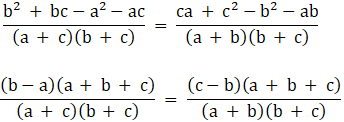Since, b2 – a2 = c2 – b2

Substituting b2 – a2= c2 – b2 in above, we get

LHS = RHS

Hence, given terms are in AP

3. If a, b, c are in A.P., then show that:
(i) a2(b + c), b2(c + a), c2(a + b) are also in A.P.
(ii) b + c – a, c + a – b, a + b – c are in A.P.
(iii) bc – a2, ca – b2, ab – c2 are in A.P.

Solution:

(i) a2(b + c), b2(c + a), c2(a + b) are also in A.P.

If b2(c + a) – a2(b + c) = c2(a + b) – b2(c + a)

b2c + b2a – a2b – a2c = c2a + c2b – b2a – b2c

Given, b – a = c – b

And since a, b, c are in AP,

c(b2 – a2 ) + ab(b – a) = a(c2 – b2 ) + bc(c – b)

(b – a) (ab + bc + ca) = (c – b) (ab + bc + ca)

Upon cancelling, ab + bc + ca from both sides

b – a = c – b

2b = c + a [which is true]

Hence, given terms are in AP

(ii) b + c – a, c + a – b, a + b – c are in A.P.

If (c + a – b) – (b + c – a) = (a + b – c) – (c + a – b)

Then, b + c – a, c + a – b, a + b – c are in A.P.

Let us consider LHS and RHS

(c + a – b) – (b + c – a) = (a + b – c) – (c + a – b)

2a – 2b = 2b – 2c

b – a = c – b

And since a, b, c are in AP,

b – a = c – b

Hence, given terms are in AP.

(iii) bc – a2, ca – b2, ab – c2 are in A.P.

If (ca – b2) – (bc – a2) = (ab – c2) – (ca – b2)

Then, bc – a2, ca – b2, ab – c2 are in A.P.

Let us consider LHS and RHS

(ca – b2) – (bc – a2) = (ab – c2) – (ca – b2)

(a – b2 – bc + a2) = (ab – c2 – ca + b2)

(a – b) (a + b + c) = (b – c) (a + b + c)

a – b = b – c

And since a, b, c are in AP,

b – c = a – b

Hence, given terms are in AP

4. If (b+c)/a, (c+a)/b, (a+b)/c are in AP., prove that:

(i) 1/a, 1/b, 1/c are in AP

(ii) bc, ca, ab are in AP

Solution:

(i) 1/a, 1/b, 1/c are in AP

If 1/a, 1/b, 1/c are in AP then,

1/b – 1/a = 1/c – 1/b

Let us consider LHS:

1/b – 1/a = (a-b)/ab

= c(a-b)/abc [by multiplying with ‘c’ on both the numerator and denominator]

Let us consider RHS:

1/c – 1/b = (b-c)/bc

= a(b-c)/bc [by multiplying with ‘a’ on both the numerator and denominator]

Since, (b+c)/a, (c+a)/b, (a+b)/c are in AP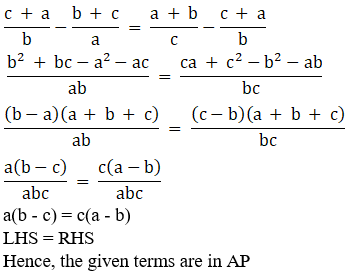(ii) bc, ca, ab are in AP

If bc, ca, ab are in AP then,

ca – bc = ab – ca

c (a-b) = a (b-c)

If 1/a, 1/b, 1/c are in AP then,

1/b – 1/a = 1/c – 1/b

c (a-b) = a (b-c)

Hence, the given terms are in AP

5. If a, b, c are in A.P., prove that:
(i) (a – c)2 = 4 (a – b) (b – c)

(ii) a2 + c2 + 4ac = 2 (ab + bc + ca)

(iii) a3 + c3 + 6abc = 8b3

Solution:

(i) (a – c)2 = 4 (a – b) (b – c)

Let us expand the above expression

a2 + c2 – 2ac = 4(ab – ac – b2 + bc)

a2 + 4c2b2 + 2ac – 4ab – 4bc = 0

(a + c – 2b)2 = 0

a + c – 2b = 0

Since a, b, c are in AP

b – a = c – b

a + c – 2b = 0

a + c = 2b

Hence, (a – c)2 = 4 (a – b) (b – c)

(ii) a2 + c2 + 4ac = 2 (ab + bc + ca)

Let us expand the above expression

a2 + c2 + 4ac = 2 (ab + bc + ca)

a2 + c2 + 2ac – 2ab – 2bc = 0

(a + c – b)2 – b2 = 0

a + c – b = b

a + c – 2b = 0

2b = a+c

b = (a+c)/2

Since a, b, c are in AP

b – a = c – b

b = (a+c)/2

Hence, a2 + c2 + 4ac = 2 (ab + bc + ca)

(iii) a3 + c3 + 6abc = 8b3

Let us expand the above expression

a3 + c3 + 6abc = 8b3

a3 + c3 – (2b)3 + 6abc = 0

a3 + (-2b)3 + c3 + 3a(-2b)c = 0

Since, if a + b + c = 0, a3 + b3 + c3 = 3abc

(a – 2b + c)3 = 0

a – 2b + c = 0

a + c = 2b

b = (a+c)/2

Since a, b, c are in AP

a – b = c – b

b = (a+c)/2

Hence, a3 + c3 + 6abc = 8b3

6. If a(1/b + 1/c), b(1/c + 1/a), c(1/a + 1/b) are in AP., prove that a, b, c are in AP.

Solution:

Here, we know a(1/b + 1/c), b(1/c + 1/a), c(1/a + 1/b) are in AP

Also, a(1/b + 1/c) + 1, b(1/c + 1/a) + 1, c(1/a + 1/b) + 1 are in AP

Let us take LCM for each expression then we get,

(ac+ab+bc)/bc , (ab+bc+ac)/ac, (cb+ac+ab)/ab are in AP

1/bc, 1/ac, 1/ab are in AP

Let us multiply numerator with ‘abc’, we get

abc/bc, abc/ac, abc/ab are in AP

∴ a, b, c are in AP.

Hence proved.

7. Show that x2 + xy + y2, z2 + zx + x2 and y2 + yz + z2 are in consecutive terms of an A.P., if x, y and z are in A.P.

Solution:

x, y, z are in AP

Given, x2 + xy + y2, z2 + zx + x2 and y2 + yz + z2 are in AP

(z2 + zx + x2) – (x2 + xy + y2) = (y2 + yz + z2) – (z2 + zx + x2)

Let d = common difference,

So, Y = x + d and x = x + 2d

Let us consider the LHS:

(z2 + zx + x2) – (x2 + xy + y2)

z2 + zx – xy – y2

(x + 2d)2 + (x + 2d)x – x(x + d) – (x + d)2

x2 + 4xd + 4d2 + x2 + 2xd – x2 – xd – x2 – 2xd – d2

3xd + 3d2

Now, let us consider RHS:

(y2 + yz + z2) – (z2 + zx + x2)

y2 + yz – zx – x2

(x + d)2 + (x + d)(x + 2d) – (x + 2d)x – x2

x2 + 2dx + d2 + x2 + 2dx + xd + 2d2 – x2 – 2dx – x2

3xd + 3d2

LHS = RHS

∴ x2 + xy + y2, z2 + zx + x2 and y2 + yz + z2 are in consecutive terms of A.P

Hence proved.

EXERCISE 19.6 PAGE NO: 19.46

1. Find the A.M. between:
(i) 7 and 13 (ii) 12 and – 8 (iii) (x – y) and (x + y)

Solution:

(i) Let A be the Arithmetic mean

Then 7, A, 13 are in AP

Now, let us solve

A-7 = 13-A

2A = 13 + 7

A = 10

(ii) Let A be the Arithmetic mean

Then 12, A, – 8 are in AP

Now, let us solve

A – 12 = – 8 – A

2A = 12 + 8

A = 2

(iii) Let A be the Arithmetic mean

Then x – y, A, x + y are in AP

Now, let us solve

A – (x – y) = (x + y) – A

2A = x + y + x – y

A = x

2. Insert 4 A.M.s between 4 and 19.

Solution:

Let A1, A2, A3, A4 be the 4 AM Between 4 and 19

Then, 4, A1, A2, A3, A4, 19 are in AP.

By using the formula,

d = (b-a) / (n+1)

= (19 – 4) / (4 + 1)

= 15/5

= 3

So,

A1 = a + d = 4 + 3 = 7

A2 = A1 + d = 7 + 3 = 10

A3 = A2 + d = 10 + 3 = 13

A4 = A3 + d = 13 + 3 = 16

3. Insert 7 A.M.s between 2 and 17.

Solution:

Let A1, A2, A3, A4, A5, A6, A7 be the 7 AMs between 2 and 17

Then, 2, A1, A2, A3, A4, A5, A6, A7, 17 are in AP

By using the formula,

an = a + (n – 1)d

an = 17, a = 2, n = 9

so,

17 = 2 + (9 – 1)d

17 = 2 + 9d – d

17 = 2 + 8d

8d = 17 – 2

8d = 15

d = 15/8

So,

A1 = a + d = 2 + 15/8 = 31/8

A2 = A1 + d = 31/8 + 15/8 = 46/8

A3 = A2 + d = 46/8 + 15/8 = 61/8

A4 = A3 + d = 61/8 + 15/8 = 76/8

A5 = A4 + d = 76/8 + 15/8 = 91/8

A6 = A5 + d = 91/8 + 15/8 = 106/8

A7 = A6 + d = 106/8 + 15/8 = 121/8

∴ the 7 AMs between 2 and 7 are 31/8, 46/8, 61/8, 76/8, 91/8, 106/8, 121/8

4. Insert six A.M.s between 15 and – 13.

Solution:

Let A1, A2, A3, A4, A5, A6 be the 7 AM between 15 and – 13

Then, 15, A1, A2, A3, A4, A5, A6, – 13 are in AP

By using the formula,

an = a + (n – 1)d

an = -13, a = 15, n = 8

so,

-13 = 15 + (8 – 1)d

-13 = 15 + 7d

7d = -13 – 15

7d = -28

d = -4

So,

A1 = a + d = 15 – 4 = 11

A2 = A1 + d = 11 – 4 = 7

A3 = A2 + d = 7 – 4 = 3

A4 = A3 + d = 3 – 4 = -1

A5 = A4 + d = -1 – 4 = -5

A6 = A5 + d = -5 – 4 = -9

5. There are n A.M.s between 3 and 17. The ratio of the last mean to the first mean is 3: 1. Find the value of n.

Solution:

Let the series be 3, A1, A2, A3, …….., An, 17

Given, an/a1 = 3/1

We know total terms in AP are n + 2

So, 17 is the (n + 2)th term

By using the formula,

An = a + (n – 1)d

An = 17, a = 3

So, 17 = 3 + (n + 2 – 1)d

17 = 3 + (n + 1)d

17 – 3 = (n + 1)d

14 = (n + 1)d

d = 14/(n+1)

Now,

An = 3 + 14/(n+1) = (17n + 3) / (n+1)

A1 = 3 + d = (3n+17)/(n+1)

Since,

an/a1 = 3/1

(17n + 3)/ (3n+17) = 3/1

17n + 3 = 3(3n + 17)

17n + 3 = 9n + 51

17n – 9n = 51 – 3

8n = 48

n = 48/8

= 6

∴ There are 6 terms in the AP

6. Insert A.M.s between 7 and 71 in such a way that the 5th A.M. is 27. Find the number of A.M.s.

Solution:

Let the series be 7, A1, A2, A3, …….., An, 71

We know total terms in AP are n + 2

So 71 is the (n + 2)th term

By using the formula,

An = a + (n – 1)d

An = 71, n = 6

A6 = a + (6 – 1)d

a + 5d = 27 (5th term)

d = 4

so,

71 = (n + 2)th term

71 = a + (n + 2 – 1)d

71 = 7 + n(4)

n = 15

∴ There are 15 terms in AP

7. If n A.M.s are inserted between two numbers, prove that the sum of the means equidistant from the beginning and the end is constant.

Solution:

Let a and b be the first and last terms

The series be a, A1, A2, A3, …….., An, b

We know, Mean = (a+b)/2

Mean of A1 and An = (A1 + An)/2

A1 = a+d

An = a – d

So, AM = (a+d+b-d)/2

= (a+b)/2

AM between A2 and An-1 = (a+2d+b-2d)/2

= (a+b)/2

Similarly, (a + b)/2 is constant for all such numbers

Hence, AM = (a + b)/2

8. If x, y, z are in A.P. and A1is the A.M. of x and y, and A2 is the A.M. of y and z, then prove that the A.M. of A1 and A2 is y.

Solution:

Given that,

A1 = AM of x and y

And A2 = AM of y and z

So, A1 = (x+y)/2

A2 = (y+x)/2

AM of A1 and A2 = (A1 + A2)/2

= [(x+y)/2 + (y+z)/2]/2

= [x+y+y+z]/2

= [x+2y+z]/2

Since x, y, z are in AP, y = (x+z)/2

AM = [(x + z/2) + (2y/2)]/2

= (y + y)/2

= 2y/2

= y

Hence proved.

9. Insert five numbers between 8 and 26 such that the resulting sequence is an A.P

Solution:

Let A1, A2, A3, A4, A5 be the 5 numbers between 8 and 26

Then, 8, A1, A2, A3, A4, A5, 26 are in AP

By using the formula,

An = a + (n – 1)d

An = 26, a = 8, n = 7

26 = 8 + (7 – 1)d

26 = 8 + 6d

6d = 26 – 8

6d = 18

d = 18/6

= 3

So,

A1 = a + d = 8 + 3 = 11

A2 = A1 + d = 11 + 3 = 14

A3 = A2 + d = 14 + 3 = 17

A4 = A3 + d = 17 + 3 = 20

A5 = A4 + d = 20 + 3 = 23

EXERCISE 19.7 PAGE NO: 19.48

1. A man saved ₹ 16500 in ten years. In each year after the first he saved ₹ 100/- more than he did in the preceding year. How much did he saved in the first year?

Solution:

Given: A man saved ₹16500 in ten years

Let ₹ x be his savings in the first year

His savings increased by ₹ 100 every year.

So,

A.P will be x, 100 + x, 200 + x………………..

Where, x is first term and

Common difference, d = 100 + x – x = 100

We know, Sn is the sum of n terms of an A.P

By using the formula,

Sn = n/2 [2a + (n – 1)d]

where, a is first term, d is common difference and n is number of terms in an A.P.

Given:

Sn = 16500 and n = 10

S10 = 10/2 [2x + (10 – 1)100]

16500 = 5{2x + 9(100)}

16500 = 5(2x + 900)

16500 = 10x + 4500

-10x = 4500 – 16500

–10x = –12000

x = 12000/10

= 1200

Hence, his saving in the first year is ₹ 1200.

2. A man saves ₹ 32 during the first year, ₹ 36 in the second year and in this way he increases his savings by ₹ 4 every year. Find in what time his saving will be ₹ 200.

Solution:

Given:

First year savings is ₹ 32

Second year savings is ₹ 36

In this process he increases his savings by ₹ 4 every year

Then,

A.P. will be 32, 36, 40,………

Where, 32 is first term and common difference, d = 36 – 32 = 4

We know, Sn is the sum of n terms of an A.P

By using the formula,

Sn = n/2 [2a + (n – 1)d]

where, a is first term, d is common difference and n is number of terms in an A.P.

Given:

Sn = 200, a = 32, d = 4

Sn = n/2 [2a + (n – 1)d]

200 = n/2 [2(32) + (n-1)4]

200 = n/2 [64 + 4n – 4]

400 = n [60 + 4n]

400 = 4n [15 + n]

400/4 = n [15 + n]

100 = 15n + n2

n2 + 15n – 100 = 0

n2 + 20n – 5n – 100 = 0

n (n + 20) – 5 (n + 20) = 0

(n + 20) – 5 (n + 20) = 0

(n + 20) (n – 5) = 0

n = -20 or 5

n = 5 [Since, n is a positive integer]

Hence, the man requires 5 days to save ₹ 200

3. A man arranges to pay off a debt of ₹ 3600 by 40 annual instalments which form an arithmetic series. When 30 of the instalments are paid, he dies leaving one-third of the debt unpaid, find the value of the instalment.

Solution:

Given:

40 annual instalments which form an arithmetic series.

Let the first instalment be ‘a’

S40 = 3600, n = 40

By using the formula,

Sn = n/2 [2a + (n – 1)d]

3600 = 40/2 [2a + (40-1)d]

3600 = 20 [2a + 39d]

3600/20 = 2a + 39d

2a + 39d – 180 = 0 ….. (i)

Given:

Sum of first 30 terms is paid and one third of debt is unpaid.

So, paid amount = 2/3 × 3600 = ₹ 2400

Sn = 2400, n = 30

By using the formula,

Sn = n/2 [2a + (n – 1)d]

2400 = 30/2 [2a + (30-1)d]

2400 = 15 [2a + 29d]

2400/15 = 2a + 29d

2a + 29d -160 = 0 …. (ii)

Now, let us solve equation (i) and (ii) by substitution method, we get

2a + 39d = 180

2a = 180 – 39d … (iii)

Substitute the value of 2a in equation (ii)

2a + 29d – 160 = 0

180 – 39d + 29d – 160 = 0

20 – 10d = 0

10d = 20

d = 20/10

= 2

Substitute the value of d in equation (iii)

2a = 180 – 39d

2a = 180 – 39(2)

2a = 180 – 78

2a = 102

a = 102/2

= 51

Hence, value of first installment ‘a’ is ₹ 51

4. A manufacturer of the radio sets produced 600 units in the third year and 700 units in the seventh year. Assuming that the product increases uniformly by a fixed number every year, find
(і) the production in the first year
(іі) the total product in the 7 years and
(ііі) the product in the 10th year.

Solution:

Given:

600 and 700 radio sets units are produced in third and seventh year respectively.

a3 = 600 and a7 = 700

(і) The production in the first year

We need to find the production in the first year.

Let first year production be ‘a’

So the AP formed is, a, a+x, a+2x, ….

By using the formula,

an = a + (n-1)d

a3 = a + (3-1)d

600 = a + 2d …. (i)

a7 = a + (7-1)d

700 = a + 6d

a = 700 – 6d…. (ii)

Substitute value of a in (i) we get,

600 = a + 2d

600 = 700 – 6d + 2d

700 – 600 = 4d

100 = 4d

d = 100/4

= 25

Now substitute value of d in (ii) we get,

a = 700 – 6d

= 700 – 6(25)

= 700 – 150

= 550

∴ The production in the first year, ‘a’ is 550

(іі) the total product in the 7 years

We need to find the total product in 7 years i.e. is S7

By using the formula,

Sn = n/2 [2a + (n-1)d]

n = 7, a = 550, d = 25

S7 = 7/2 [2(550) + (7-1)25]

= 7/2 [1100 + 150]

= 7/2 

= 7 

= 4375

∴ The total product in the 7 years is 4375.

(ііі) the product in the 10th year.

We need to find the product in the 10th year i.e. a10

By using the formula,

an = a + (n-1)d

n = 10, a = 550, d = 25

a10 = 550 + (10-1)25

= 550 + (9)25

= 550 + 225

= 775

∴ The product in the 10th year is 775.

5. There are 25 trees at equal distances of 5 meters in a line with a well, the distance of well from the nearest tree being 10 meters. A gardener waters all the trees separately starting from the well and he returns to the well after watering each tree to get water for the next. Find the total distance the gardener will cover in order to water all the trees.

Solution:

Given: total trees are 25 and equal distance between two adjacent trees are 5 meters

We need to find the total distance the gardener will cover.

As gardener is coming back to well after watering every tree:

Distance covered by gardener to water 1st tree and return back to the initial position is 10m + 10m = 20m

Now, distance between adjacent trees is 5m.

Distance covered by him to water 2nd tree and return back to the initial position is 15m + 15m = 30m

Distance covered by the gardener to water 3rd tree return back to the initial position is 20m + 20m = 40m

Hence distance covered by the gardener to water the trees are in A.P

A.P. is 20, 30, 40 ………upto 25 terms

Here, first term, a = 20, common difference, d = 30 – 20 = 10, n = 25

We need to find S25 which will be the total distance covered by gardener to water 25 trees.

So by using the formula,

Sn = n/2 [2a + (n – 1)d]

S25 = 25/2 [2(20) + (25-1)10]

= 25/2 [40 + (24)10]

= 25/2 [40 + 240]

= 25/2 

= 25 

= 3500

∴ The total distance covered by gardener to water trees all 25 trees is 3500m.

6. A man is employed to count ₹ 10710. He counts at the rate of ₹ 180 per minute for half an hour. After this he counts at the rate of ₹ 3 less every minute than the preceding minute. Find the time taken by him to count the entire amount.

Solution:

Given: Amount to be counted is ₹ 10710

We need to find time taken by man to count the entire amount.

He counts at the rate of ₹ 180 per minute for half an hour or 30 minutes.

So, Amount to be counted in an hour = 180 × 30 = ₹ 5400

Amount left = 10710 – 5400 = ₹ 5310

Sn = 5310

After an hour, rate of counting is decreasing at ₹ 3 per minute. This rate will form an A.P.

A.P. is 177, 174, 171,……

Here a = 177 and d = 174 – 177 = –3

By using the formula,

Sn = n/2 [2a + (n – 1)d]

5310 = n/2 [2(177) + (n-1) (-3)]

5310 = n/2 [354 -3n + 3]

5310 × 2 = n [357 – 3n]

10620 = 357n – 3n2

10620 = 3n(119 – n)

10620/3 = n(119 – n)

3540 = 119n – n2

n2 – 119n + 3540 = 0

n2 – 59n – 60n + 3540 = 0

n(n – 59) – 60(n – 59) = 0

(n – 59) (n – 60) = 0

n = 59 or 60

We shall consider value of n = 59. Since, at 60th min he will count ₹ 0

∴ The total time taken by him to count the entire amount = 30 + 59 = 89 minutes.

7. A piece of equipment cost a certain factory ₹ 600,000. If it depreciates in value 15% the first, 13.5% the next year, 12% the third year, and so on. What will be its value at the end of 10 years, all percentages applying to the original cost?

Solution:

Given: A piece of equipment cost a certain factory is ₹ 600,000

We need to find the value of the equipment at the end of 10 years.

The price of equipment depreciates 15%, 13.5%, 12% in 1st, 2nd, 3rd year and so on.

So the A.P. will be 15, 13.5, 12,…………… up to 10 terms

Here, a = 15, d = 13.5 – 15 = –1.5, n = 10

By using the formula,

Sn = n/2 [2a + (n – 1)d]

S10 = 10/2 [2(15) + (10-1) (-1.5)]

= 5 [30 + 9(-1.5)]

= 5 [30 – 13.5]

= 5 [16.5]

= 82.5

The value of equipment at the end of 10 years is = [100 – Depreciation %]/100 × cost

= [100 – 82.5]/100 × 600000

= 175/10 × 6000

= 175 × 600

= 105000

∴ The value of equipment at the end of 10 years is ₹ 105000.

8. A farmer buys a used tractor for ₹ 12000. He pays ₹ 6000 cash and agrees to pay the balance in annual instalments of ₹ 500 plus 12% interest on the unpaid amount. How much the tractor cost him?

Solution:

Given: Price of the tractor is ₹12000.

We need to find the total cost of the tractor if he buys it in installments.

Total price = ₹ 12000

Paid amount = ₹ 6000

Unpaid amount = ₹ 12000 – 6000 = ₹ 6000

He pays remaining ₹ 6000 in ‘n’ number of installments of ₹ 500 each.

So, n = 6000/500 = 12

Cost incurred by him to pay remaining 6000 is

The AP will be:

(500 + 12% of 6000) + (500 + 12% of 5500) + … up to 12 terms

500 × 12 + 12% of (6000 + 5500 + … up to 12 terms)

By using the formula,

Sn = n/2 [2a + (n – 1)d]

n = 12, a = 6000, d = -500

S12 = 500×12 + 12/100 × 12/2 [2(6000) + (12-1) (-500)]

= 6000 + 72/100 [12000 + 11 (-500)]

= 6000 + 72/100 [12000 – 5500]

= 6000 + 72/100 

= 6000 + 4680

= 10680

Total cost = 6000 + 10680

= 16680

∴ The total cost of the tractor if he buys it in installment is ₹ 16680.

### Also, access exercises of RD Sharma Solutions for Class 11 Maths Chapter 19 – Arithmetic Progressions

Exercise 19.1 Solutions

Exercise 19.2 Solutions

Exercise 19.3 Solutions

Exercise 19.4 Solutions

Exercise 19.5 Solutions

Exercise 19.6 Solutions

Exercise 19.7 Solutions

## Frequently Asked Questions on RD Sharma Solutions for Class 11 Maths Chapter 19

### What is the main aim of RD Sharma Solutions for Class 11 Maths Chapter 19?

The students are encouraged to go through RD Sharma Solutions for Class 11 Maths Chapter 19 thoroughly before the final exams to score well and intensify their problem-solving abilities. Understanding the concepts and clearing the doubts immediately during self-study is the main aim of these solutions.

### Why should we refer to RD Sharma Solutions for Class 11 Maths Chapter 19?

Students can refer to these RD Sharma Solutions for Class 11 Maths Chapter 19 which helps in gaining knowledge and for better guidance its best to solve these RD Sharma Solutions. Solving the exercises in this chapter will assure that students can score good marks in the exams.

### What are the key benefits of learning RD Sharma Solutions for Class 11 Maths Chapter 19?

Key benefits of RD Sharma Solutions for Class 11 Maths Chapter 19 are provided here:
1. Students can easily access the solutions for each exercise from the chapters available.
2. The solutions are prepared by an expert team at BYJU’S focusing on speed and accuracy.
3. The chapter contains sample examples which include graphs and illustrations that help students to understand the concepts clearly.